# How to Create Admirable Plots with rpact

Utilities
Sample size
Power
Power simulation
This document provides many different examples for creating plots with rpact and ggplot2.
Author

Friedrich Pahlke and Gernot Wassmer

Published

March 8, 2023

# Preparation

``````library(rpact)
packageVersion("rpact") # version should be version 3.0 or later``````
`` '3.3.4'``

# Design plots

## One-sided design with futility bounds

``````design <- getDesignGroupSequential(
kMax = 3,
typeOfDesign = "OF", sided = 1,
futilityBounds = c(0, 0.1)
)

plot(design, type = 1)``````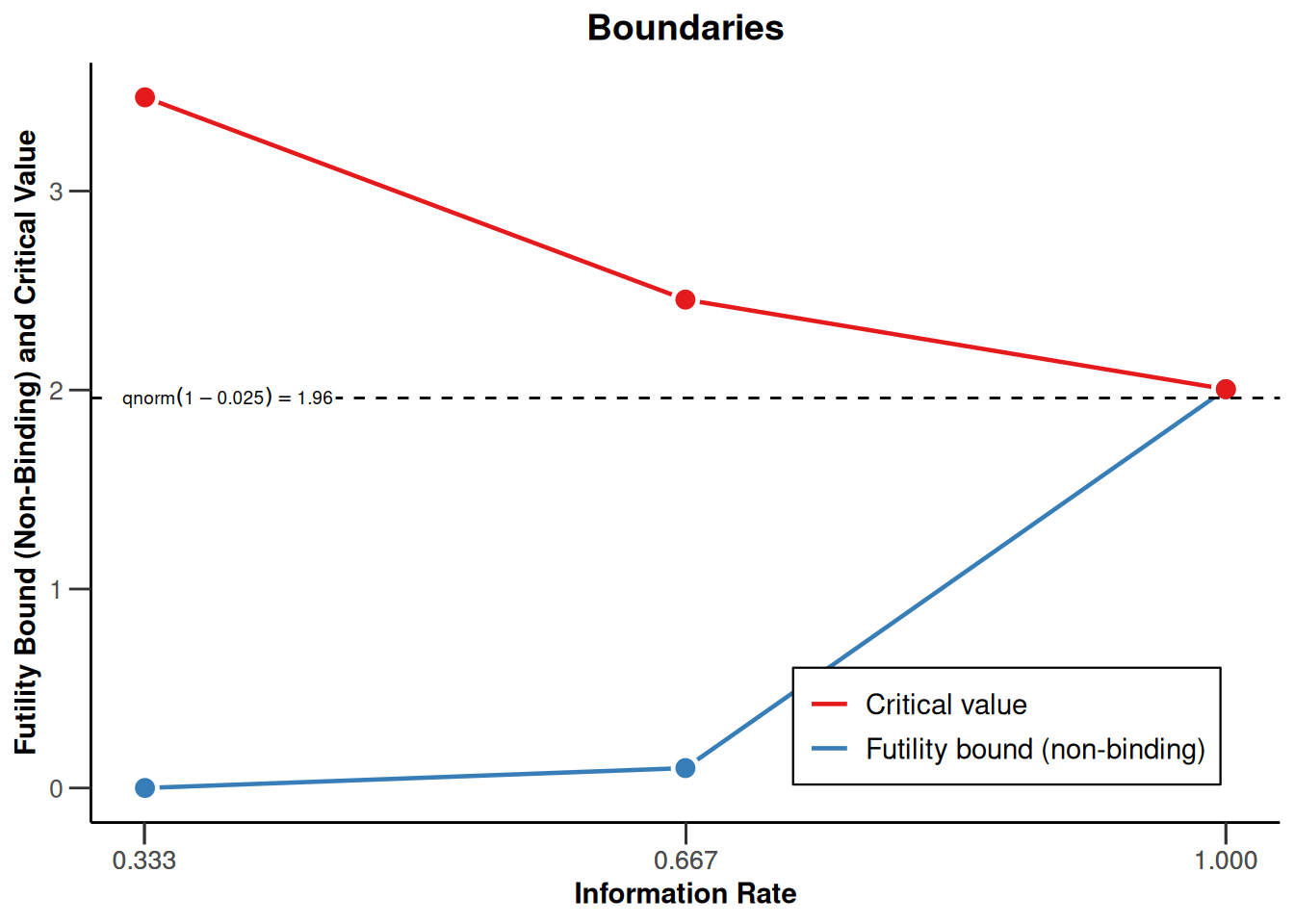``plot(design, type = 5, nMax = 10)``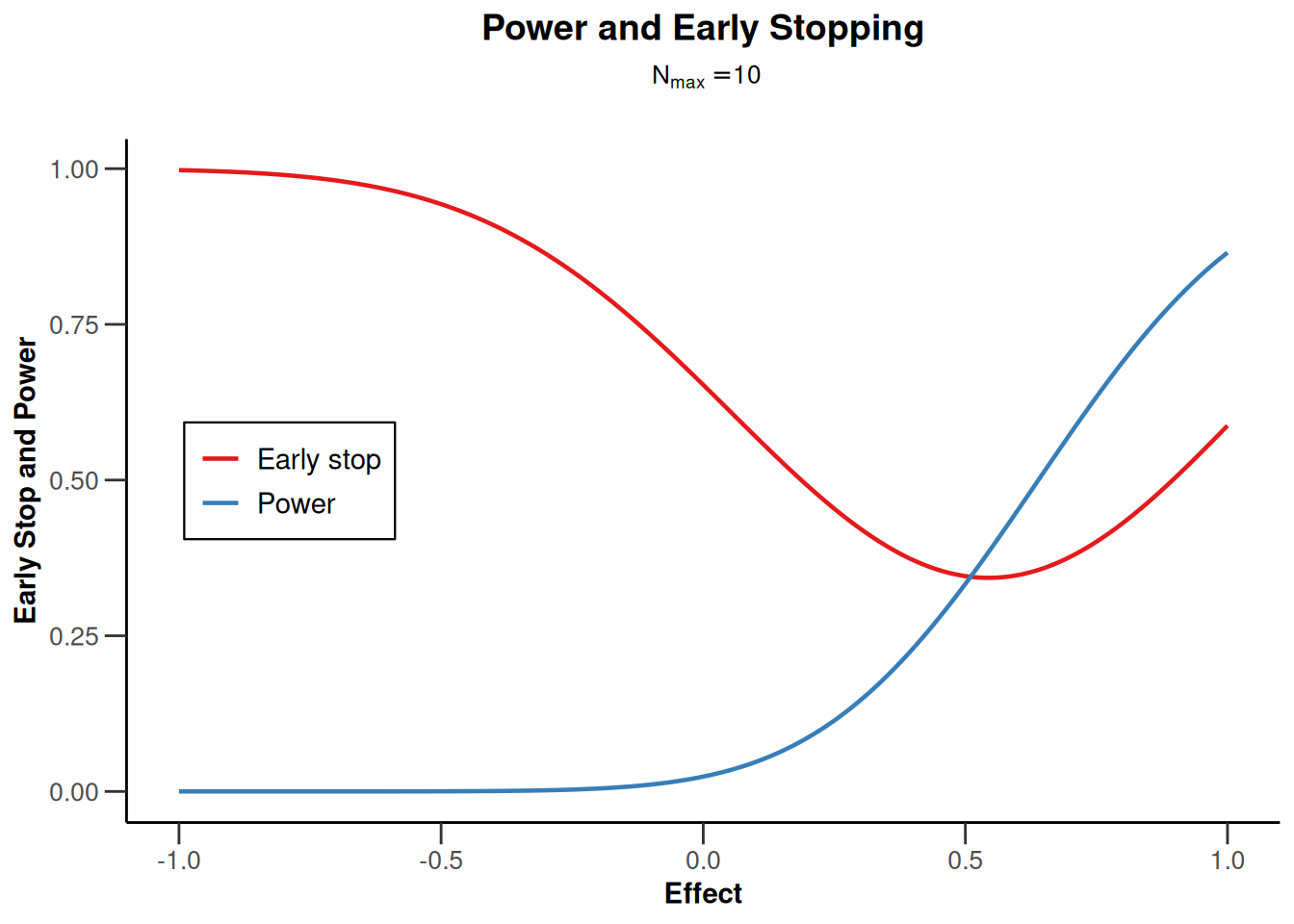``plot(design, type = 6, nMax = 10)``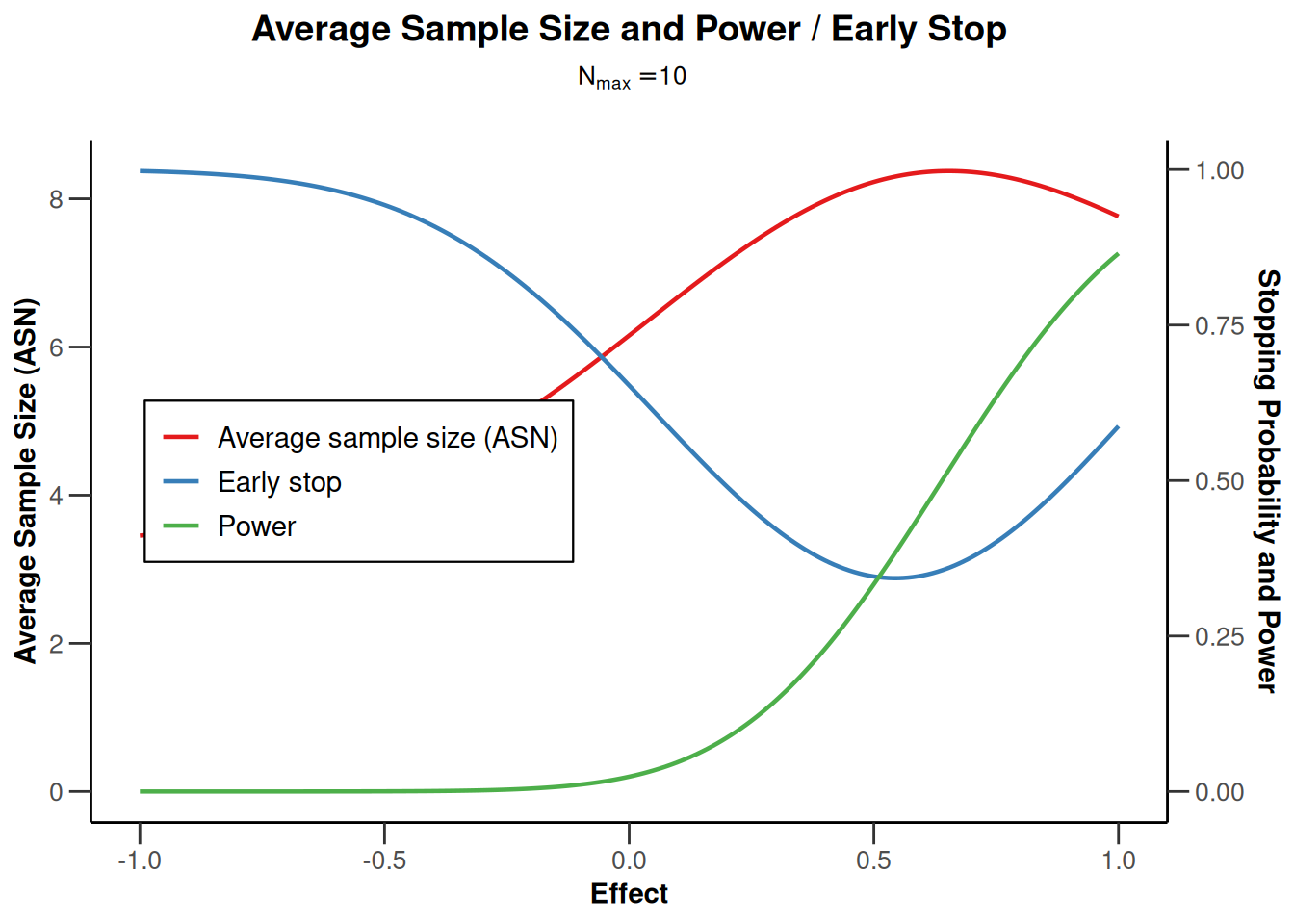## Two-sided design

``````design <- getDesignGroupSequential(
kMax = 4, typeOfDesign = "OF", sided = 2, twoSidedPower = TRUE
)

plot(design, type = 1)``````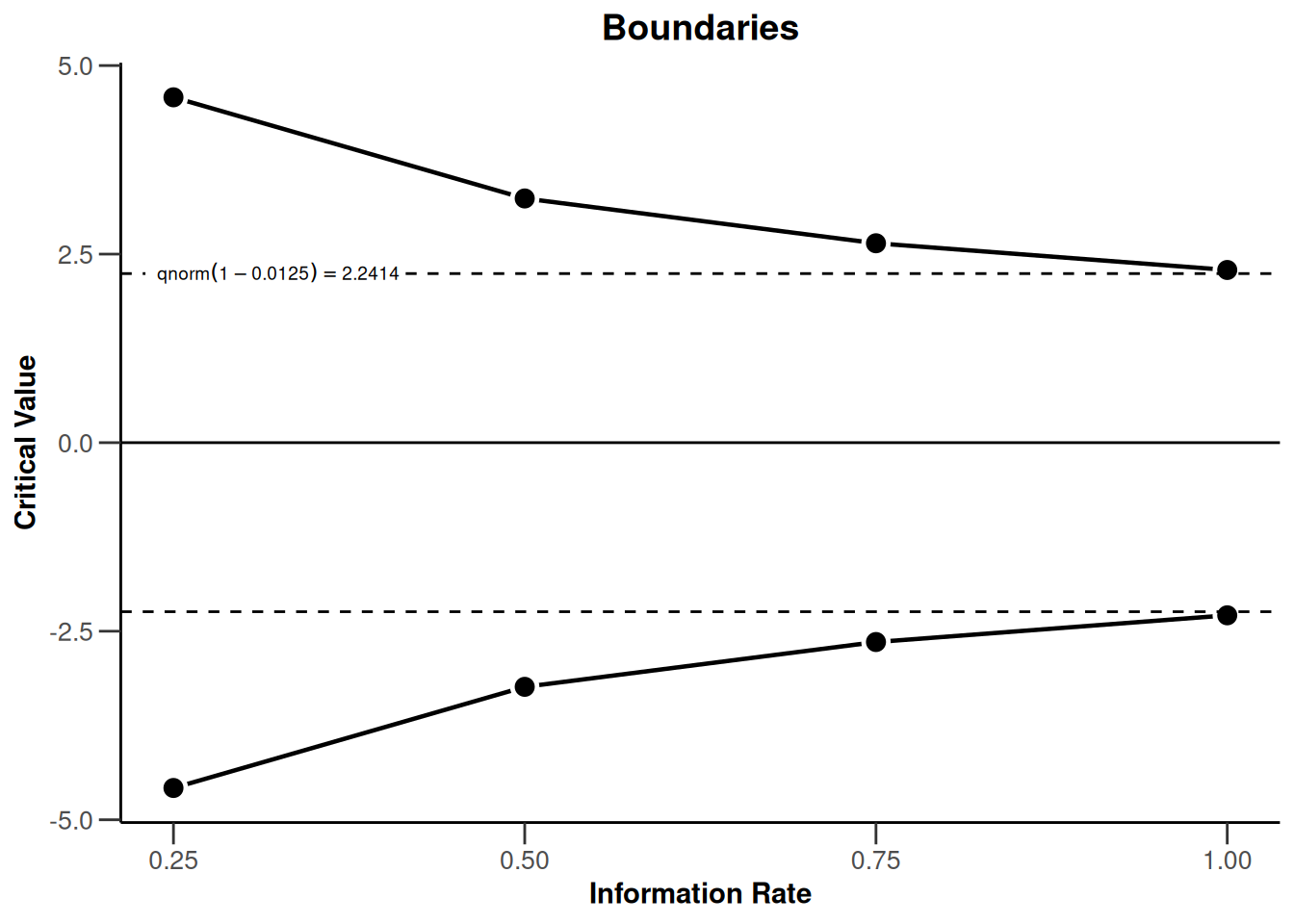``plot(design, type = 5, nMax = 10)````plot(design, type = 6, nMax = 10)``## Two-sided design with futility bound

``````design <- getDesignGroupSequential(
beta = 0.05, kMax = 4, typeOfDesign = "asOF",
typeBetaSpending = "bsOF", sided = 2
)``````
``````Warning: The two-sided beta-spending approach is experimental and hence not
fully validated (see www.rpact.com/experimental)``````
``plot(design, type = 1)``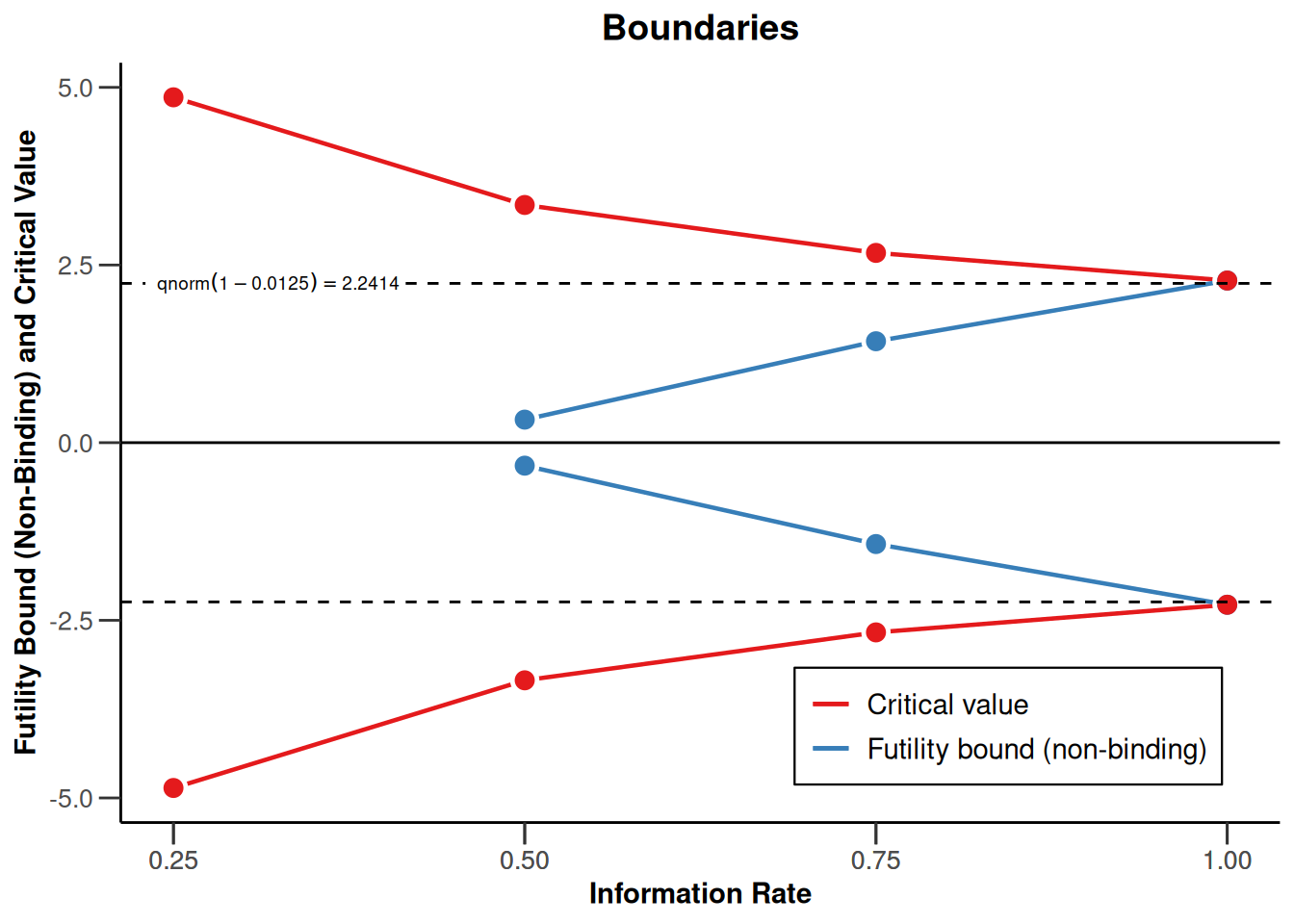``plot(design, type = 5, nMax = 10)``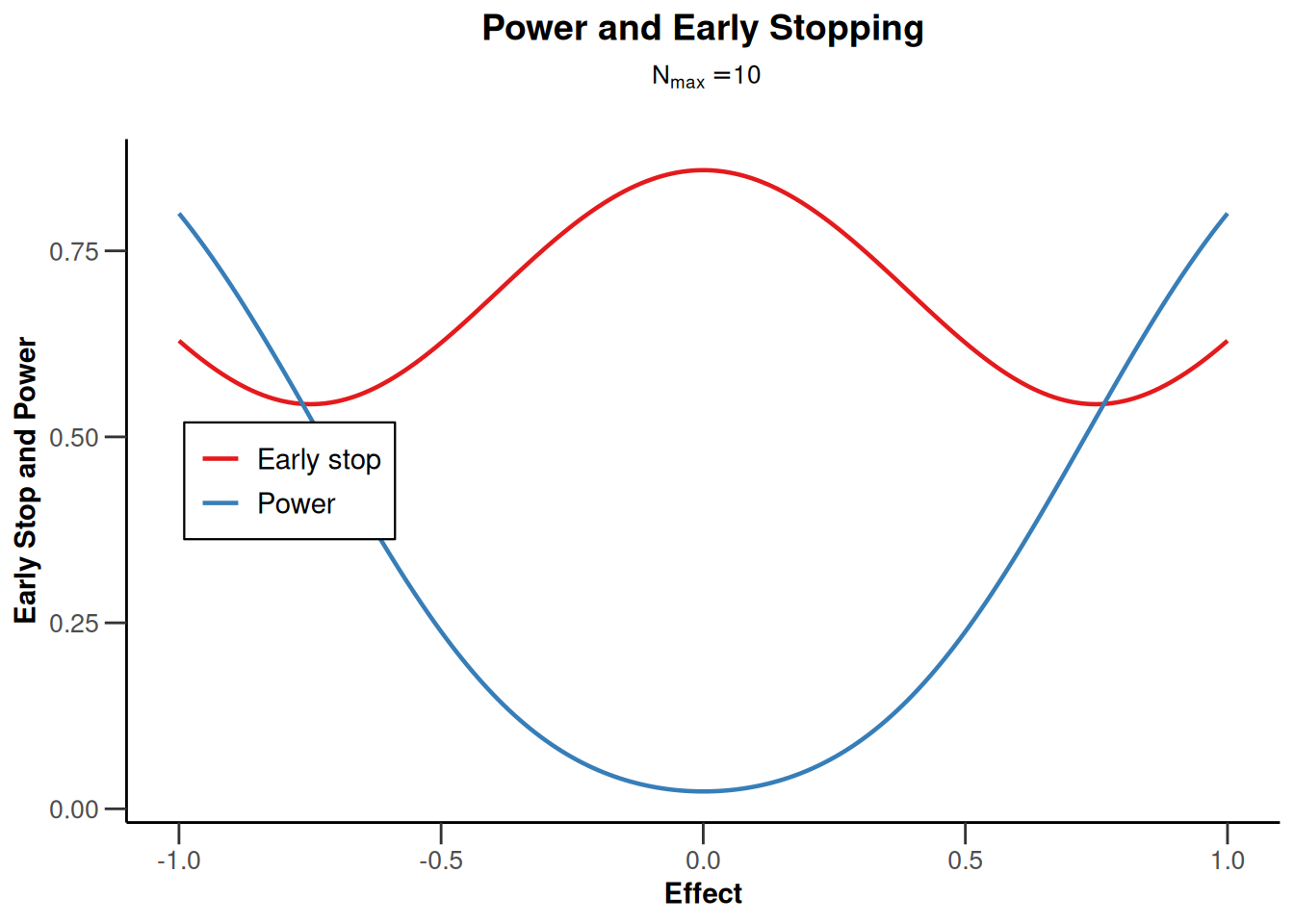``plot(design, type = 6, nMax = 10)``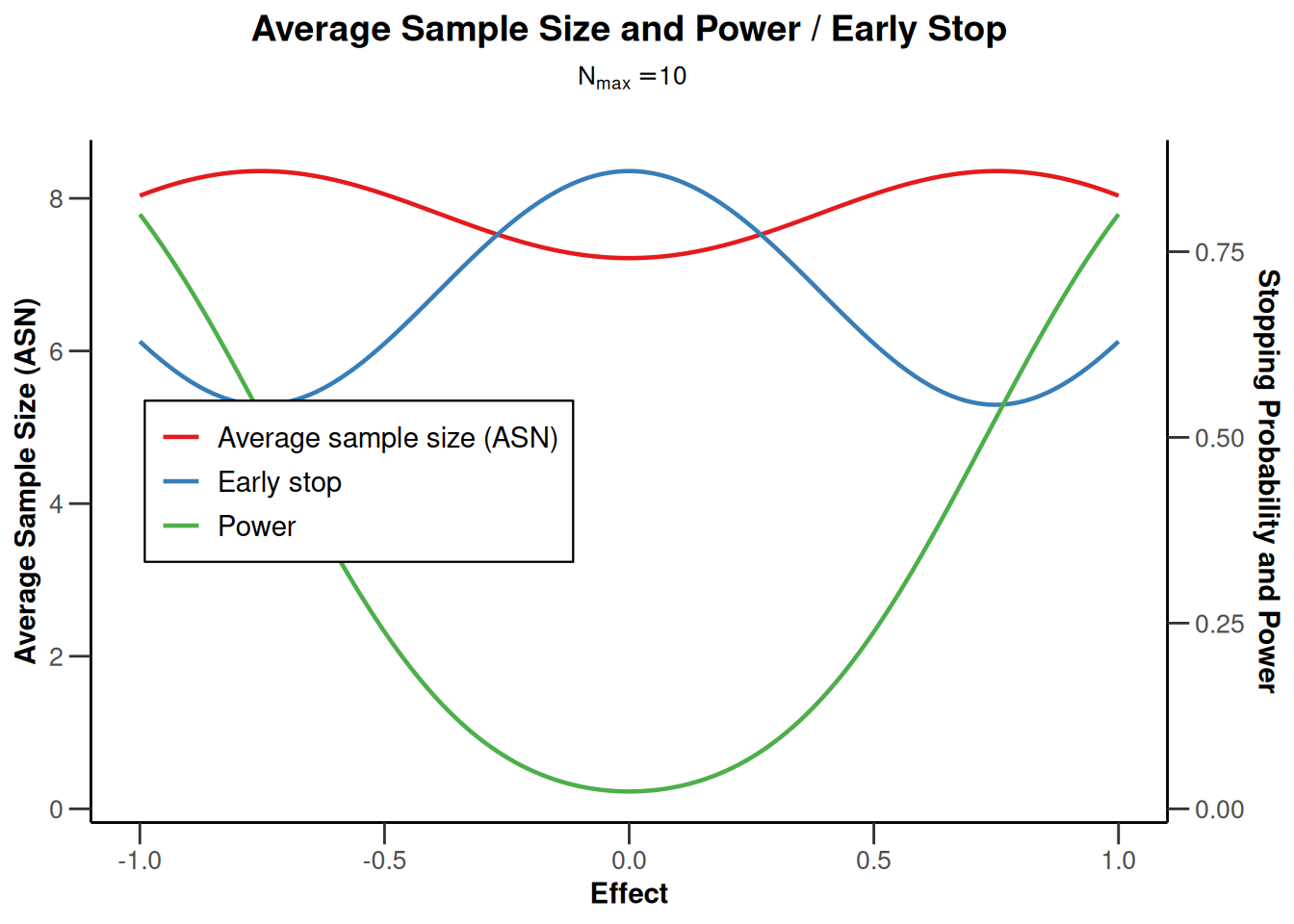# Sample size plots

## Sample size means (continuous endpoint)

### Sample size means for a one-sided design with futility bounds

``````sampleSizeMeans1 <- getSampleSizeMeans(
getDesignGroupSequential(
sided = 1,
futilityBounds = c(0, 0.2)
)
)

plot(sampleSizeMeans1, type = 1)``````
``Warning: Only the first 'alternative' (0.2) was used for plotting``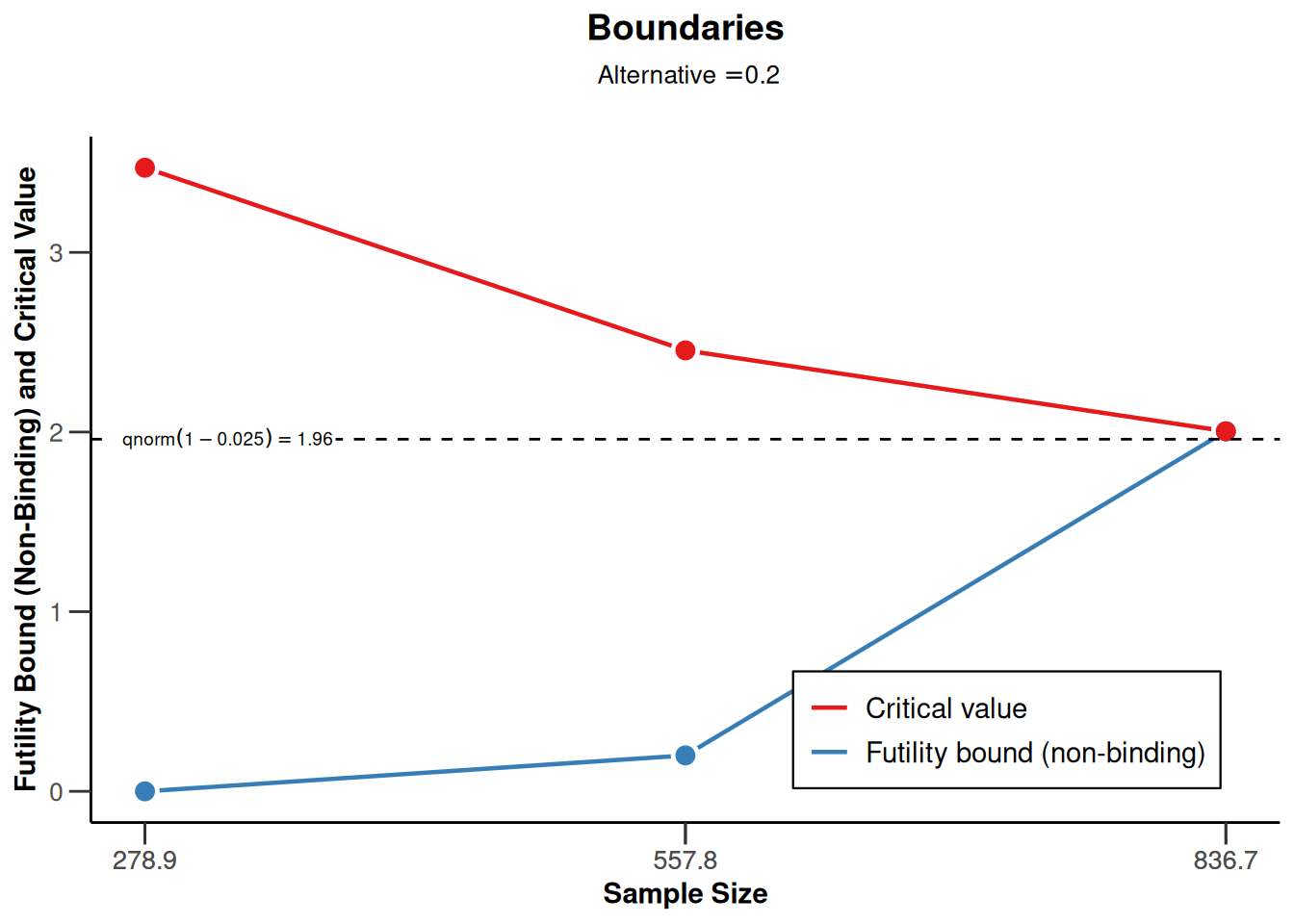``plot(sampleSizeMeans1, type = 2)``
``Warning: Only the first 'alternative' (0.2) was used for plotting``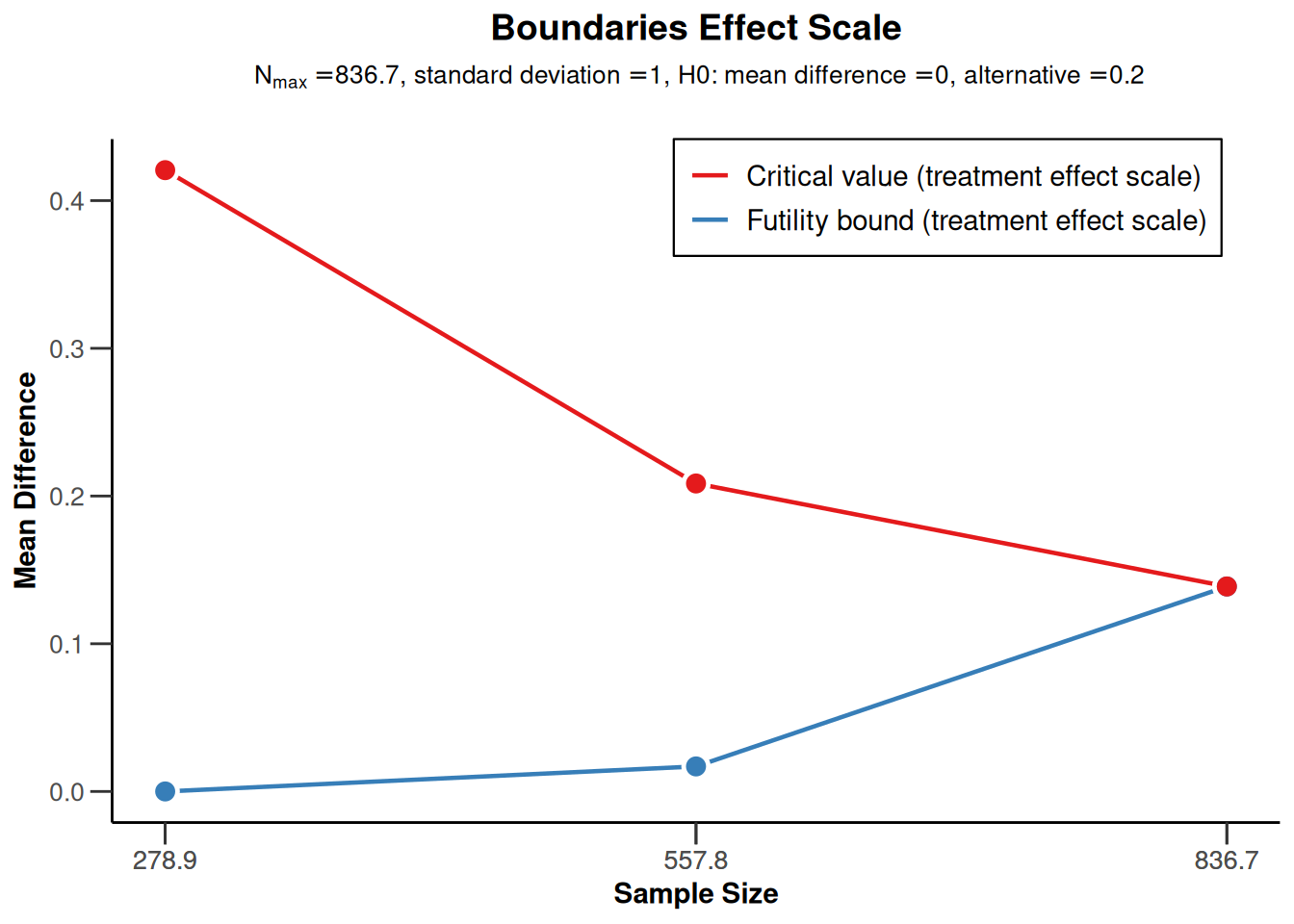### Sample size means for a two-sided design

``````sampleSizeMeans2 <- getSampleSizeMeans(
getDesignGroupSequential(sided = 2)
)

plot(sampleSizeMeans2, type = 1)``````
``Warning: Only the first 'alternative' (0.2) was used for plotting``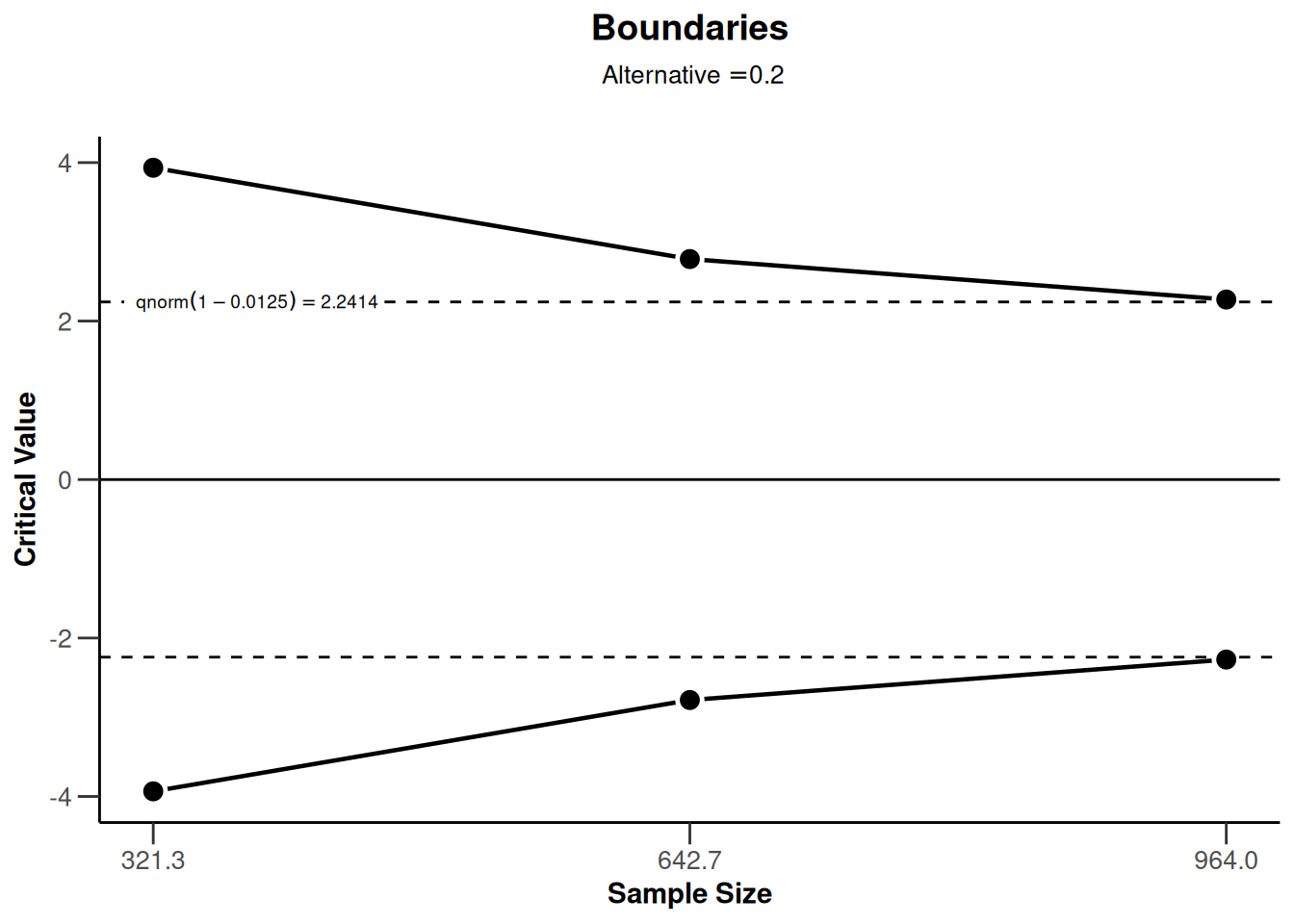``plot(sampleSizeMeans2, type = 2)``
``Warning: Only the first 'alternative' (0.2) was used for plotting``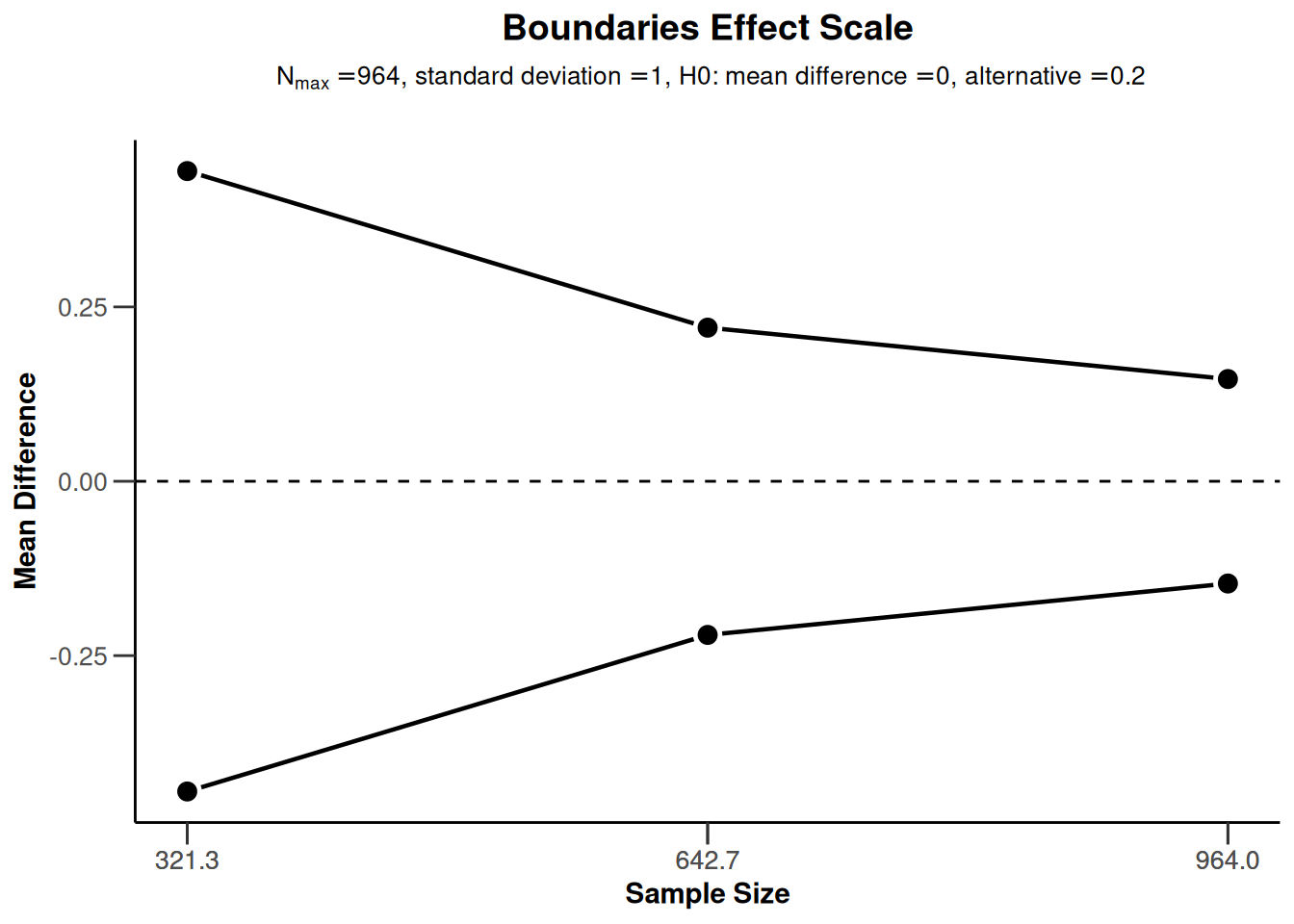## Sample size rates (binary endpoint)

### Sample size rates for a one-sided design with futility bounds

``````sampleSizeRates1 <- getSampleSizeRates(
getDesignGroupSequential(
sided = 1,
futilityBounds = c(0, 0.1)
)
)

plot(sampleSizeRates1, type = 1)``````
``Warning: Only the first 'pi1' (0.4) was used for plotting``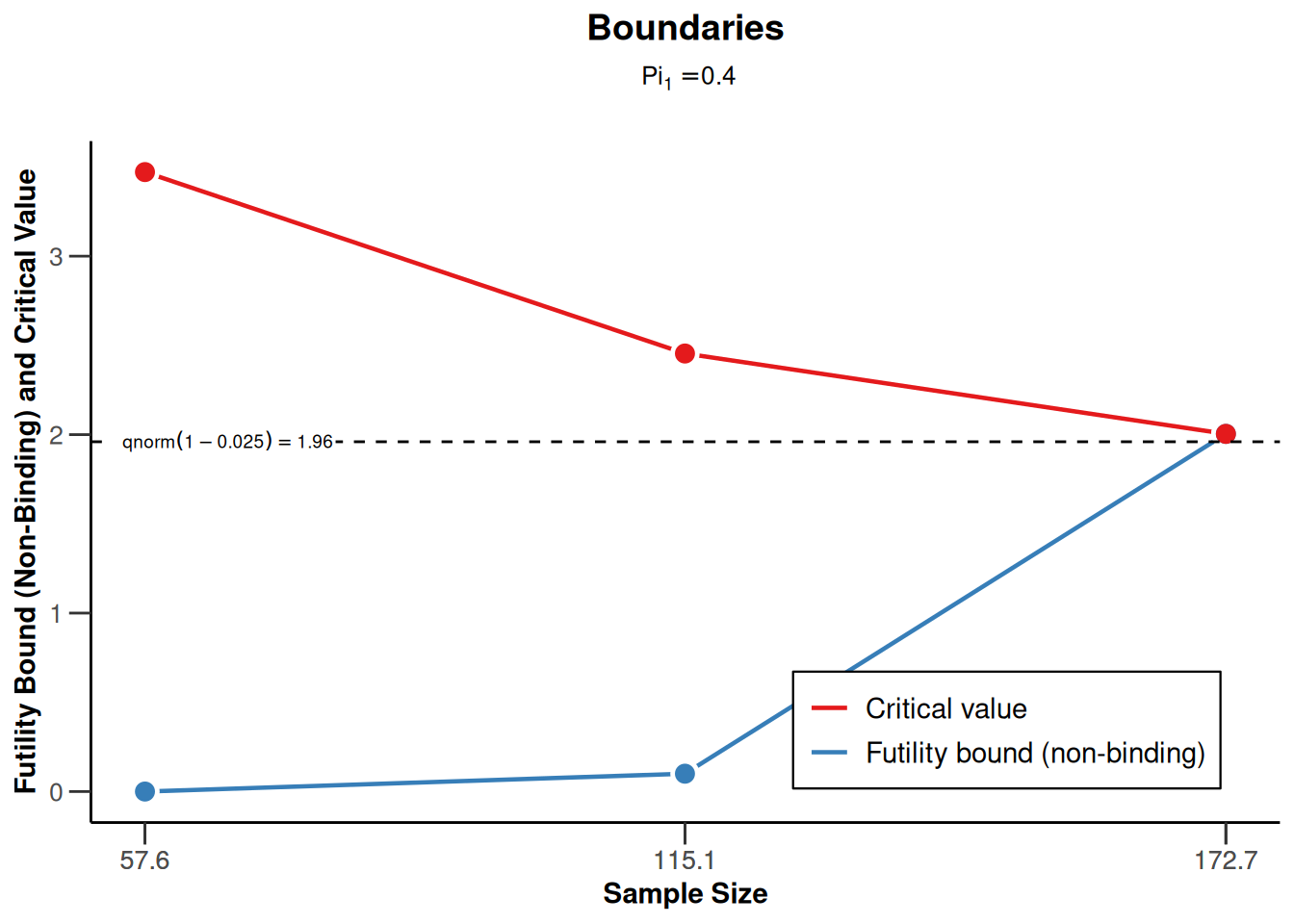``plot(sampleSizeRates1, type = 2)``
``Warning: Only the first 'pi1' (0.4) was used for plotting``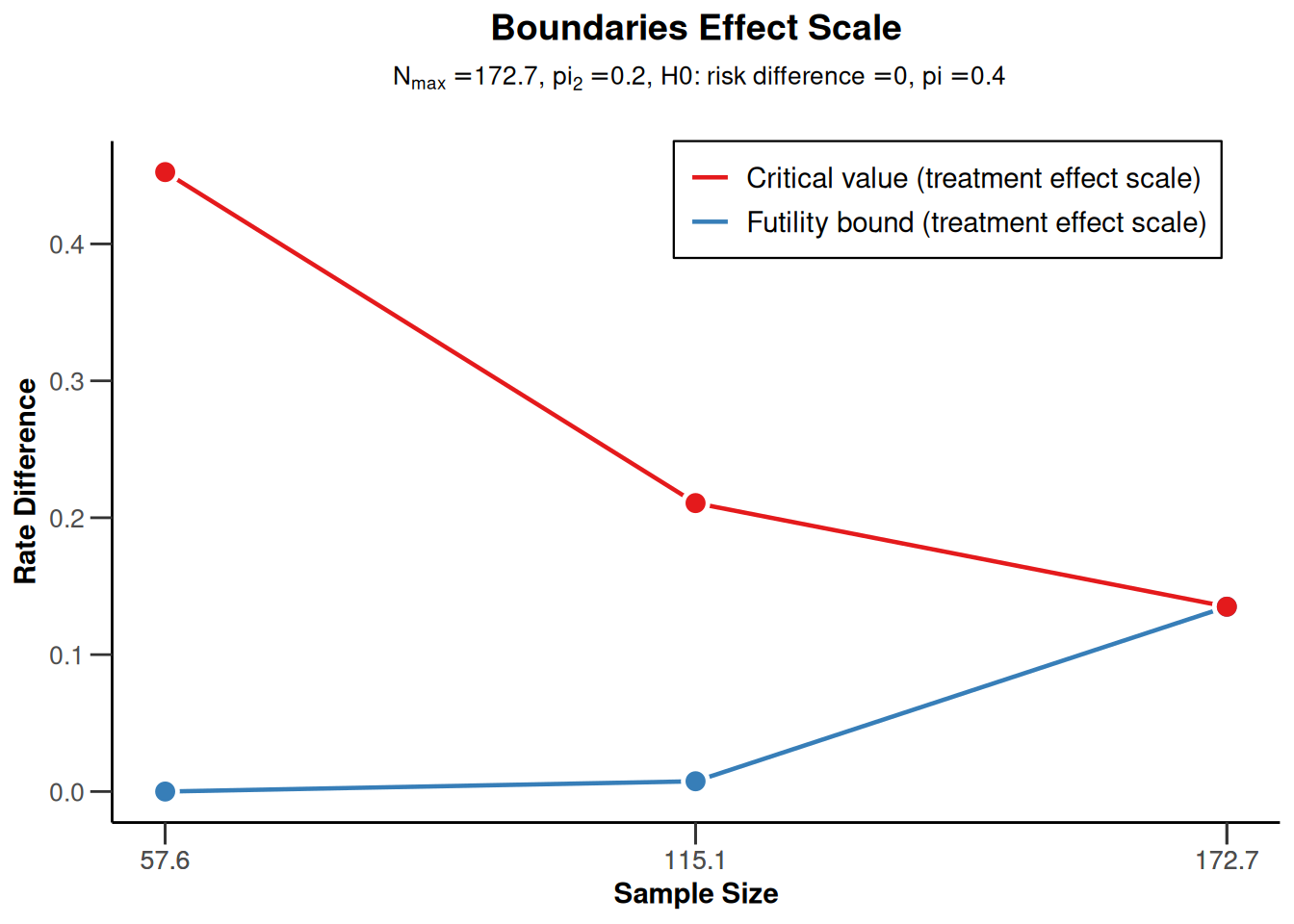### Sample size rates for a two-sided design

``````sampleSizeRates2 <- getSampleSizeRates(
getDesignGroupSequential(sided = 2)
)

plot(sampleSizeRates2, type = 1)``````
``Warning: Only the first 'pi1' (0.4) was used for plotting``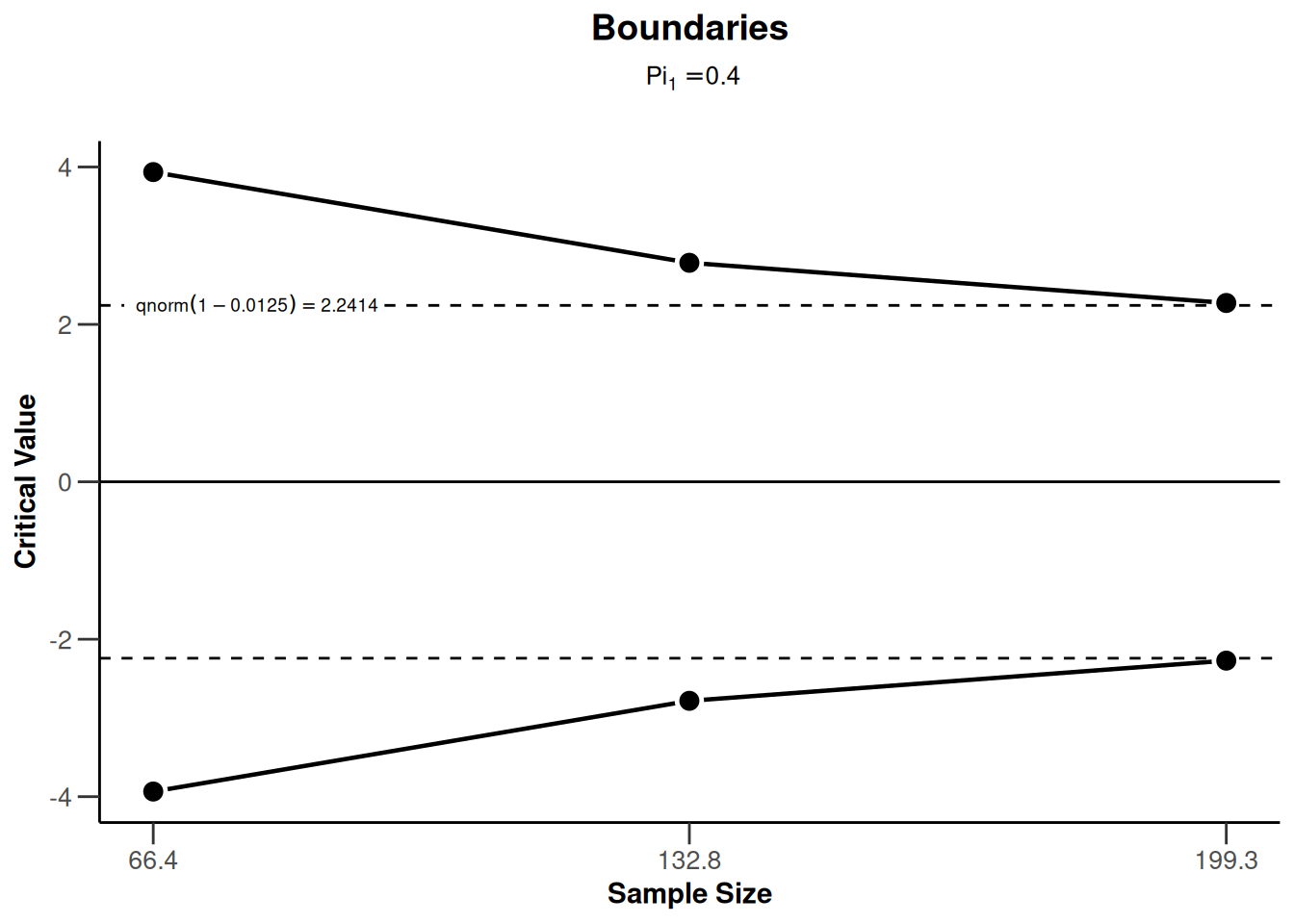``plot(sampleSizeRates2, type = 2)``
``Warning: Only the first 'pi1' (0.4) was used for plotting``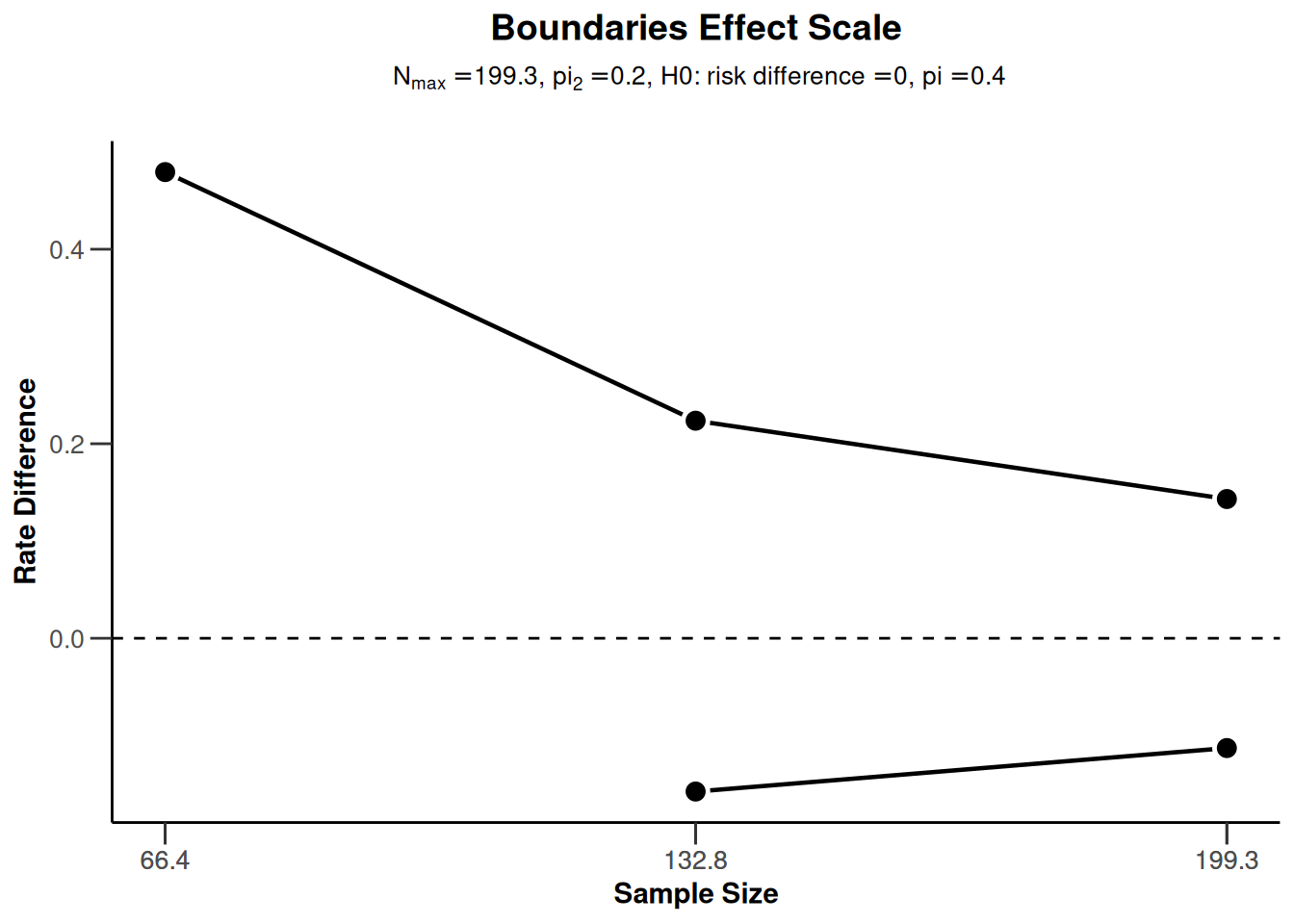## Sample size survival (survival endpoint)

### Sample size survival for a one-sided design with futility bounds

``````design <- getDesignGroupSequential(
kMax = 3, typeOfDesign = "OF", sided = 1,
futilityBounds = c(-1, 0.5)
)

piecewiseSurvivalTime <- list(
"0 - <6" = 0.025,
"6 - <9" = 0.04,
"9 - <15" = 0.015,
"15 - <21" = 0.01,
">= 21" = 0.007
)

sampleSizeSurvival1 <- getSampleSizeSurvival(
design = design,
typeOfComputation = "Schoenfeld",
thetaH0 = 1, allocationRatioPlanned = 1, kappa = 1,
piecewiseSurvivalTime = piecewiseSurvivalTime,
hazardRatio = c(0.5, 0.9)
)

plot(sampleSizeSurvival1, type = 1)``````
``Warning: Only the first 'hazardRatio' (0.5) was used for plotting````plot(sampleSizeSurvival1, type = 2)``
``Warning: Only the first 'hazardRatio' (0.5) was used for plotting``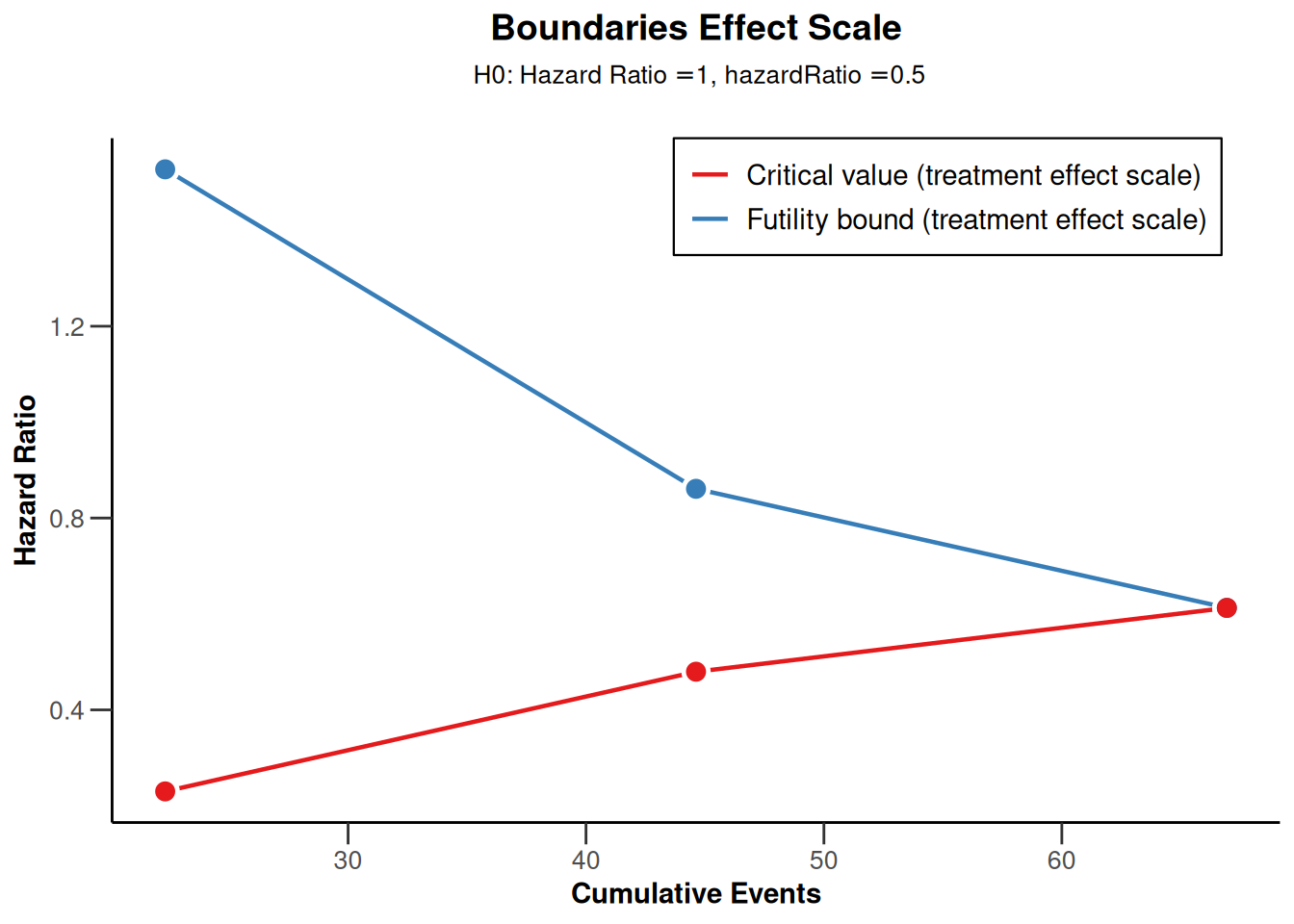``plot(sampleSizeSurvival1, type = 13, legendPosition = 1)``
``Warning: Only the first 'hazardRatio' (0.5) was used for plotting````plot(sampleSizeSurvival1, type = 14)``
``Warning: Only the first 'hazardRatio' (0.5) was used for plotting``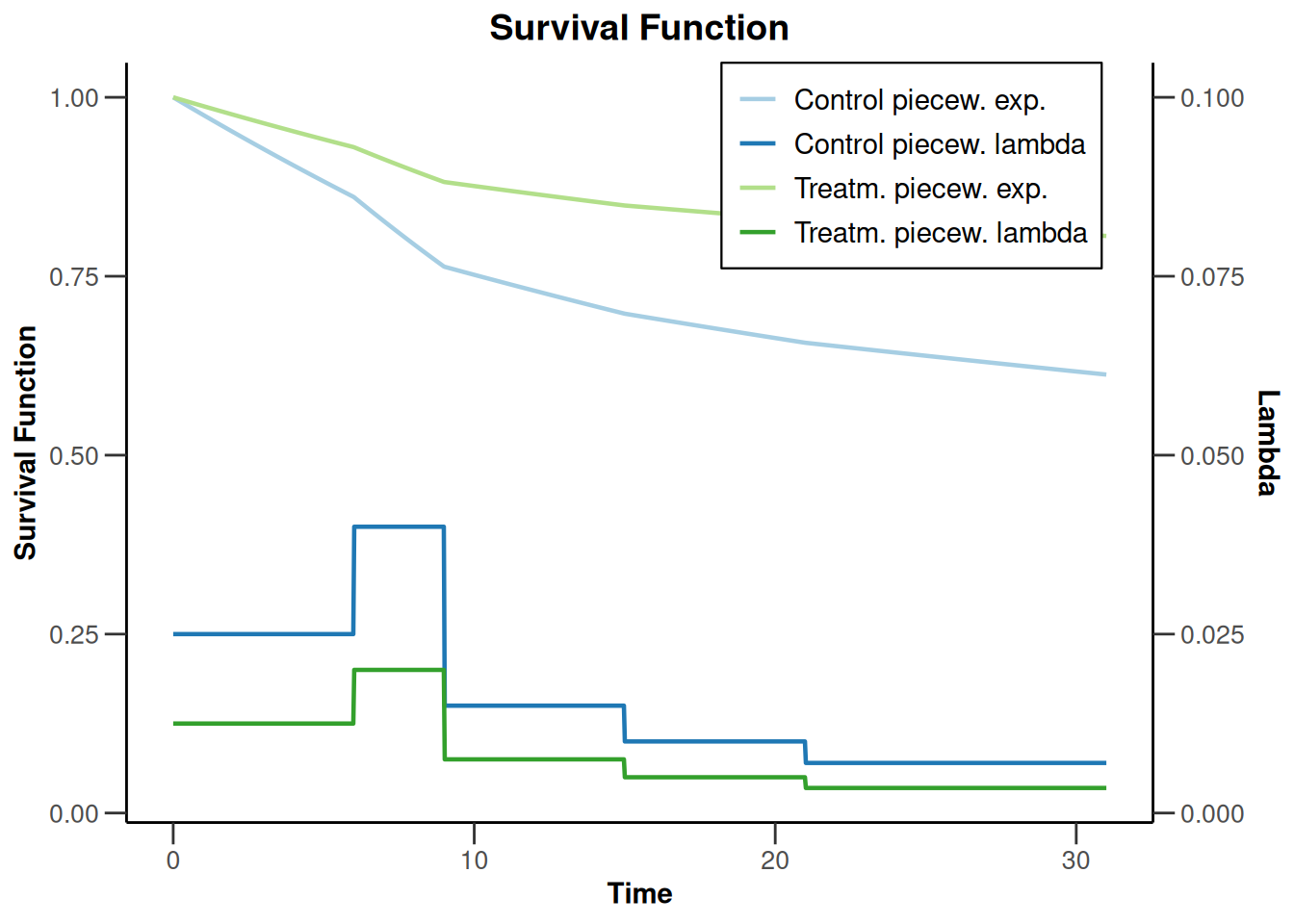### Sample size survival for a two-sided design

``````design <- getDesignGroupSequential(
kMax = 3, typeOfDesign = "OF",
sided = 2, twoSidedPower = TRUE
)

piecewiseSurvivalTime <- list(
"0  - <14" = 0.015,
"14 - <24" = 0.01,
"24 - <44" = 0.005,
">= 44" = 0.0025
)
sampleSizeSurvival2 <- getSampleSizeSurvival(
design = design,
typeOfComputation = "Schoenfeld",
thetaH0 = 1,
allocationRatioPlanned = 1, kappa = 1,
piecewiseSurvivalTime = piecewiseSurvivalTime,
maxNumberOfSubjects = 0, hazardRatio = c(0.1, 0.2, 0.5, 0.6)
)

plot(sampleSizeSurvival2, type = 1)``````
``Warning: Only the first 'hazardRatio' (0.1) was used for plotting``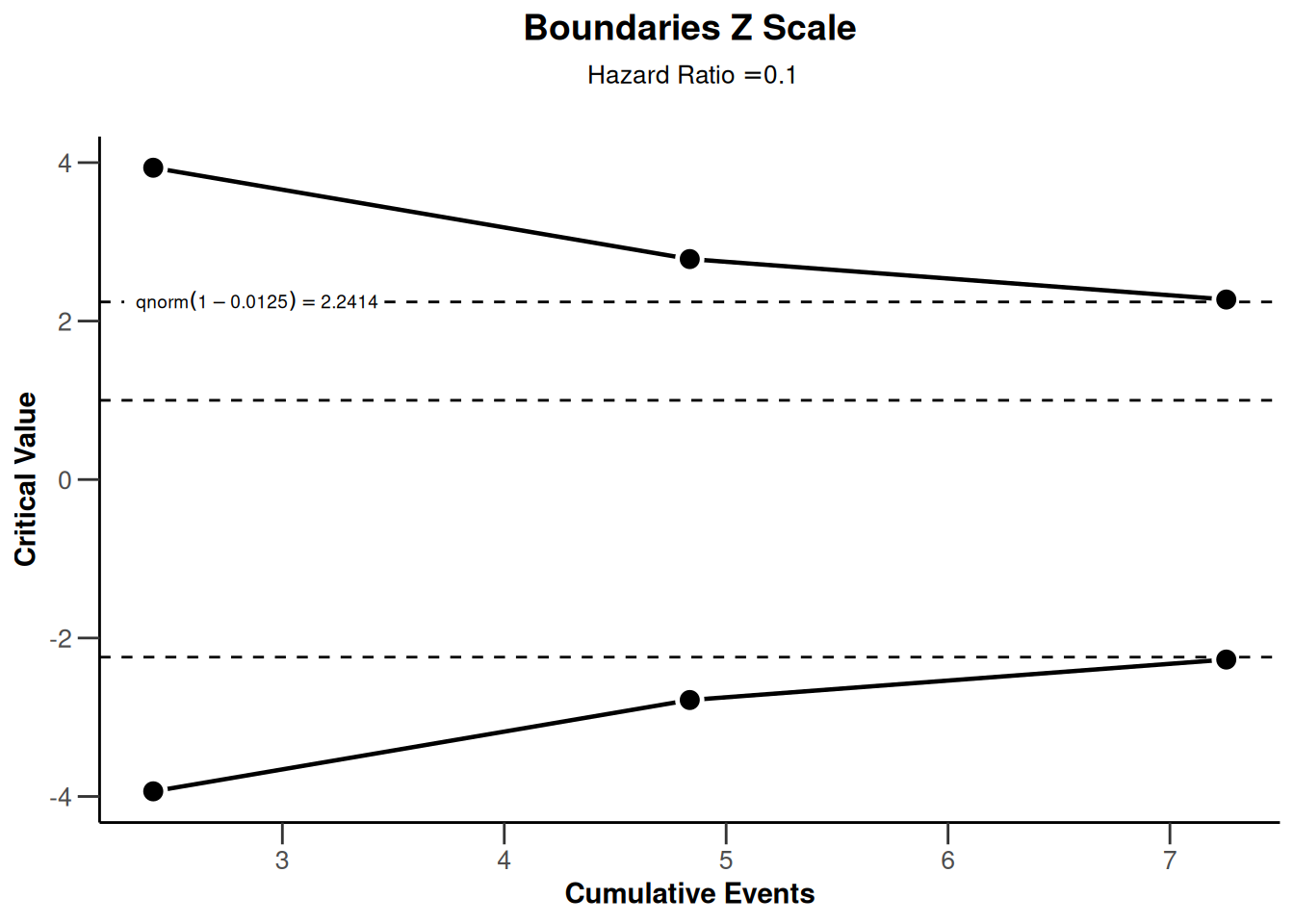``plot(sampleSizeSurvival2, type = 2)``
``Warning: Only the first 'hazardRatio' (0.1) was used for plotting``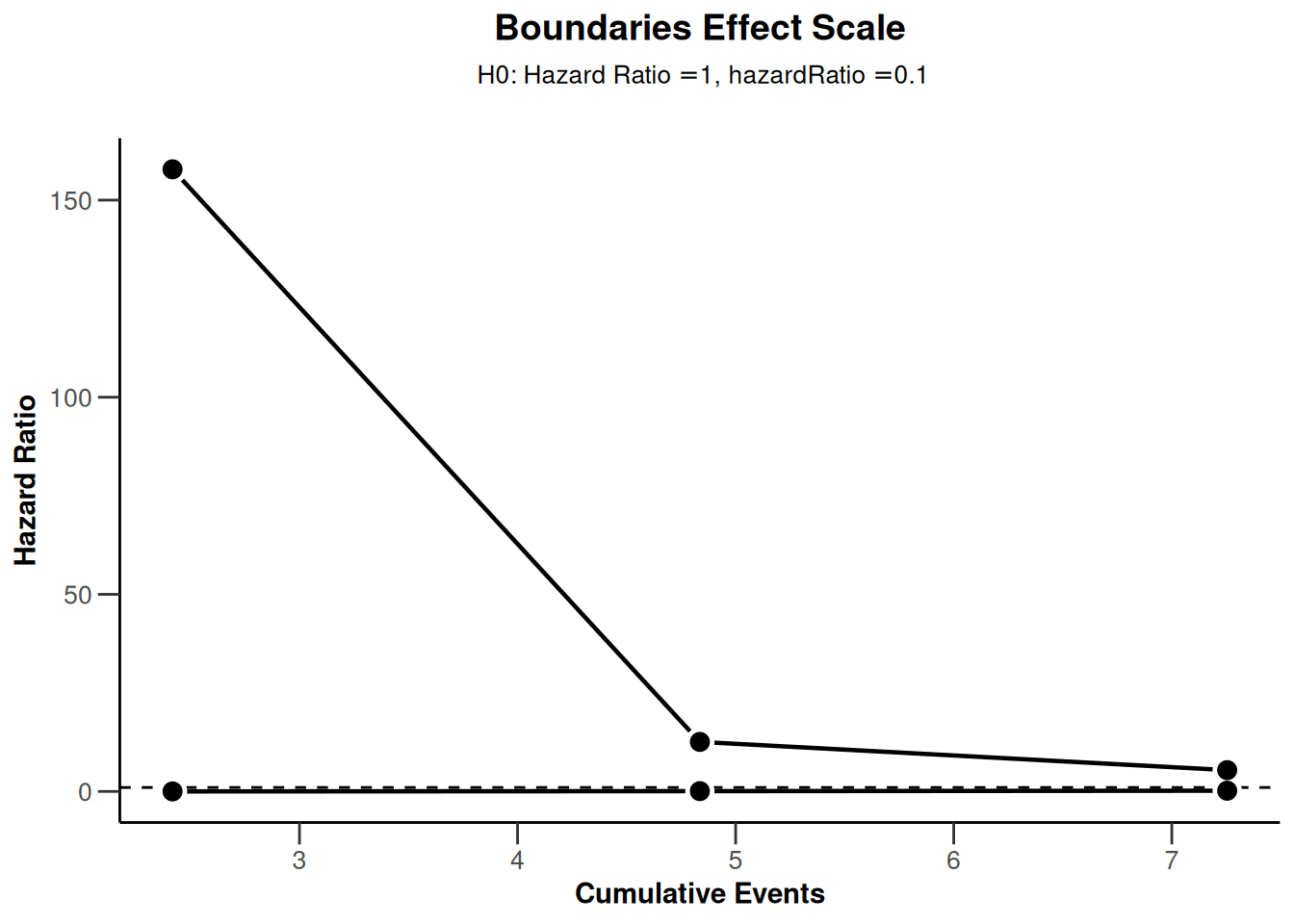``plot(sampleSizeSurvival2, type = 13, legendPosition = 1)``
``Warning: Only the first 'hazardRatio' (0.1) was used for plotting````plot(sampleSizeSurvival2, type = 14)``
``Warning: Only the first 'hazardRatio' (0.1) was used for plotting``# Power plots

## Power means (continuous endpoint)

### Power means for a one-sided design with futility bounds

``````design <- getDesignGroupSequential(
typeOfDesign = "OF", sided = 1,
futilityBounds = c(0, 0.5)
)

powerMeans1 <- getPowerMeans(design,
groups = 1, meanRatio = FALSE,
thetaH0 = 0, alternative = c(-1, 4),
stDev = 2, normalApproximation = FALSE,
maxNumberOfSubjects = 40
)

plot(powerMeans1, type = 1)``````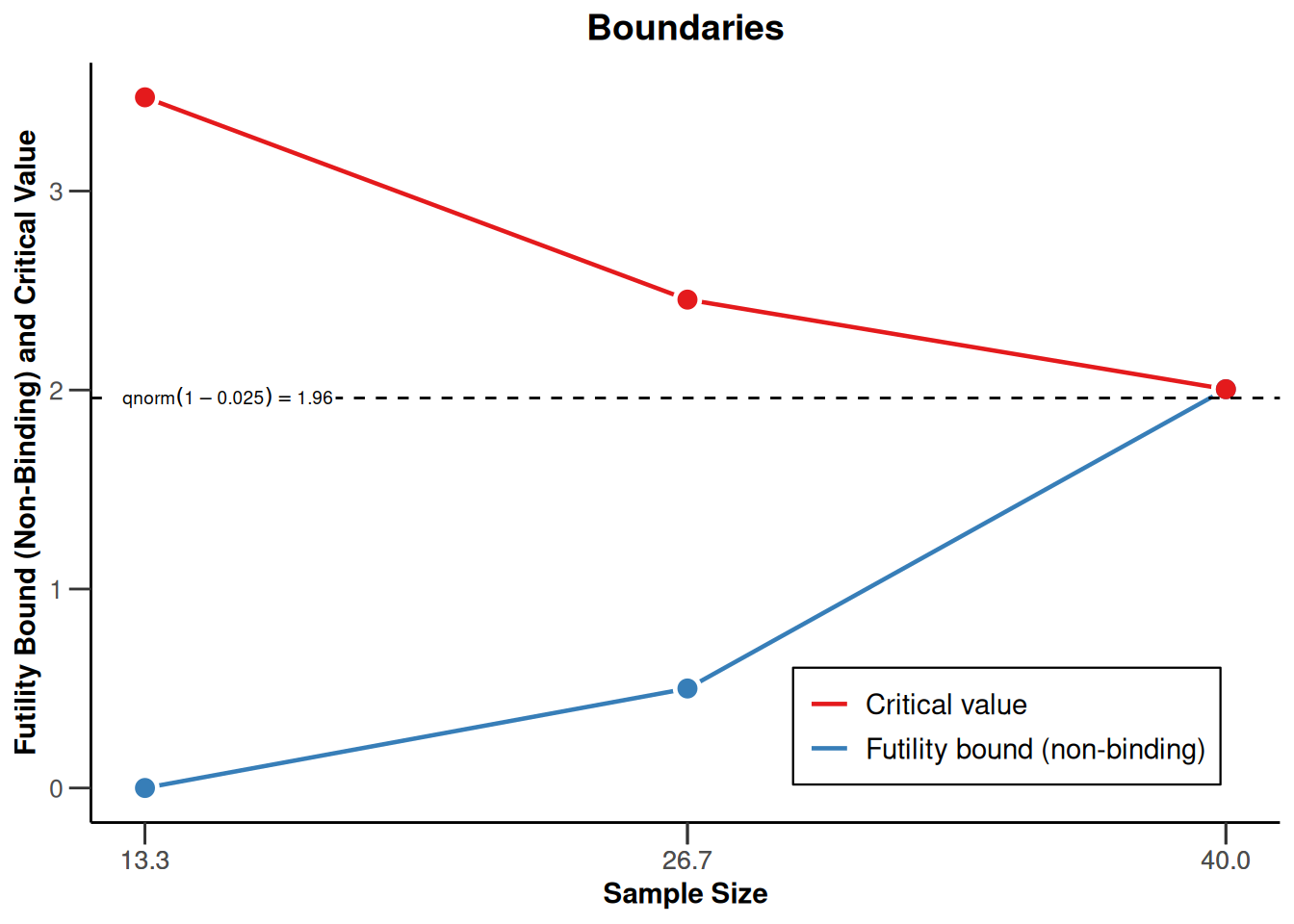``plot(powerMeans1, type = 2)````plot(powerMeans1, type = 5)``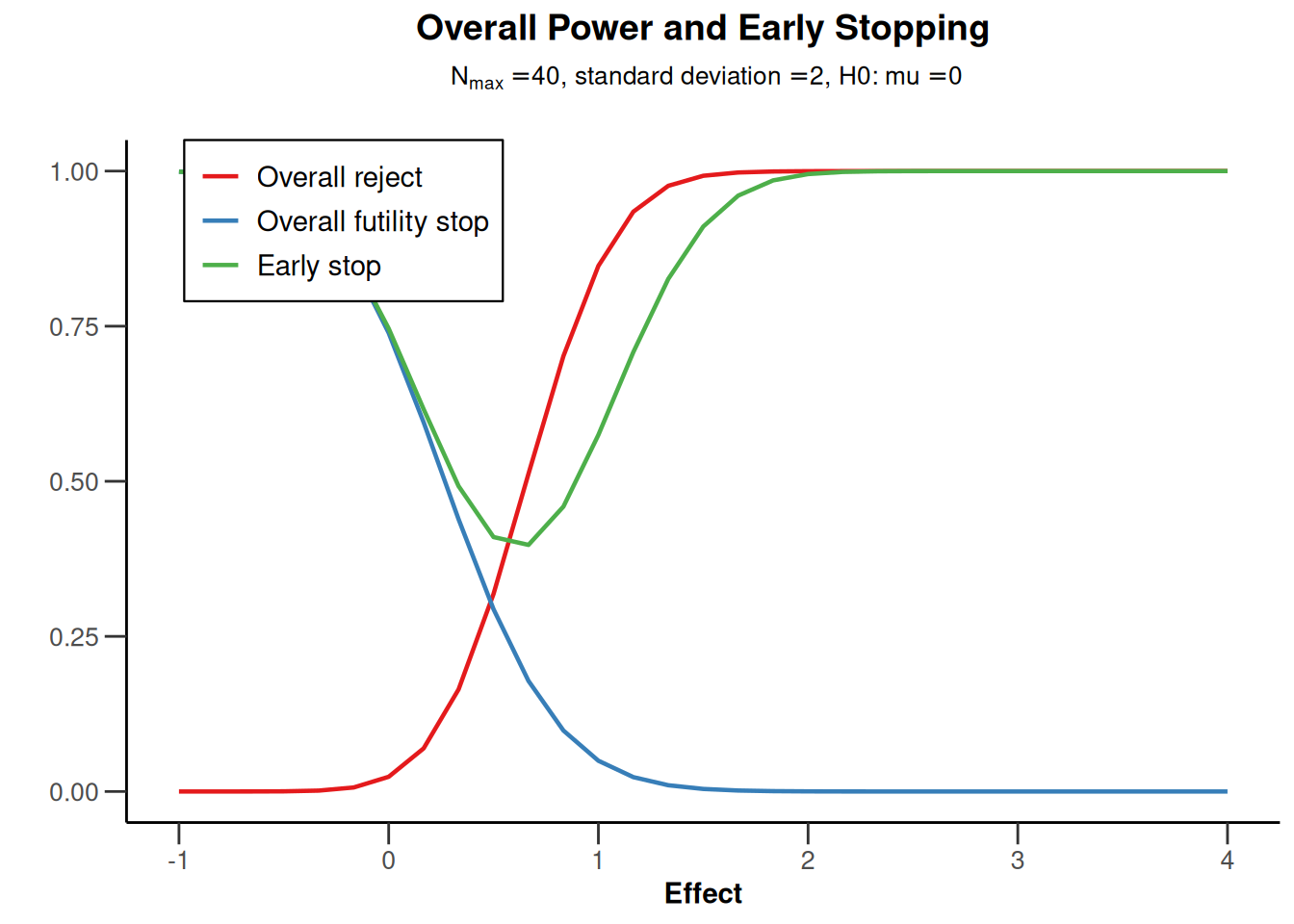``plot(powerMeans1, type = 6)``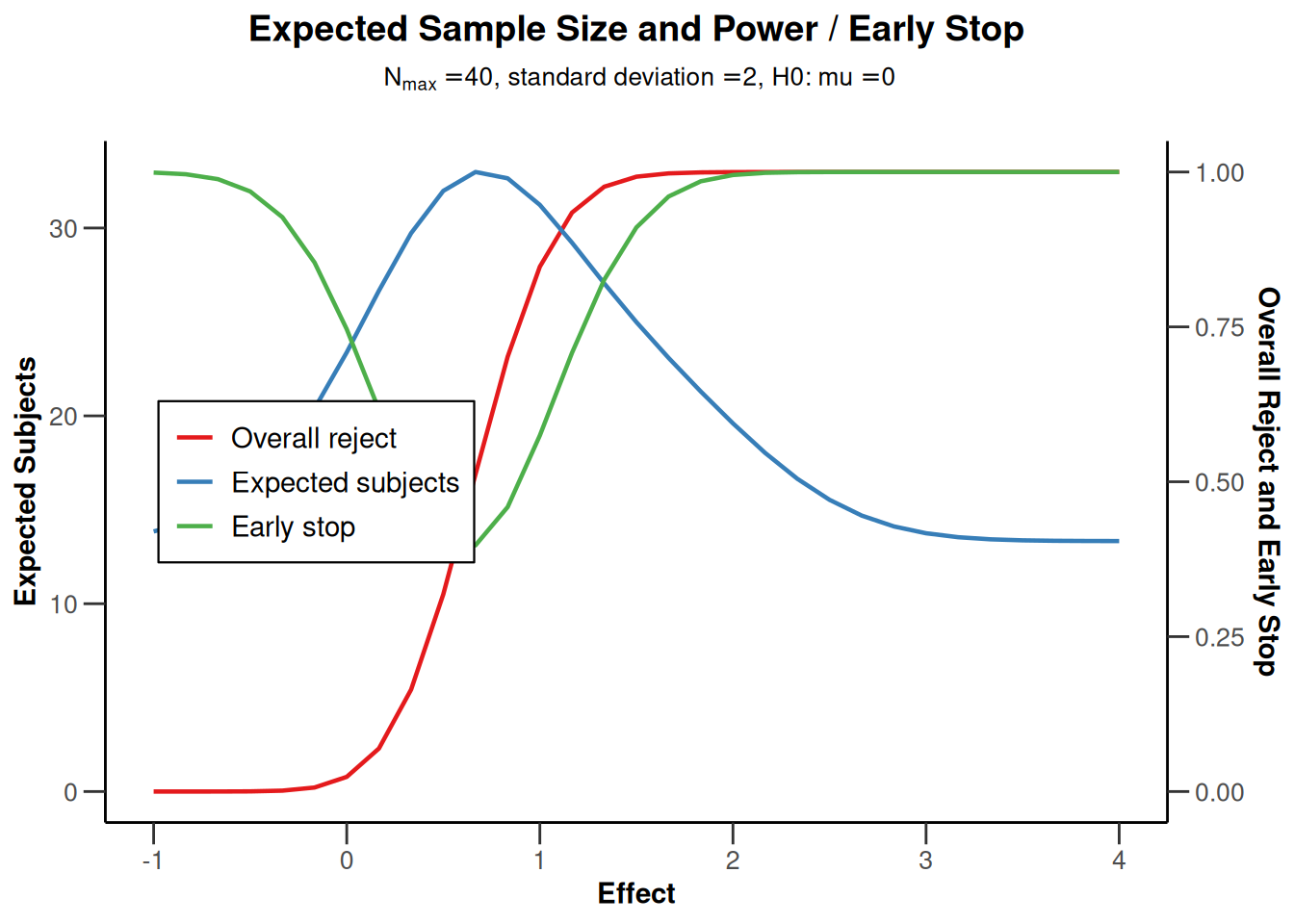### Power means for a two-sided design

``````powerMeans2 <- getPowerMeans(
getDesignGroupSequential(
typeOfDesign = "OF", sided = 2, twoSidedPower = TRUE
),
maxNumberOfSubjects = 30
)

plot(powerMeans2, type = 1)``````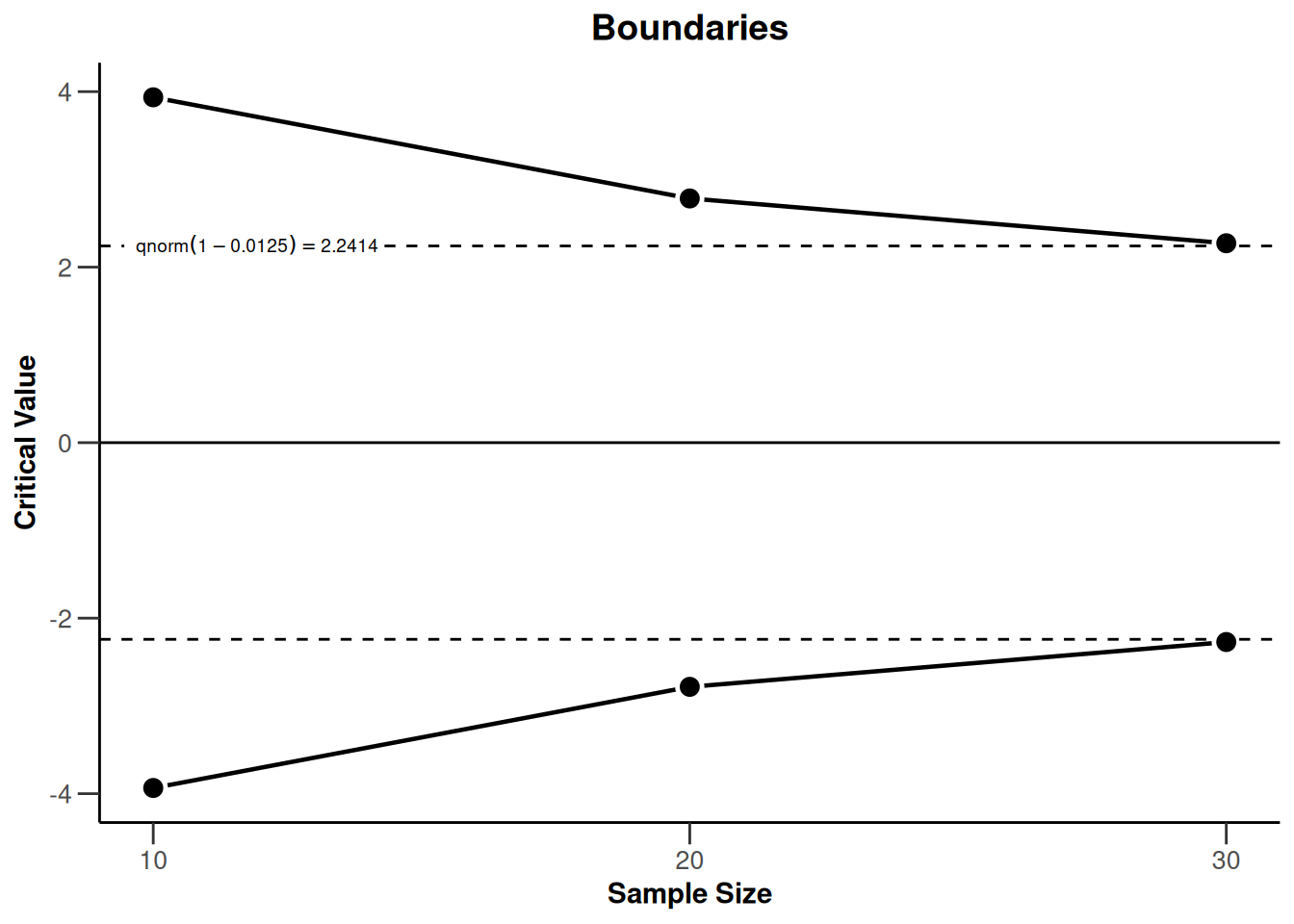``plot(powerMeans2, type = 2)``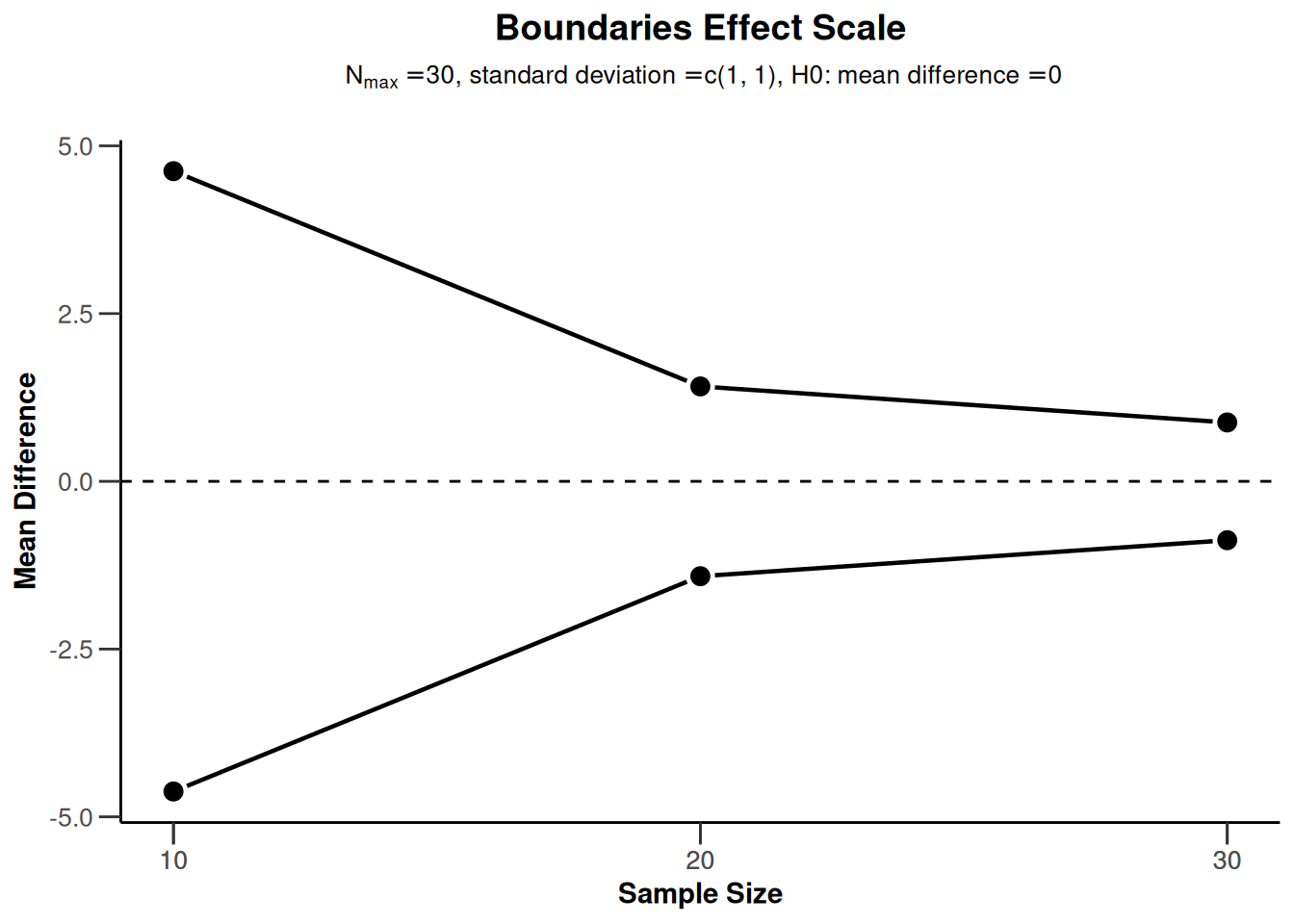``plot(powerMeans2, type = 5)````plot(powerMeans2, type = 6)``## Power rates (binary endpoint)

### Power rates for a one-sided design with futility bounds

``````design <- getDesignGroupSequential(
kMax = 3,
typeOfDesign = "OF",
sided = 1,
futilityBounds = c(-1, 0.5)
)

powerRates1 <- getPowerRates(design,
groups = 2, riskRatio = TRUE,
thetaH0 = 0.2, allocationRatioPlanned = 1,
pi1 = c(0.1, 0.4), pi2 = 0.2,
maxNumberOfSubjects = 80
)

plot(powerRates1, type = 1)````````plot(powerRates1, type = 2)``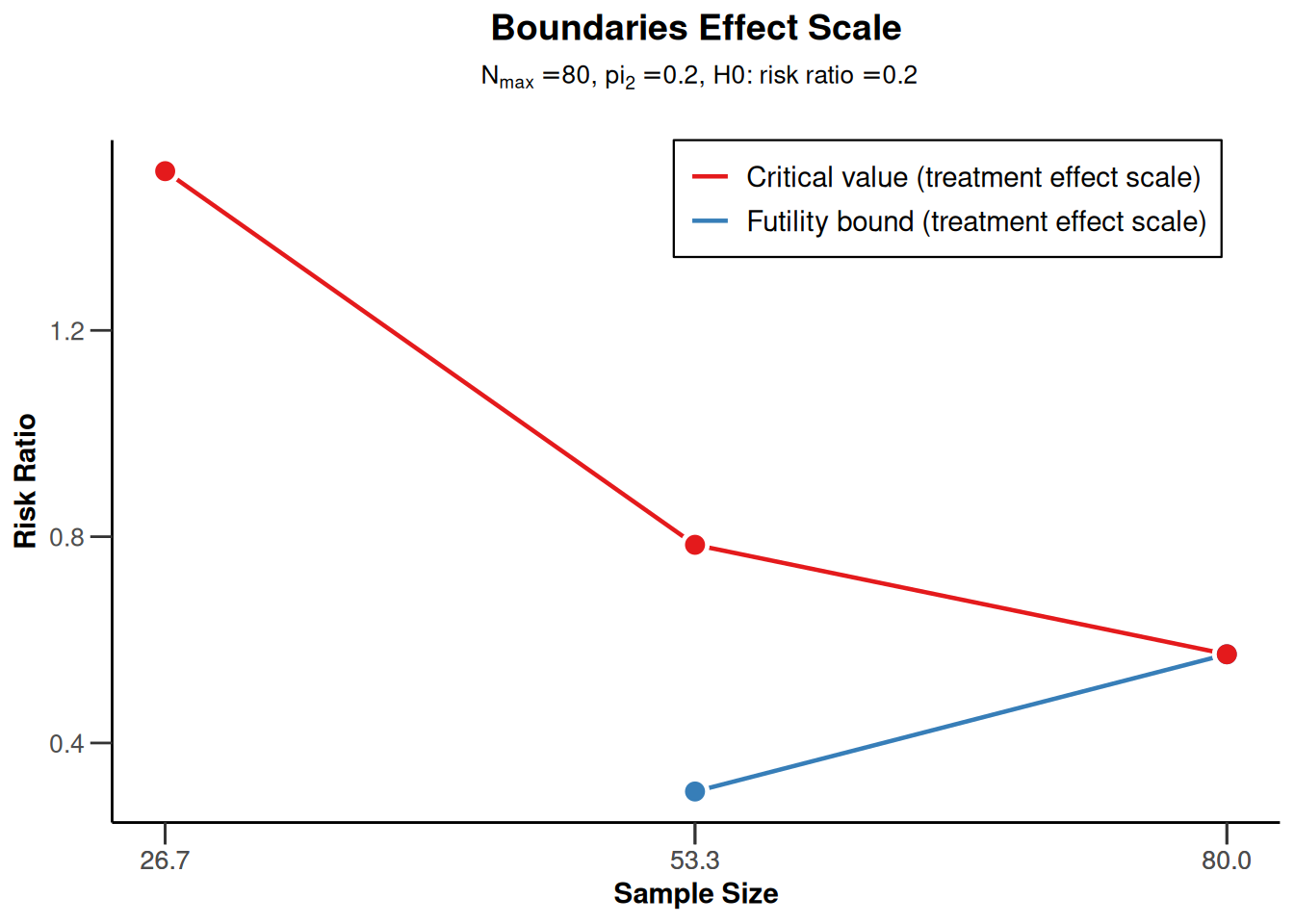``plot(powerRates1, type = 5)``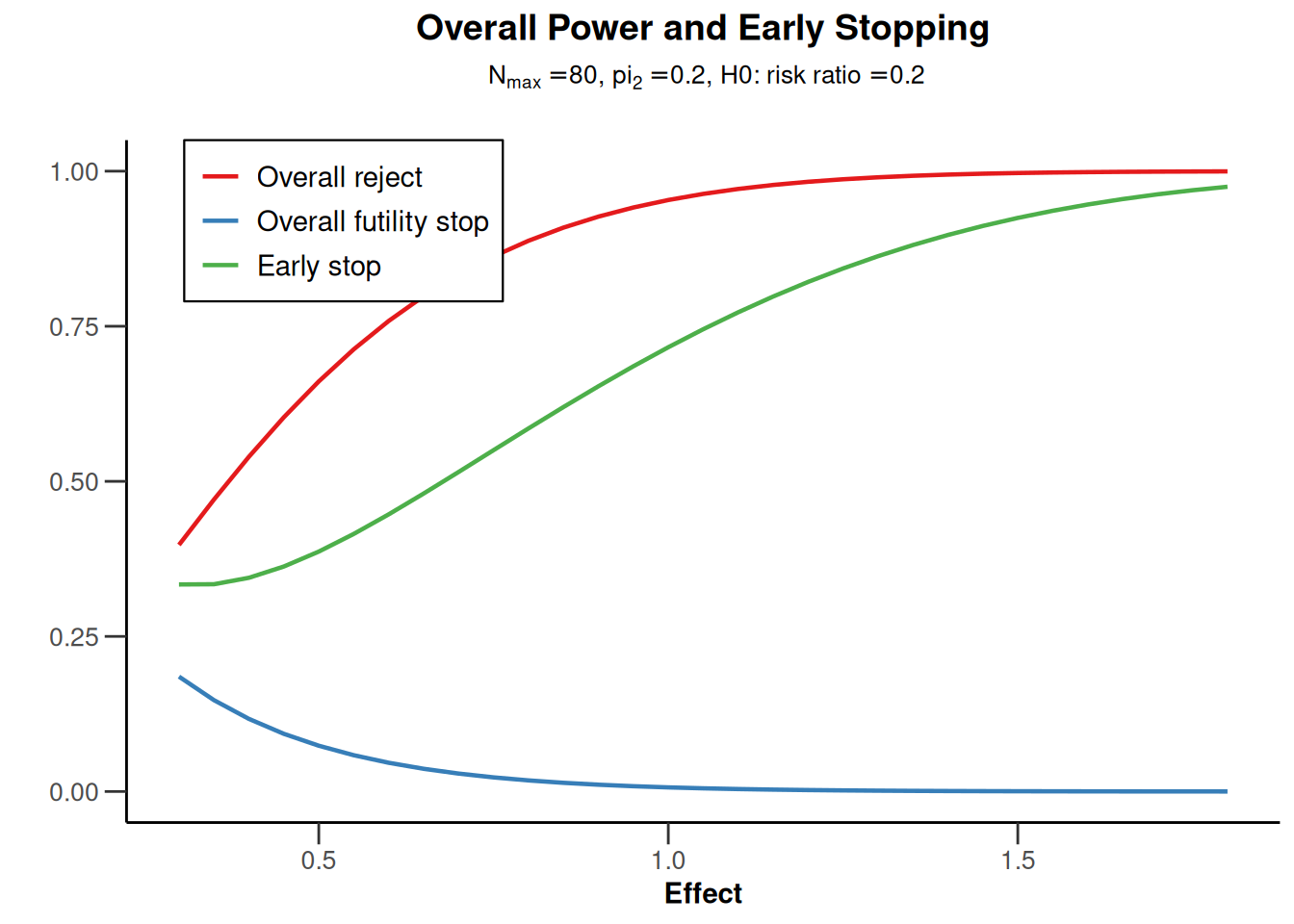``plot(powerRates1, type = 6)``### Power rates for a two-sided design

``````powerRates2 <- getPowerRates(
getDesignGroupSequential(
typeOfDesign = "OF", sided = 2, twoSidedPower = TRUE
),
maxNumberOfSubjects = 120
)

plot(powerRates2, type = 1)``````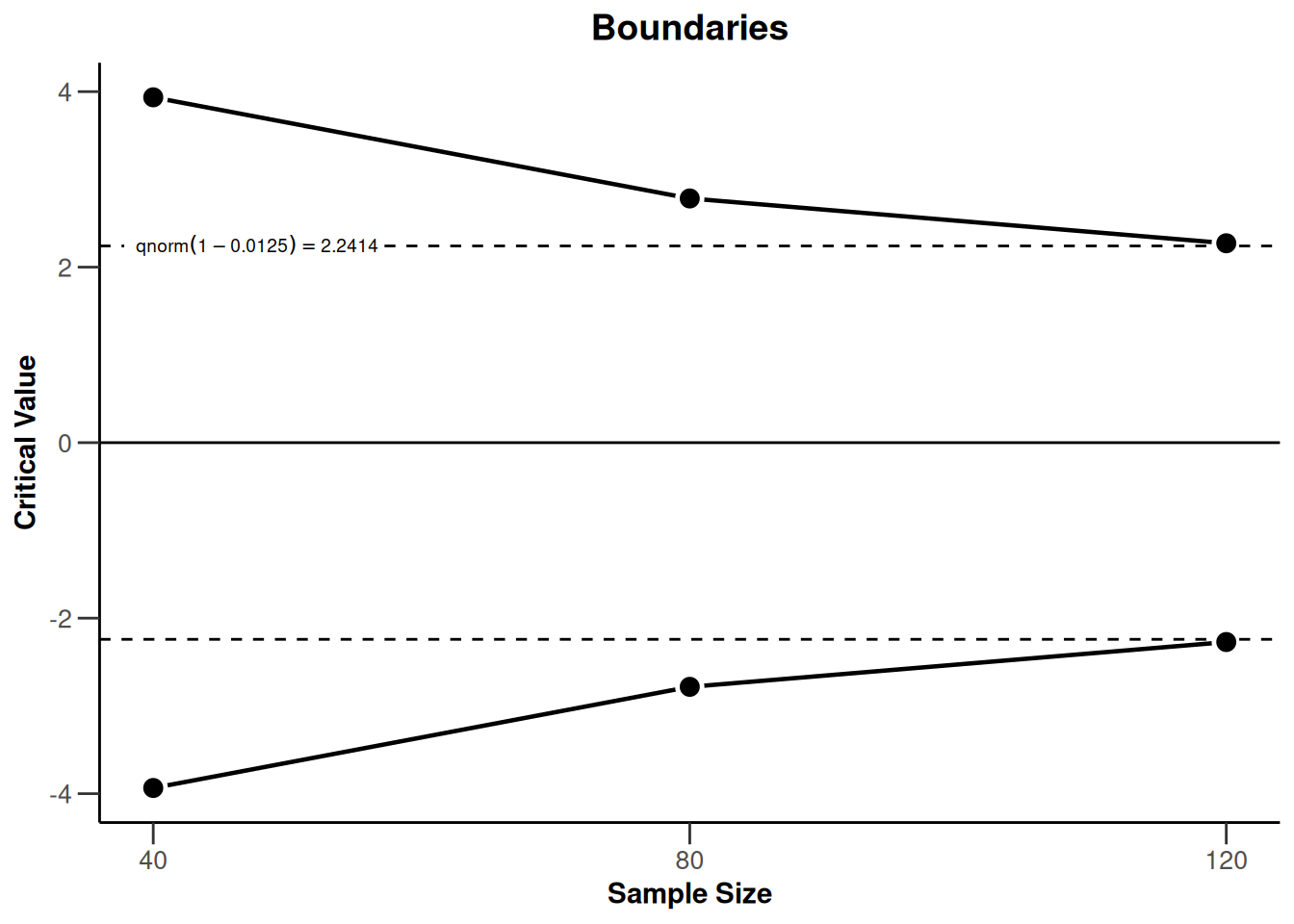``plot(powerRates2, type = 2)``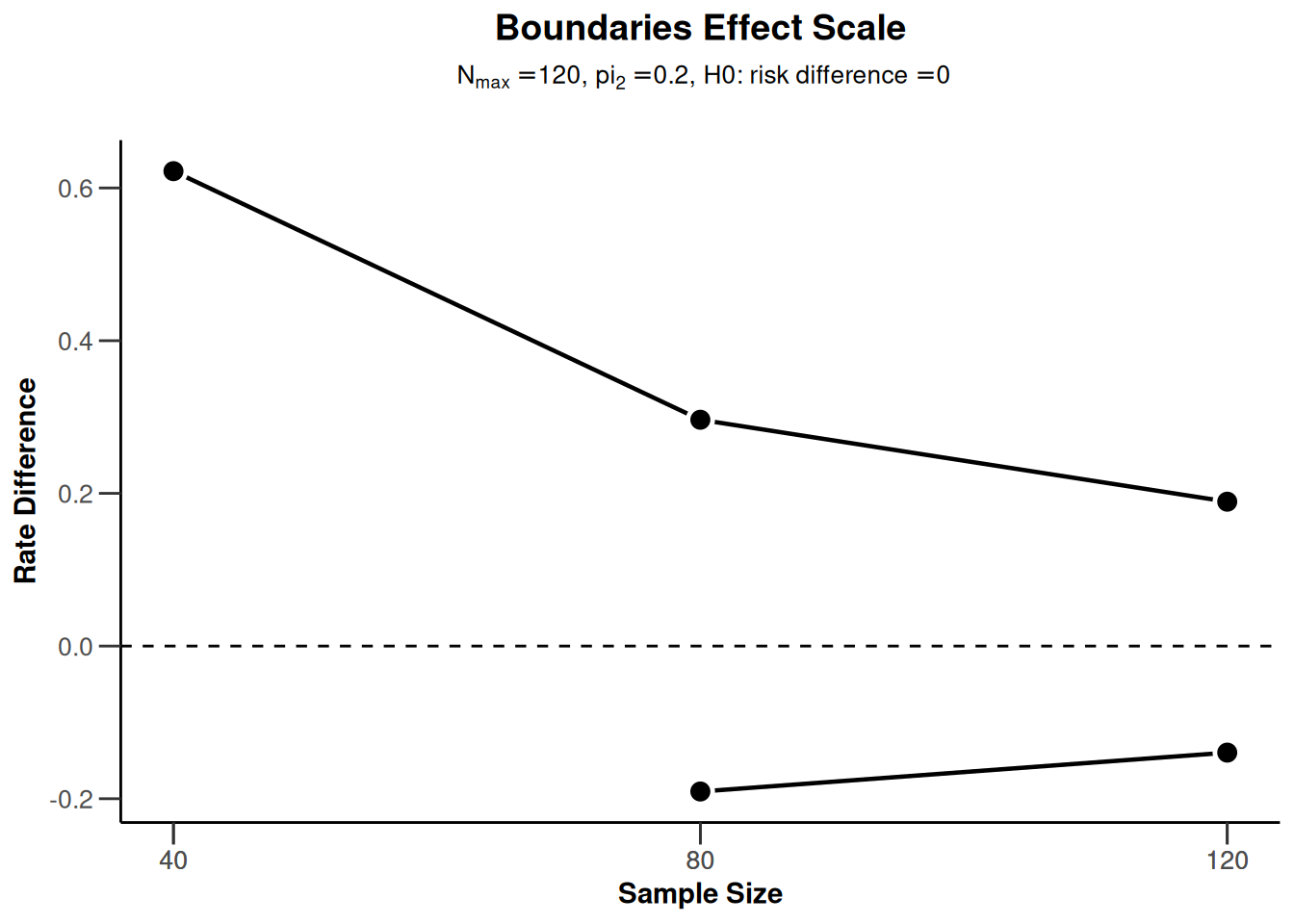``plot(powerRates2, type = 5)``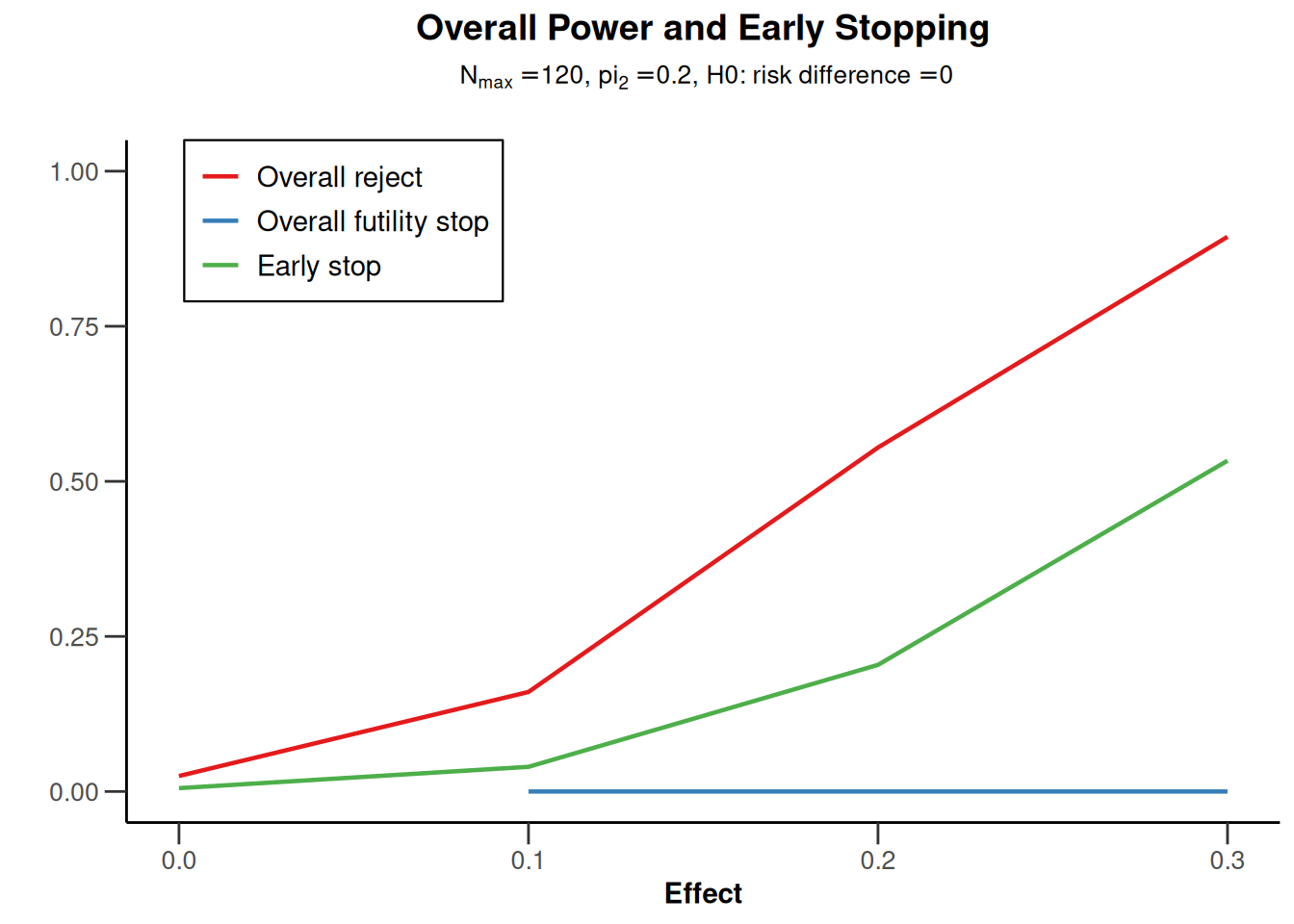``plot(powerRates2, type = 6)``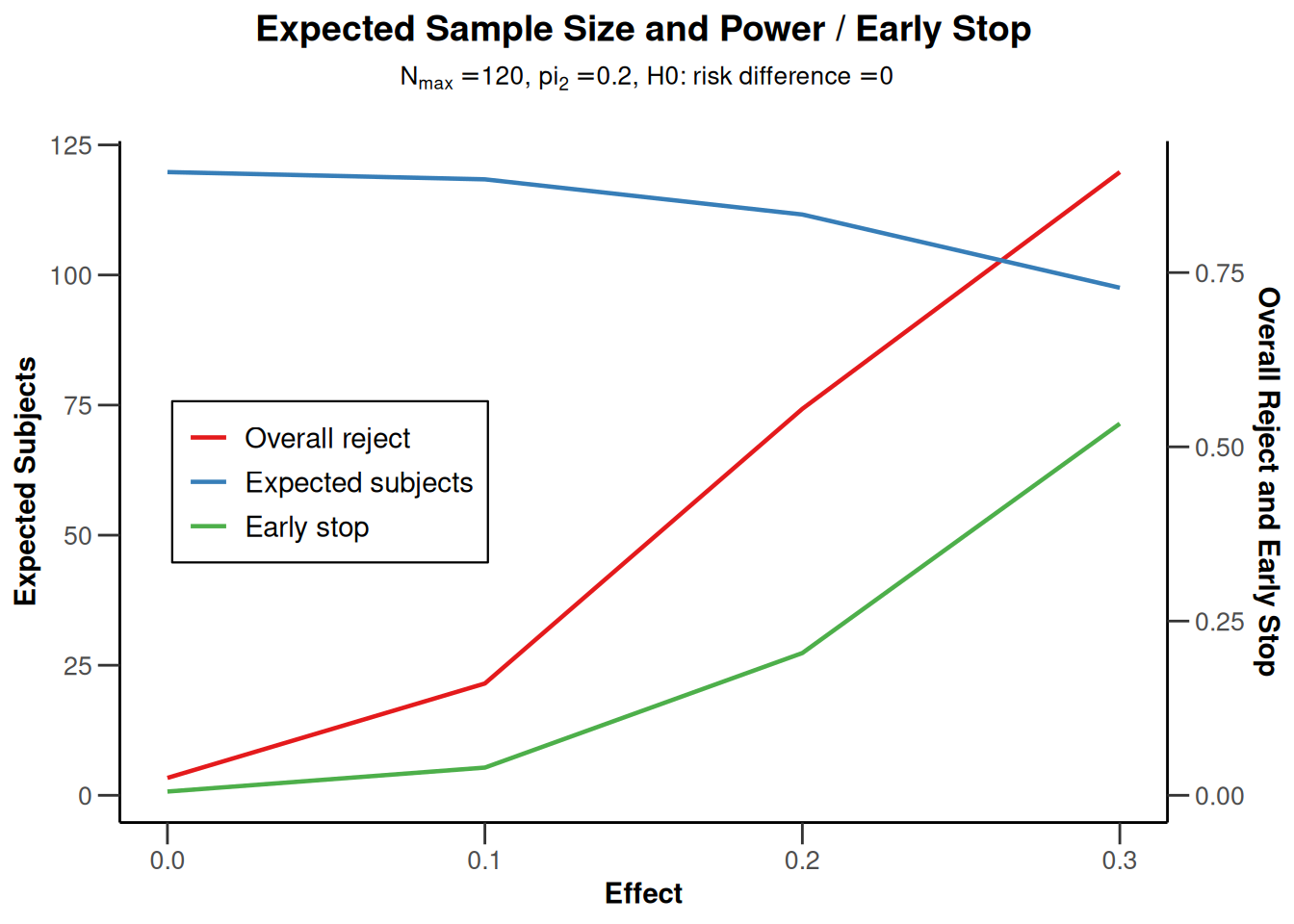## Power survival (survival endpoint)

### Power survival for a one-sided design with futility bounds

``````design <- getDesignGroupSequential(
kMax = 3,
typeOfDesign = "OF", sided = 1,
futilityBounds = c(0, 0)
)

powerSurvival <- getPowerSurvival(
design = design,
typeOfComputation = "Schoenfeld", thetaH0 = 1,
allocationRatioPlanned = 1, kappa = 1,
maxNumberOfSubjects = 2480,
maxNumberOfEvents = 70
)

plot(powerSurvival, type = 1)``````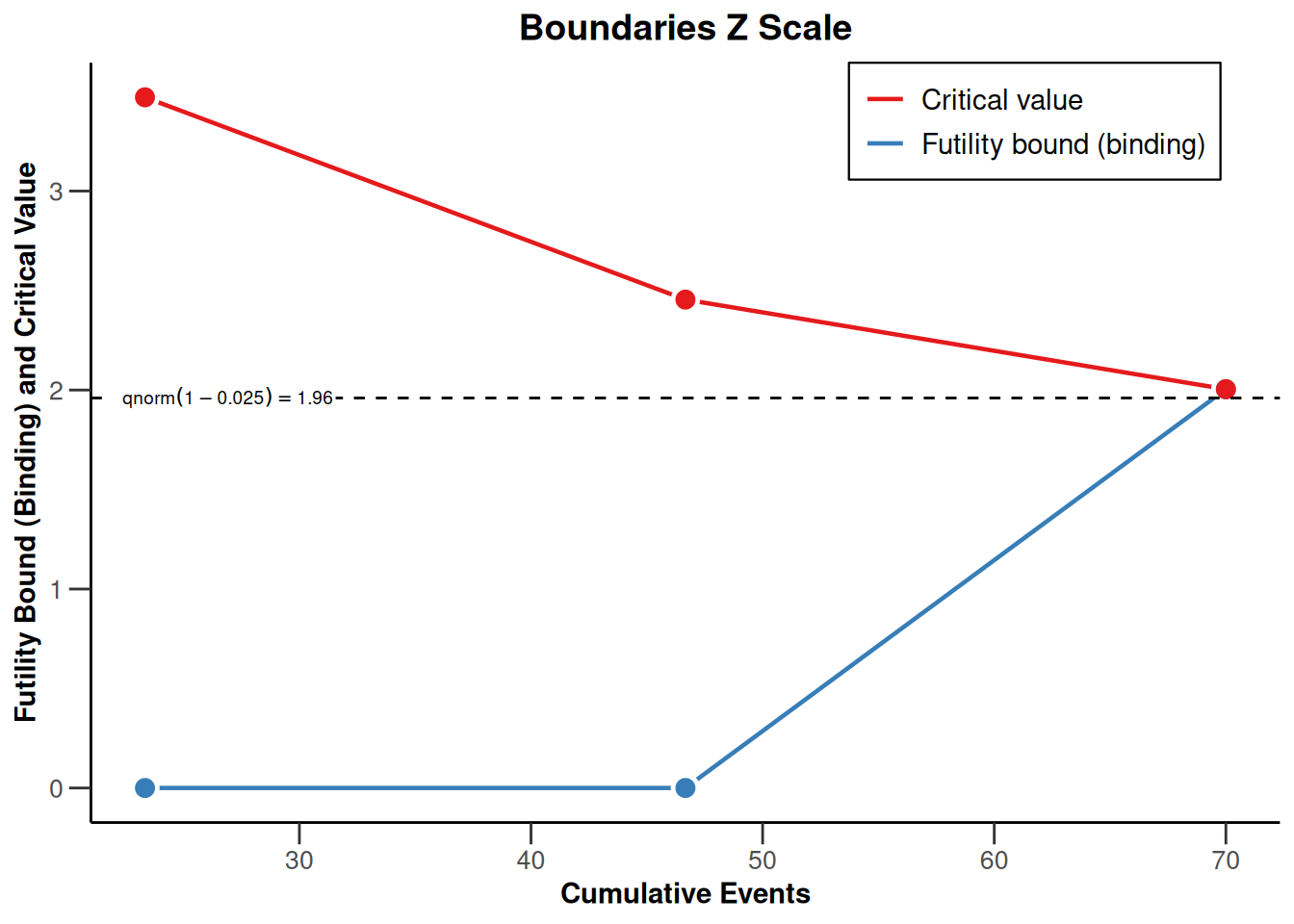``plot(powerSurvival, type = 2)````plot(powerSurvival, type = 5)````plot(powerSurvival, type = 6)``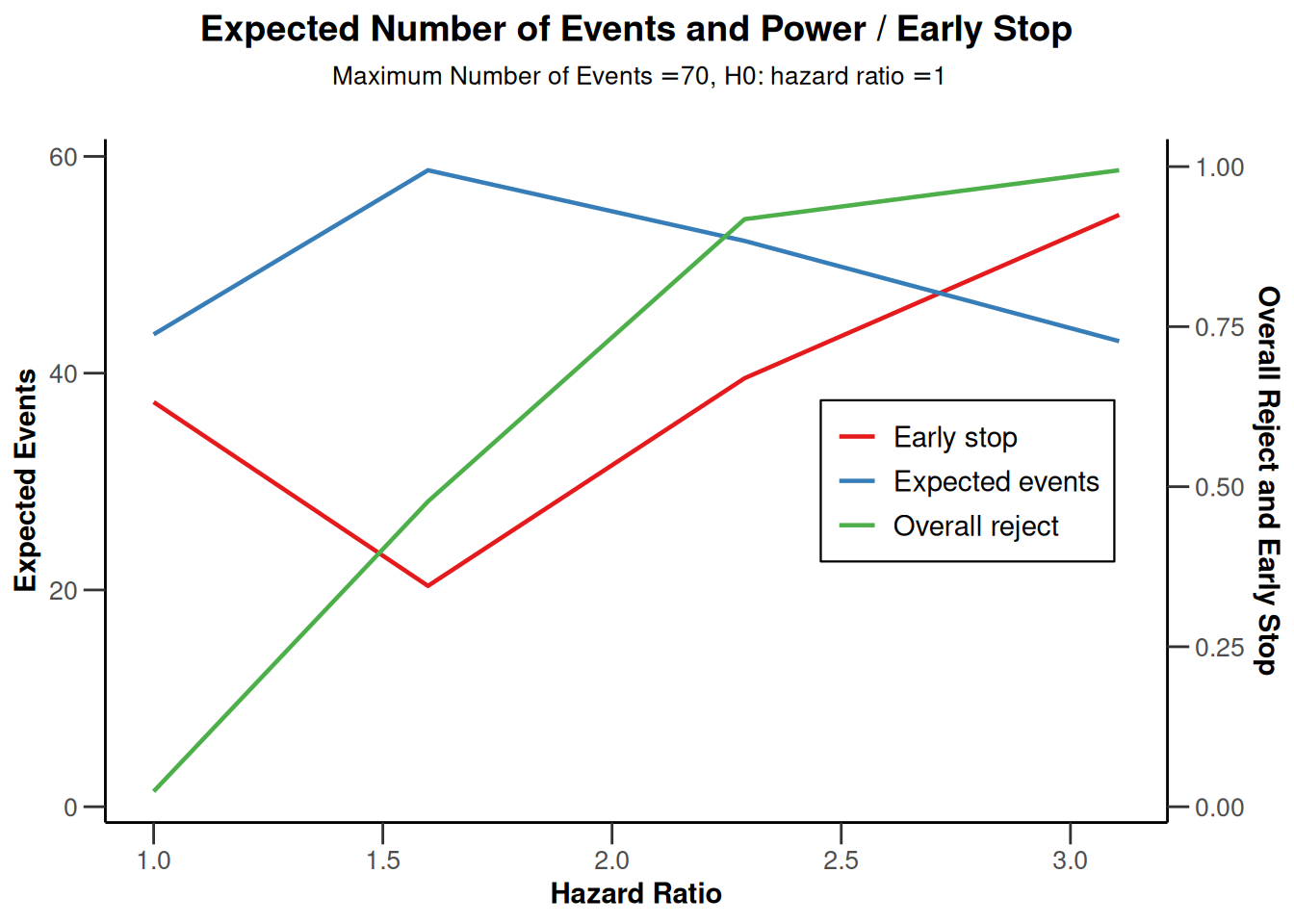``plot(powerSurvival, type = 7)``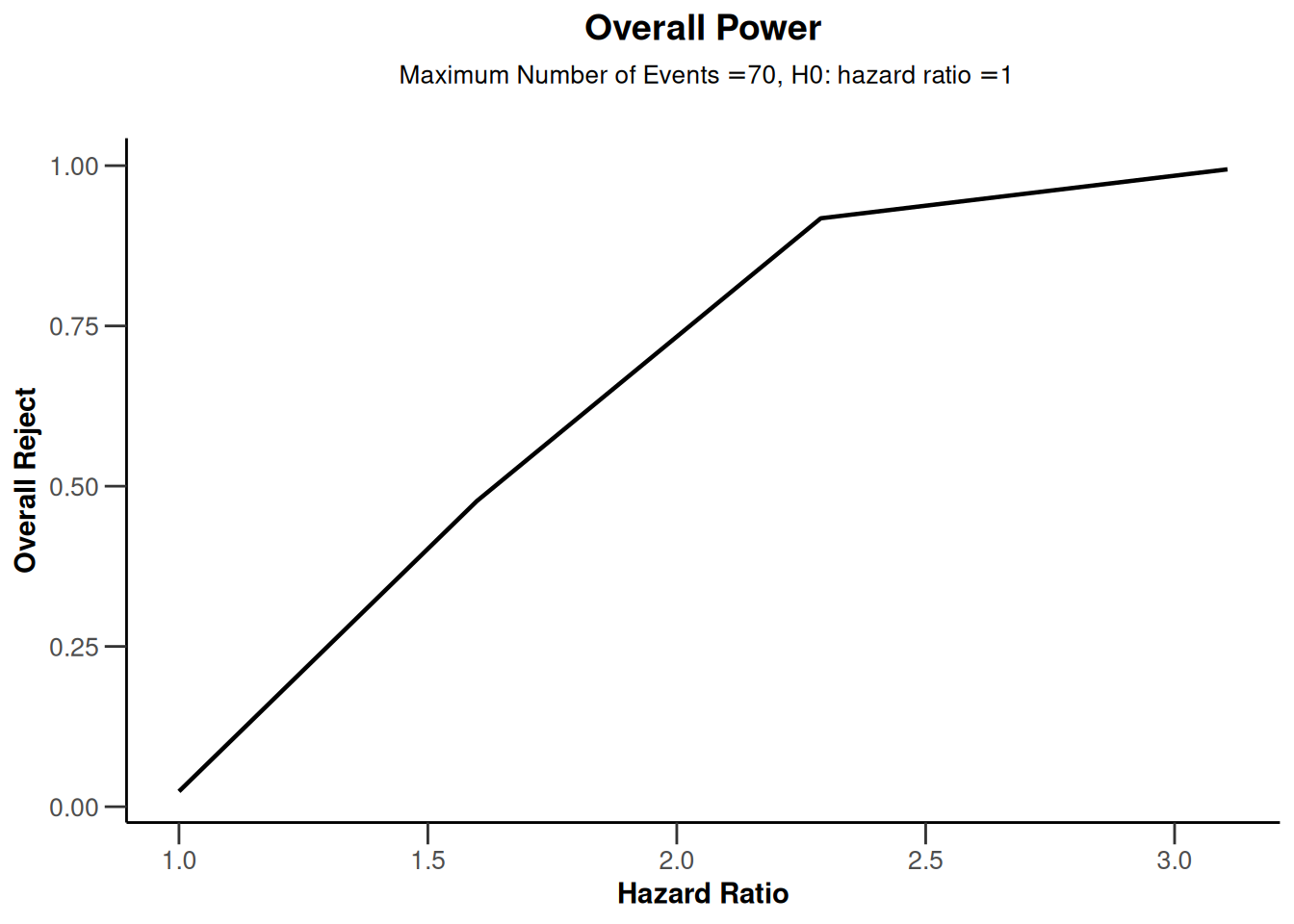``plot(powerSurvival, type = 12)``### Power for a piecewise exponential survival distribution

``````design <- getDesignGroupSequential(
kMax = 3, typeOfDesign = "OF",
sided = 2, twoSidedPower = TRUE
)
piecewiseSurvivalTime <- list(
"<5" = 0.04,
"5 - <10" = 0.02,
">= 10" = 0.008
)

powerSurvival1 <- getPowerSurvival(
design = design,
typeOfComputation = "Schoenfeld", thetaH0 = 1,
allocationRatioPlanned = 1, kappa = 1,
piecewiseSurvivalTime = piecewiseSurvivalTime,
maxNumberOfSubjects = 2480, maxNumberOfEvents = 70,
hazardRatio = c(0.5, 2)
)

plot(powerSurvival1, type = 1)````````plot(powerSurvival1, type = 2)``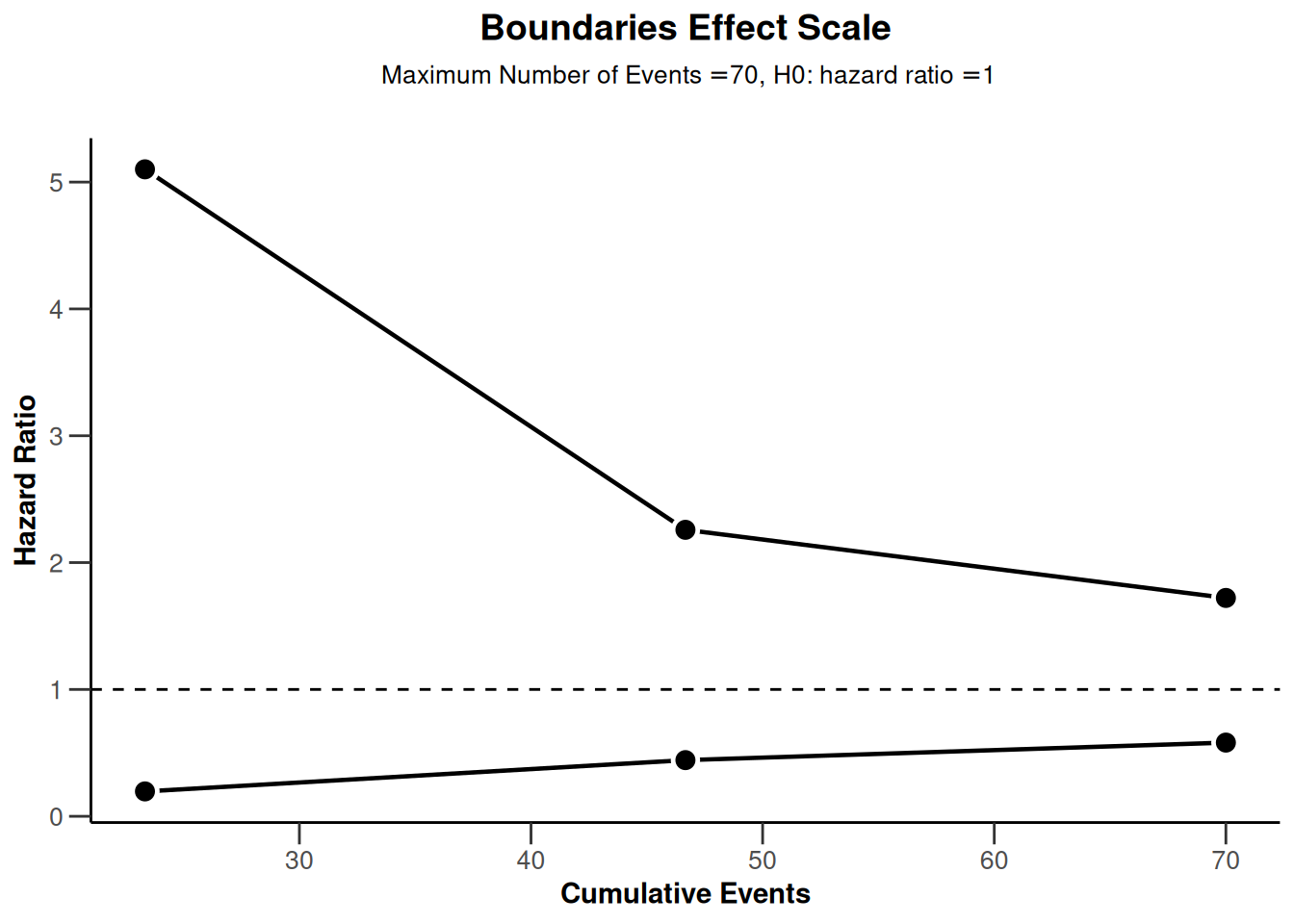``plot(powerSurvival1, type = 12)````plot(powerSurvival1, type = 13, legendPosition = 1)``
``Warning: Only the first 'hazardRatio' (0.5) was used for plotting````plot(powerSurvival1, type = 14, legendPosition = 5)``
``Warning: Only the first 'hazardRatio' (0.5) was used for plotting``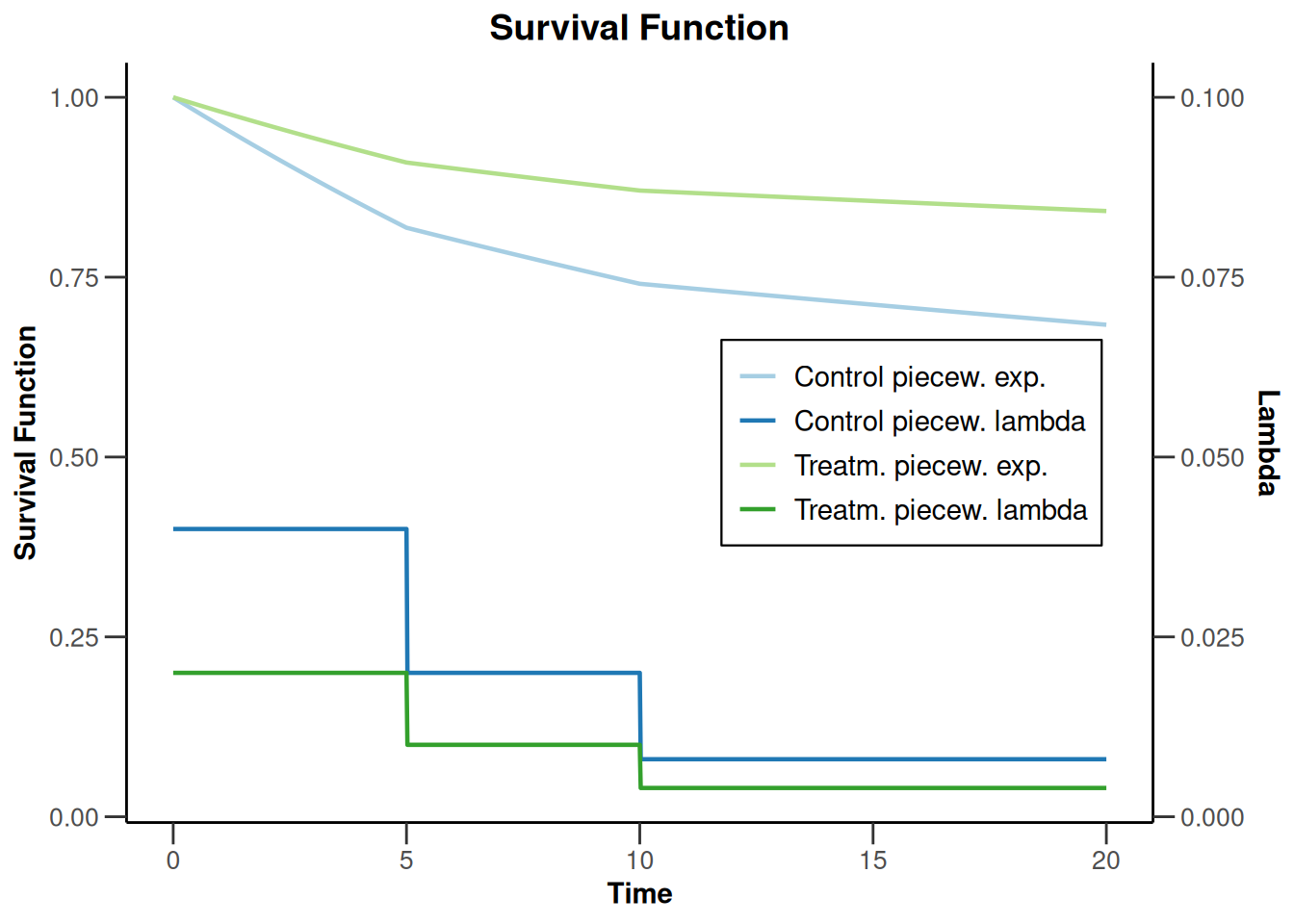### Power for a piecewise exponential survival distribution and a design with futility bounds

``````design <- getDesignGroupSequential(
typeOfDesign = "OF", sided = 1,
futilityBounds = c(0, 0.1)
)

piecewiseSurvivalTime <- list(
"0 - <6" = 0.025,
"6 - <9" = 0.04,
"9 - <15" = 0.015,
"15 - <21" = 0.01,
">= 21" = 0.007
)

powerSurvival2 <- getPowerSurvival(
design = design,
typeOfComputation = "Schoenfeld", thetaH0 = 1,
allocationRatioPlanned = 1, kappa = 1,
piecewiseSurvivalTime = piecewiseSurvivalTime,
maxNumberOfSubjects = 2480, maxNumberOfEvents = 70,
hazardRatio = 0.8
)
plot(powerSurvival2, type = 1)``````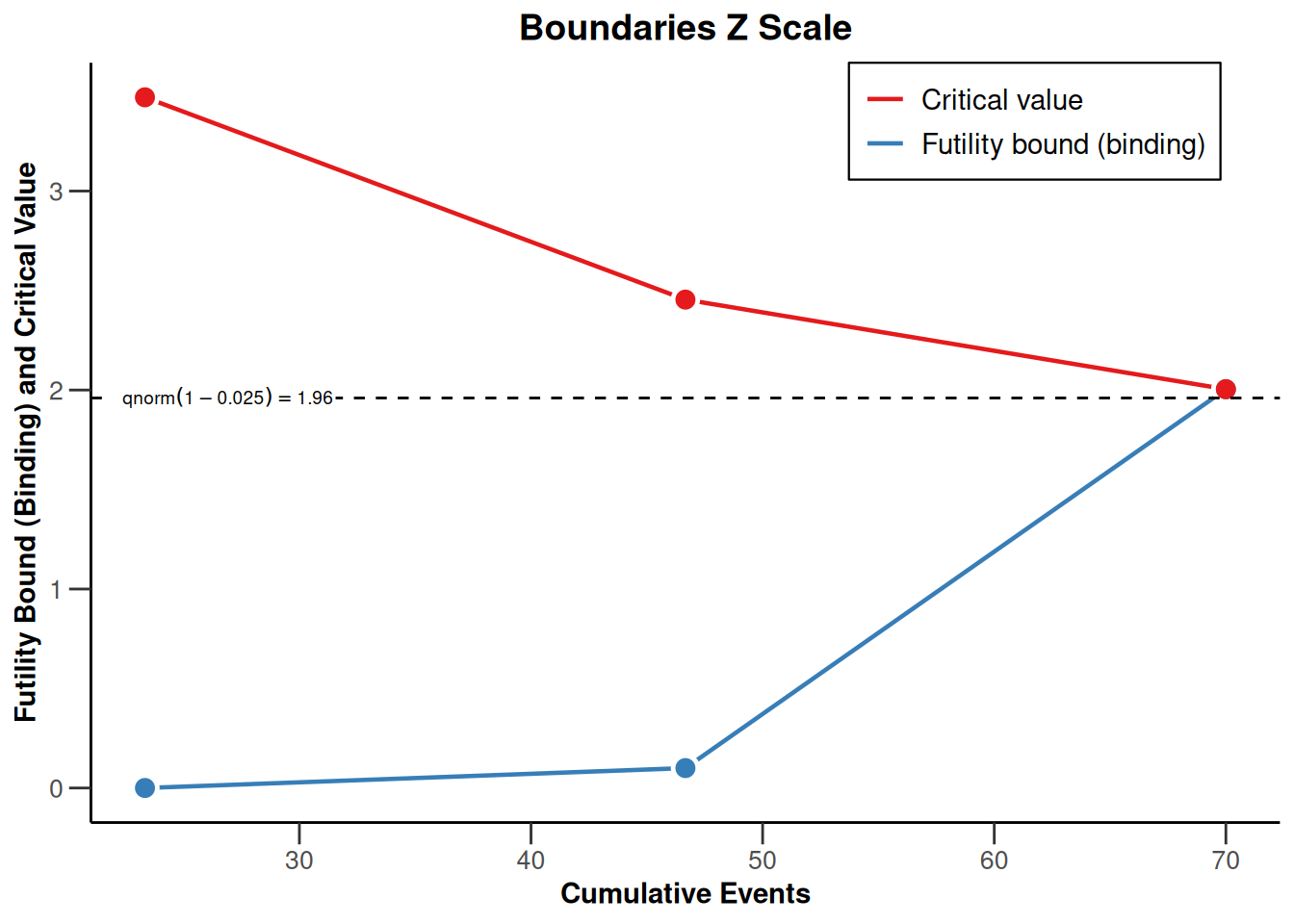``plot(powerSurvival2, type = 2)``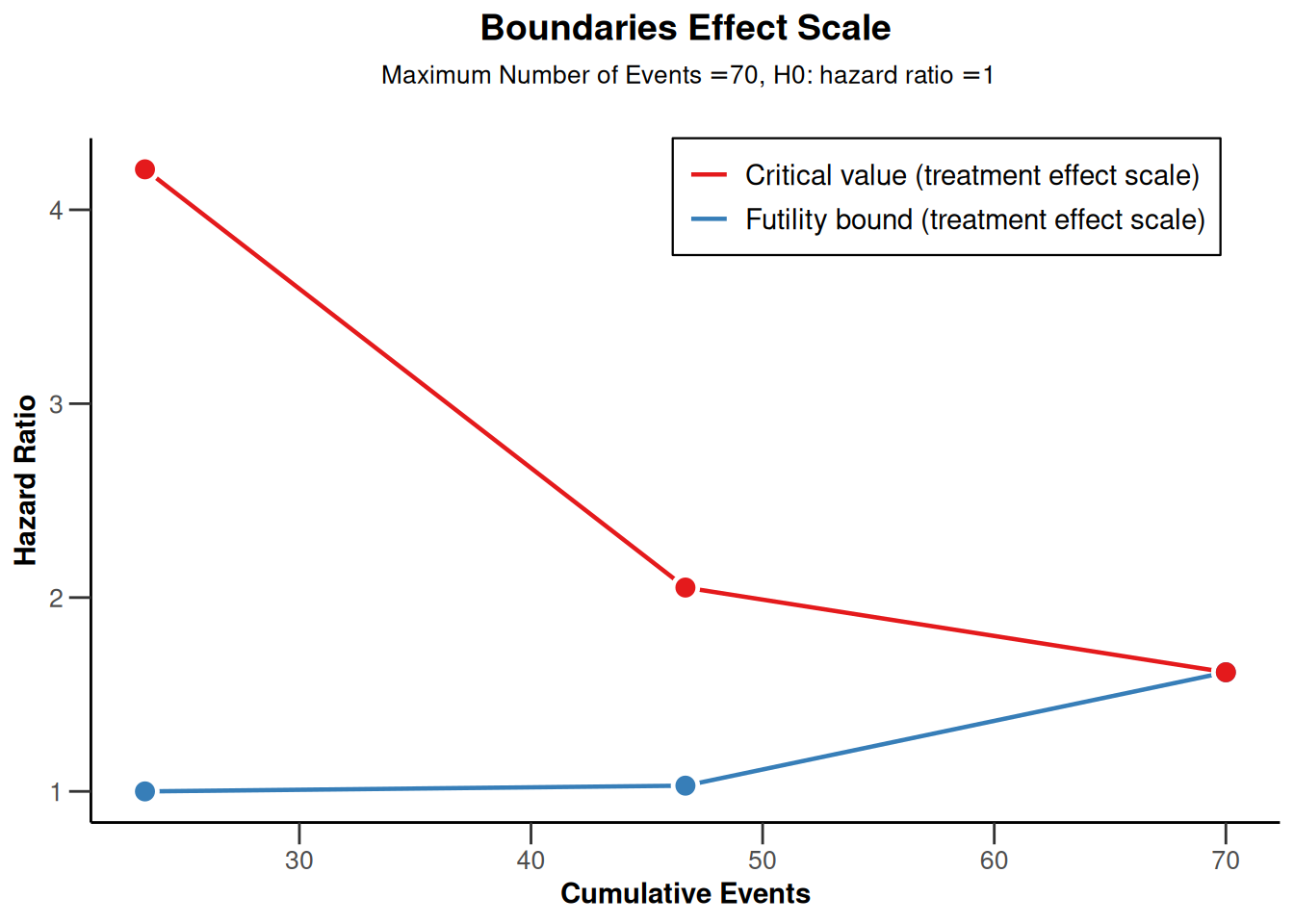``plot(powerSurvival2, type = 13, legendPosition = 1)``
``````Warning in !is.null(lambda1) && !is.na(lambda1): 'length(x) = 5 > 1' in
coercion to 'logical(1)'

Warning in !is.null(lambda1) && !is.na(lambda1): 'length(x) = 5 > 1' in
coercion to 'logical(1)'``````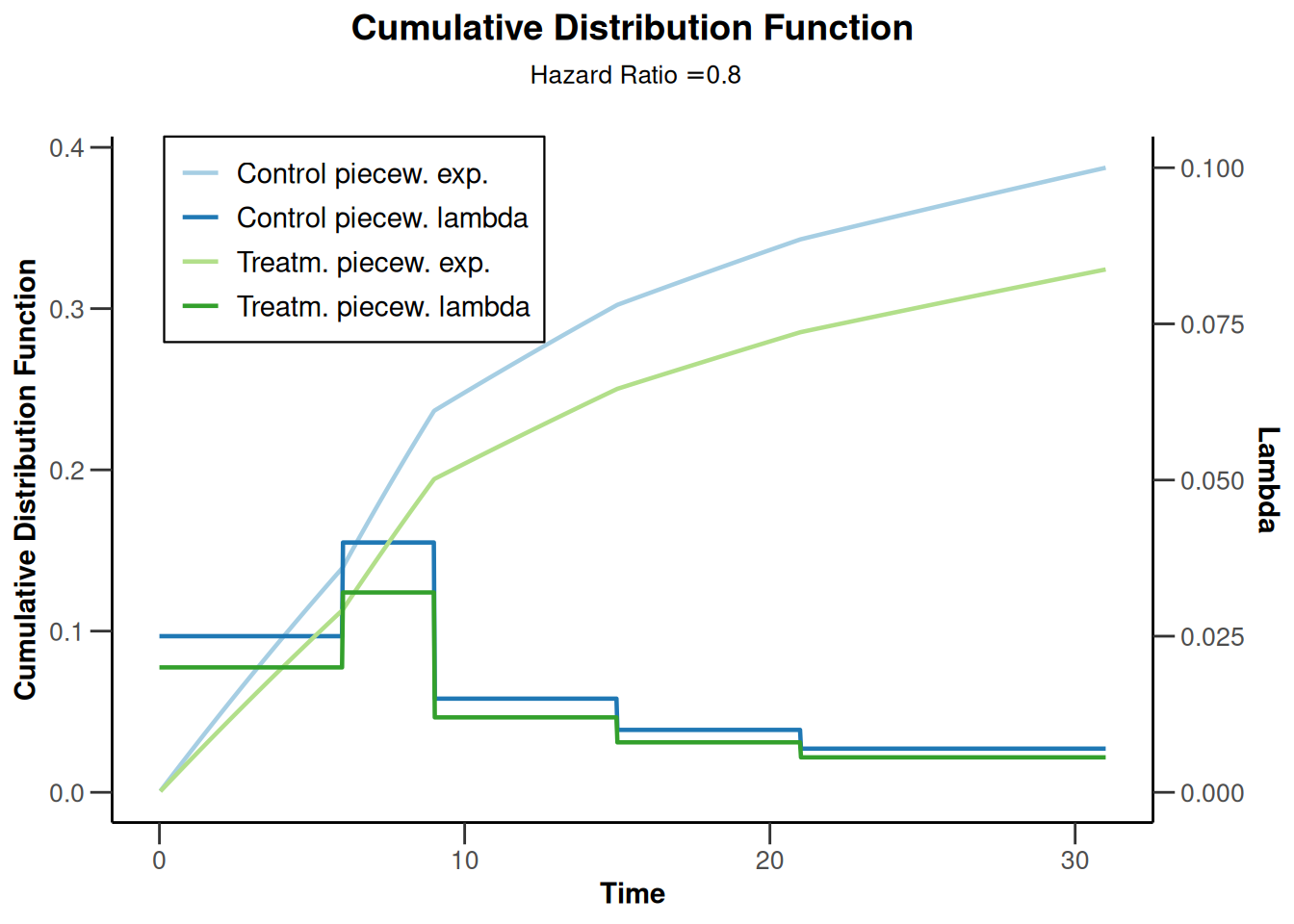``plot(powerSurvival2, type = 14)``
``````Warning in !is.null(lambda1) && !is.na(lambda1): 'length(x) = 5 > 1' in
coercion to 'logical(1)'

Warning in !is.null(lambda1) && !is.na(lambda1): 'length(x) = 5 > 1' in
coercion to 'logical(1)'``````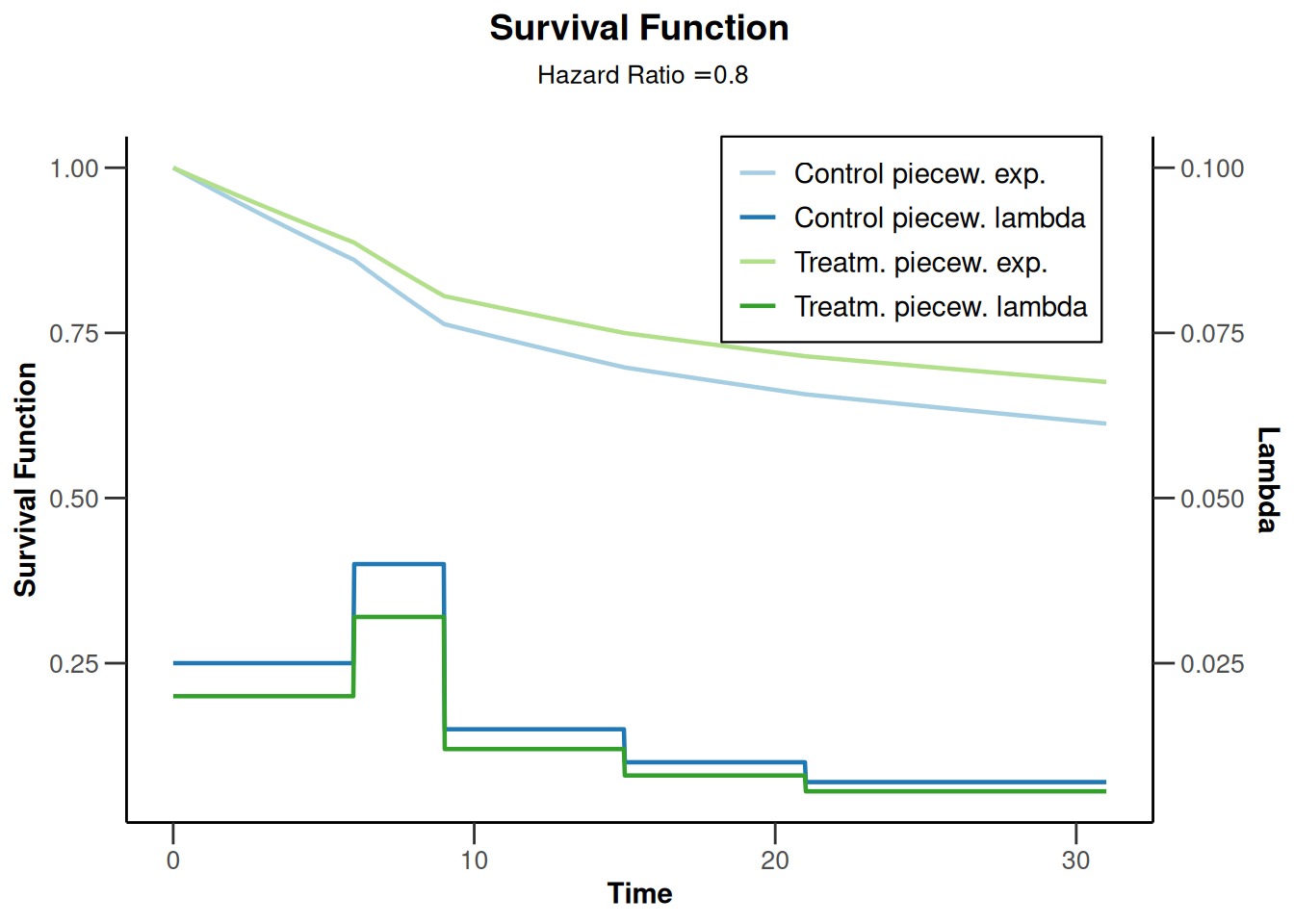``````design <- getDesignGroupSequential(
typeOfDesign = "OF", sided = 1,
futilityBounds = c(0, 0.1)
)

powerSurvival3 <- getPowerSurvival(
design = design,
typeOfComputation = "Schoenfeld", thetaH0 = 1,
allocationRatioPlanned = 1, kappa = 1,
piecewiseSurvivalTime = c(0, 5, 10),
lambda2 = c(0.025, 0.04, 0.015),
lambda1 = c(0.02, 0.032, 0.012),
maxNumberOfSubjects = 2480, maxNumberOfEvents = 70
)

plot(powerSurvival3, type = 1)````````plot(powerSurvival3, type = 2)````plot(powerSurvival3, type = 13, legendPosition = 1)``
``````Warning in !is.null(lambda1) && !is.na(lambda1): 'length(x) = 3 > 1' in
coercion to 'logical(1)'

Warning in !is.null(lambda1) && !is.na(lambda1): 'length(x) = 3 > 1' in
coercion to 'logical(1)'``````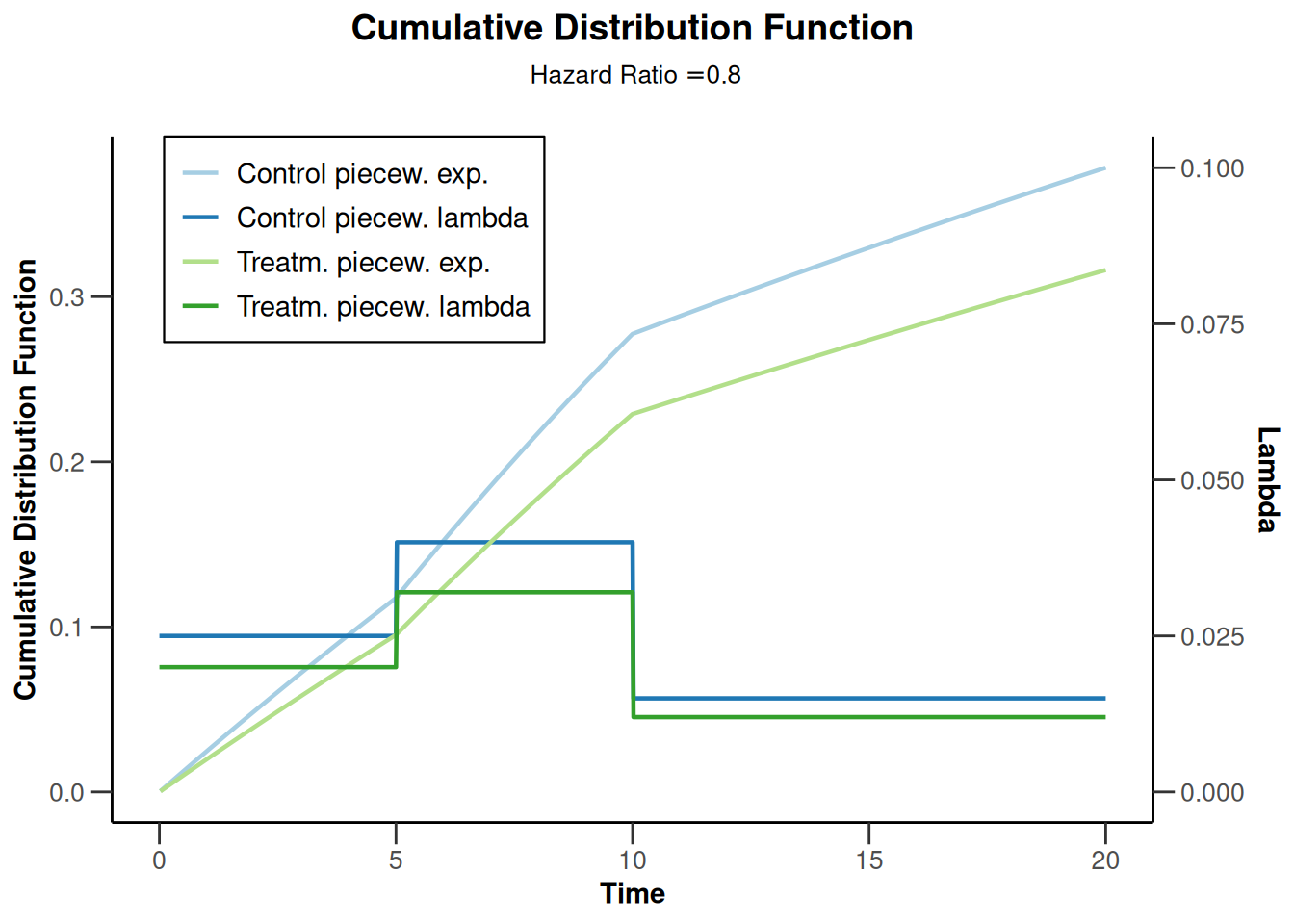``plot(powerSurvival2, type = 14)``
``````Warning in !is.null(lambda1) && !is.na(lambda1): 'length(x) = 5 > 1' in
coercion to 'logical(1)'``````
``````Warning in !is.null(lambda1) && !is.na(lambda1): 'length(x) = 5 > 1' in
coercion to 'logical(1)'``````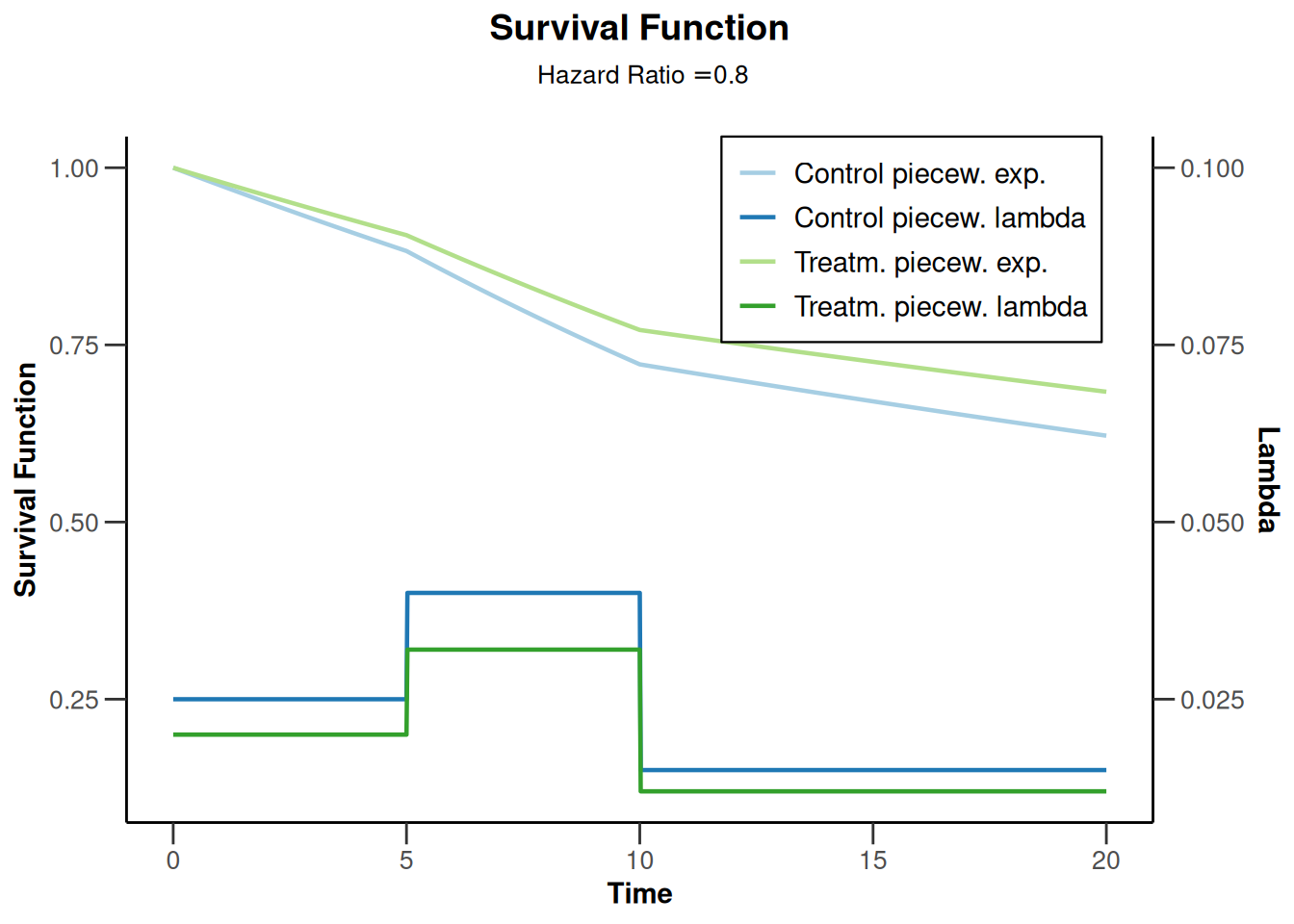### Power survival for one lambda

``````powerSurvival4 <- getPowerSurvival(
accrualTime = 12,
lambda2 = 0.04,
hazardRatio = 0.6,
maxNumberOfSubjects = 1400,
maxNumberOfEvents = 300
)

plot(powerSurvival4, type = 13, legendPosition = 1)``````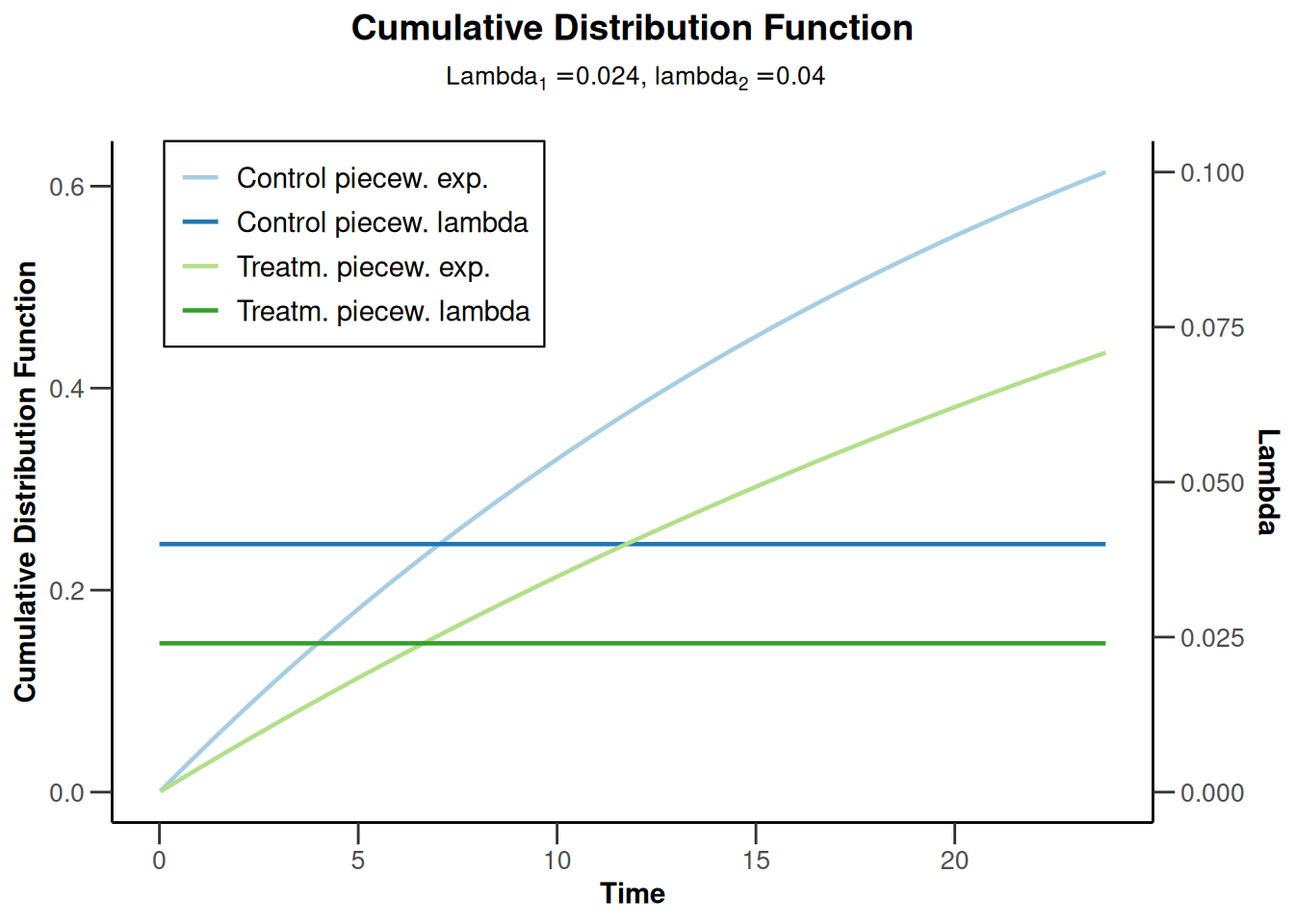``plot(powerSurvival4, type = 14, legendPosition = 5)``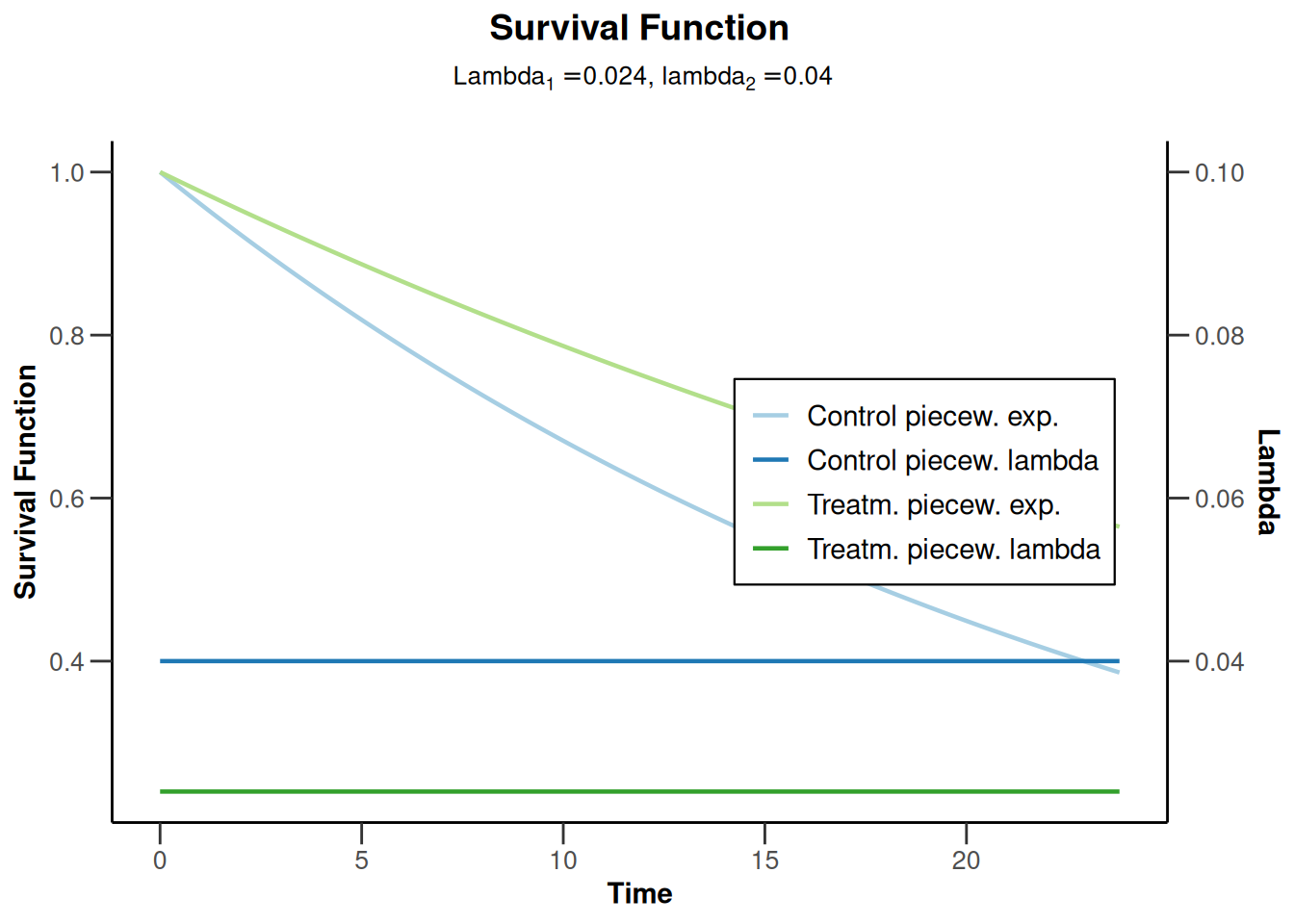### Power survival for default pi1 and pi2

``````powerSurvival5 <- getPowerSurvival(
maxNumberOfSubjects = 1400,
maxNumberOfEvents = 300
)

plot(powerSurvival5, type = 13, legendPosition = 1)``````
``Warning: Only the first 'pi1' (0.2) was used for plotting``
``````Warning in !is.na(designPlan\$pi1) && !is.na(designPlan\$pi2): 'length(x) = 4 >
1' in coercion to 'logical(1)'````````plot(powerSurvival5, type = 14, legendPosition = 5)``
``````Warning: Only the first 'pi1' (0.2) was used for plotting

Warning: 'length(x) = 4 > 1' in coercion to 'logical(1)'``````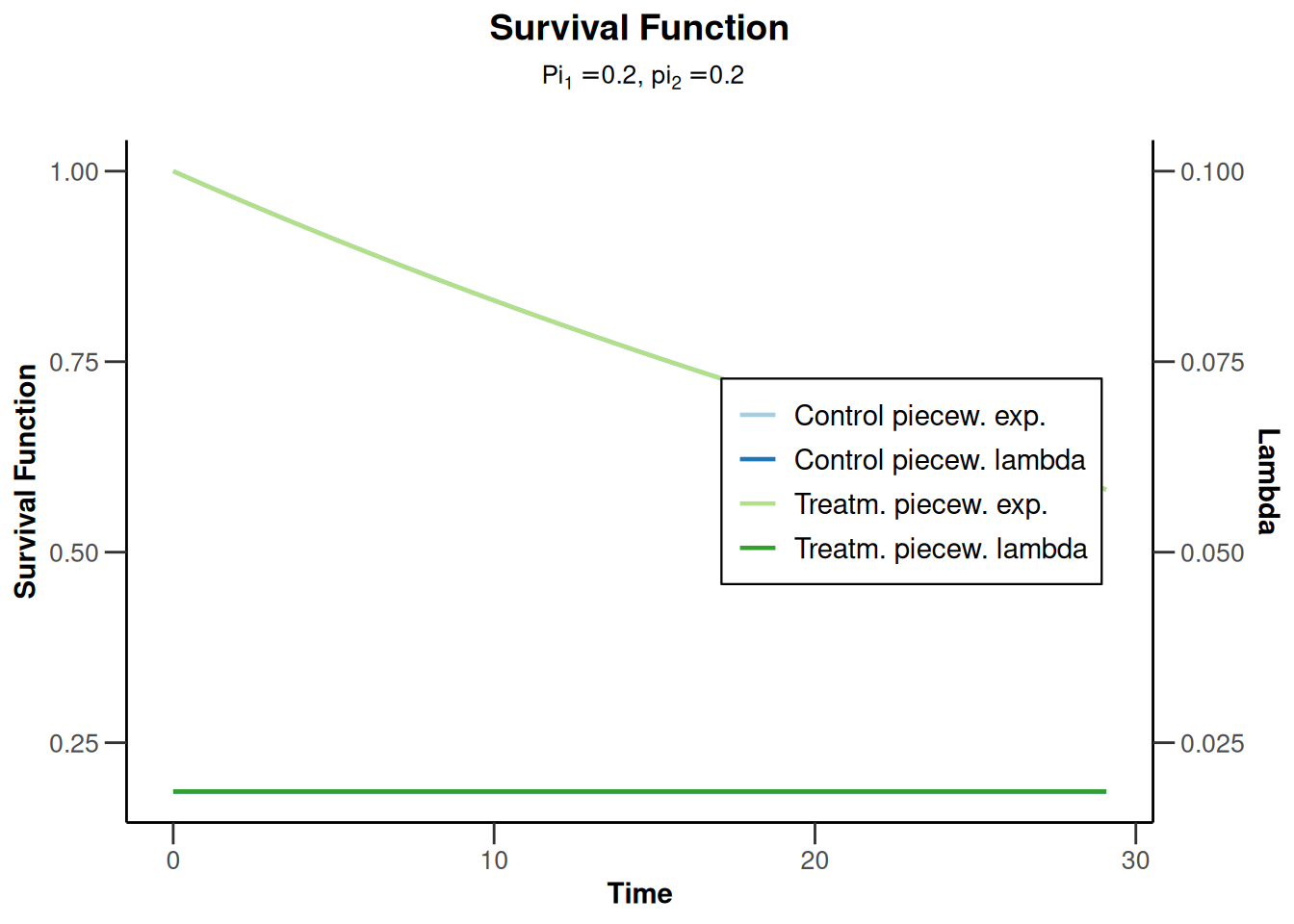# Simulation plots

## Simulation means (continuous endpoint)

``````simulationResults1 <- getSimulationMeans(
design = getDesignFisher(kMax = 2),
plannedSubjects = c(20, 40), maxNumberOfIterations = 1000,
seed = 12345
)

plot(simulationResults1, type = "all", grid = 0)``````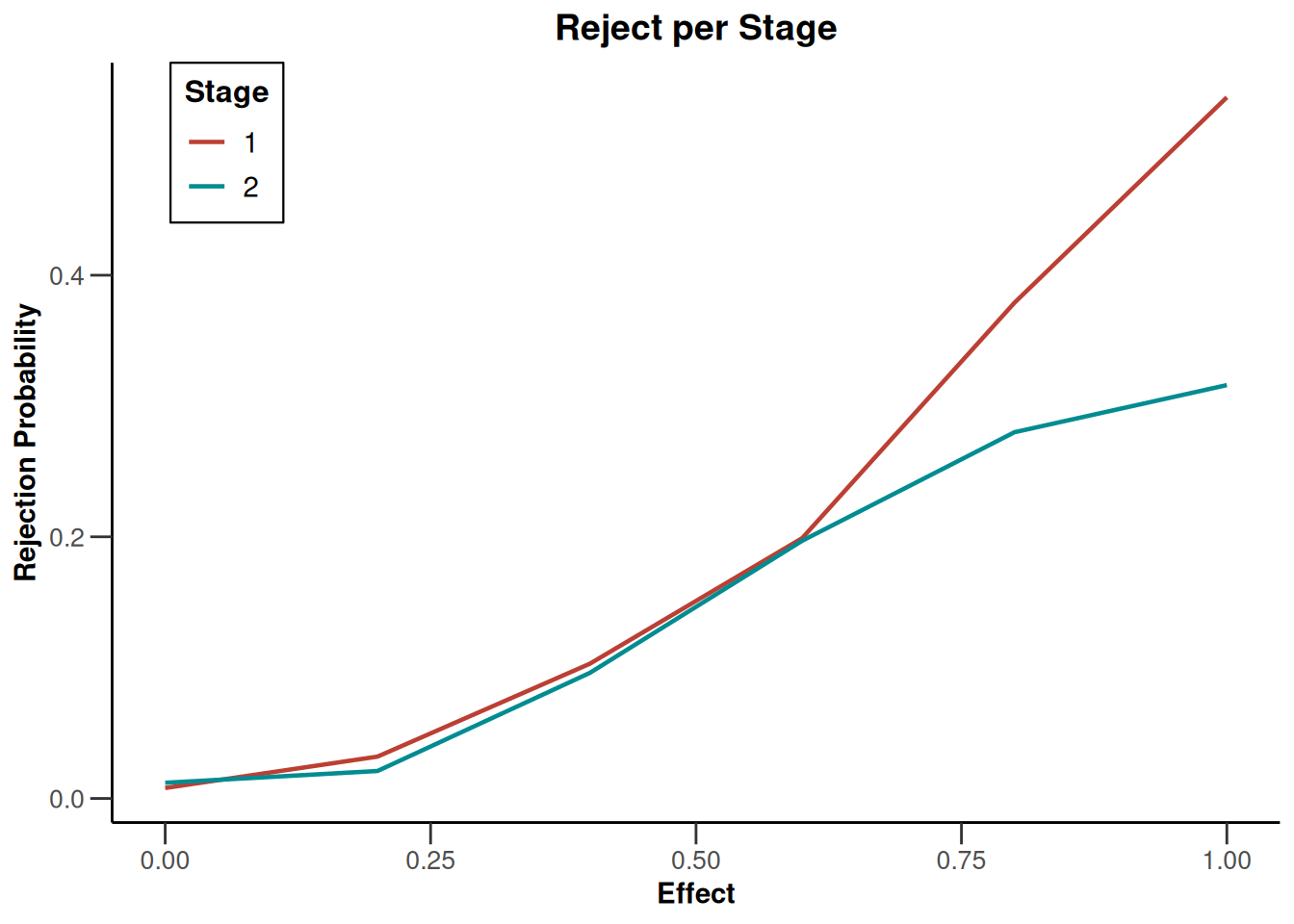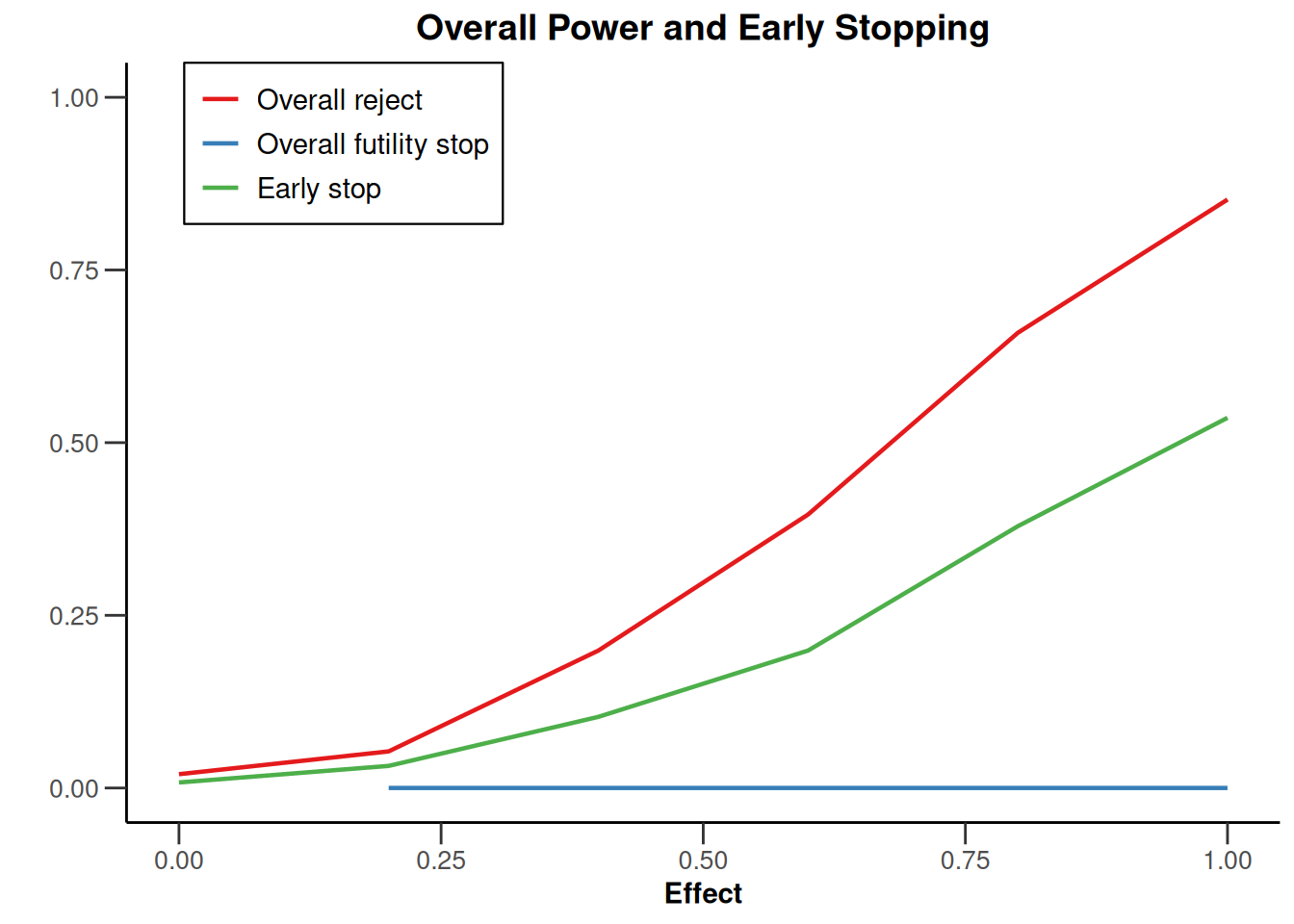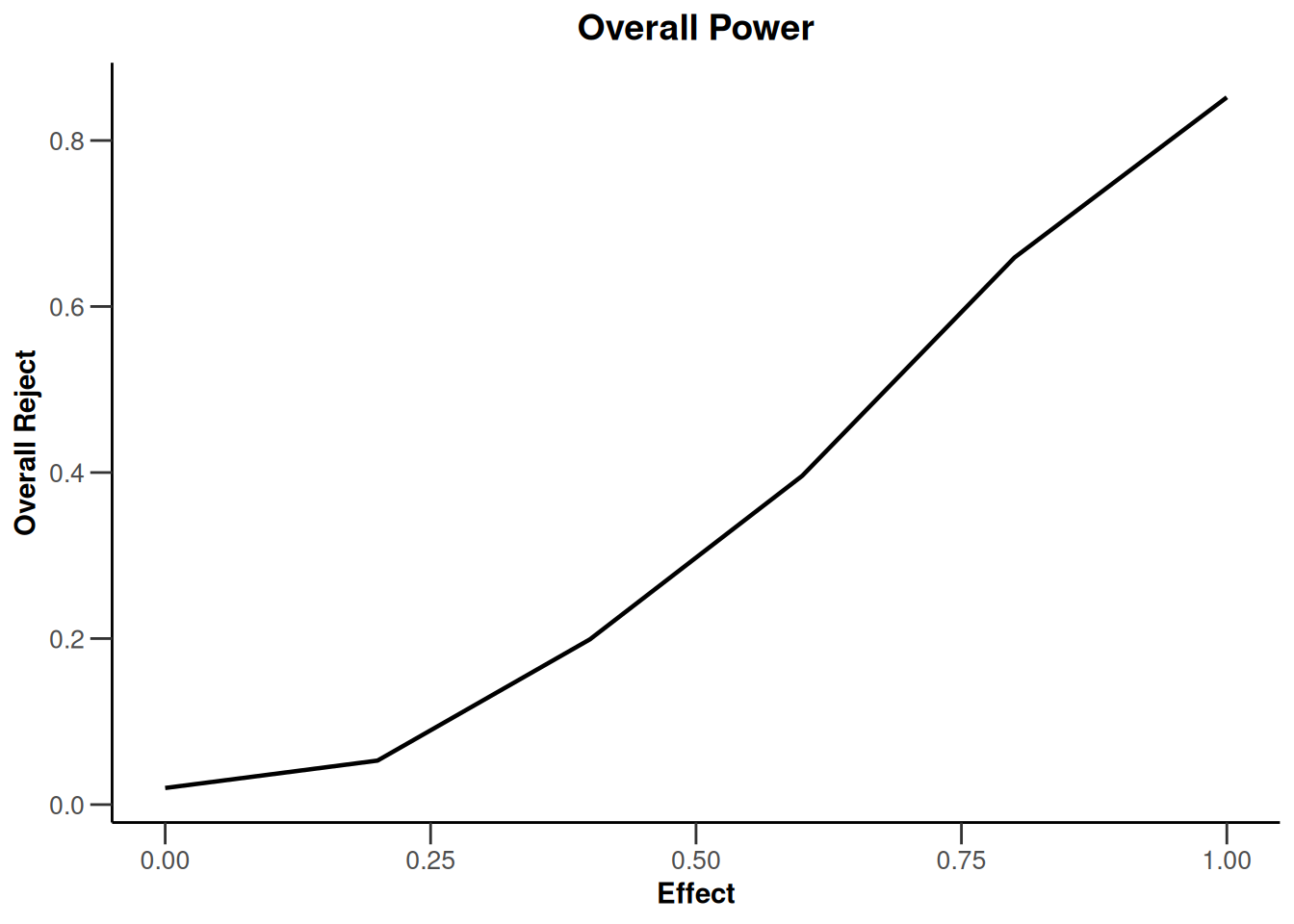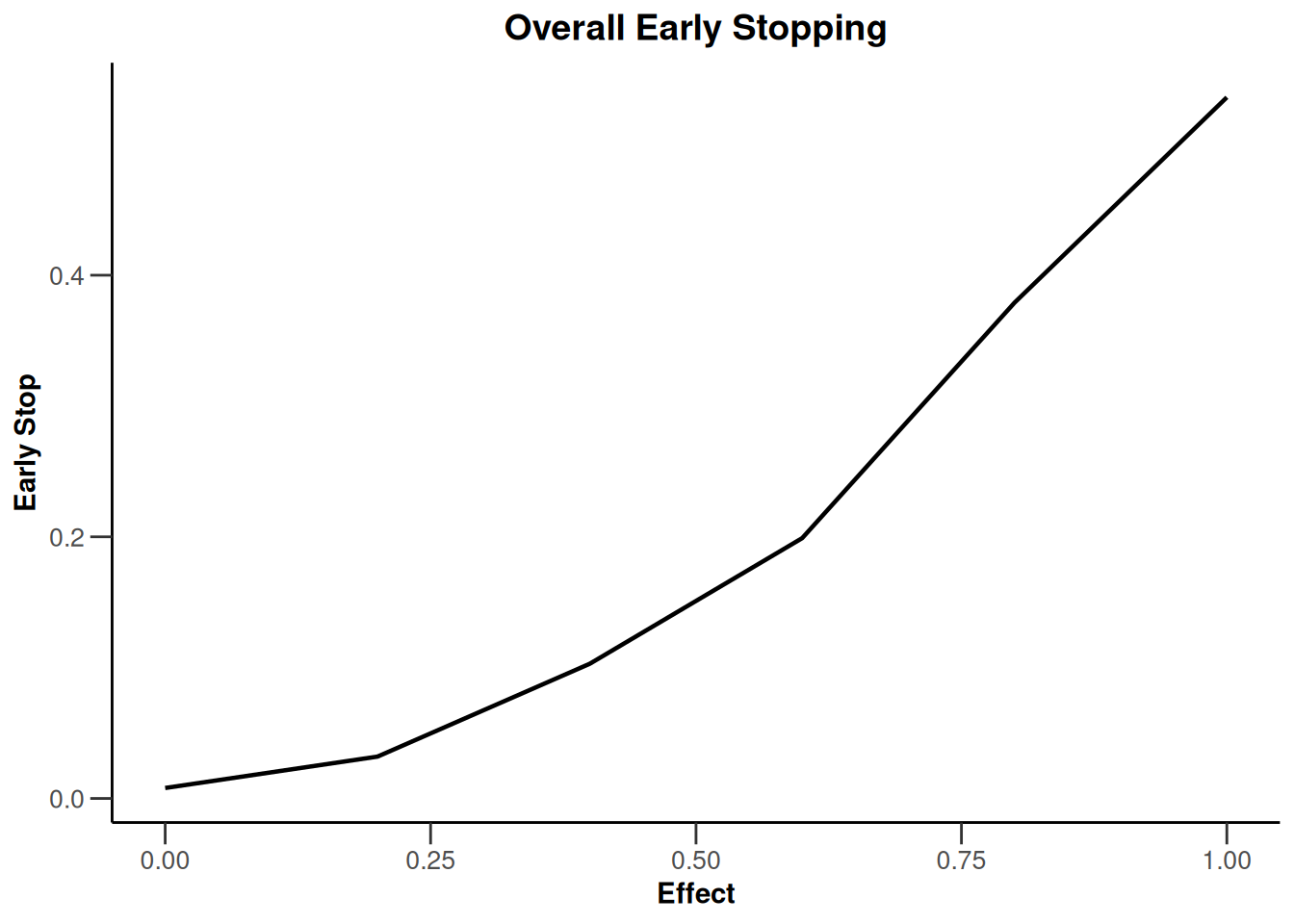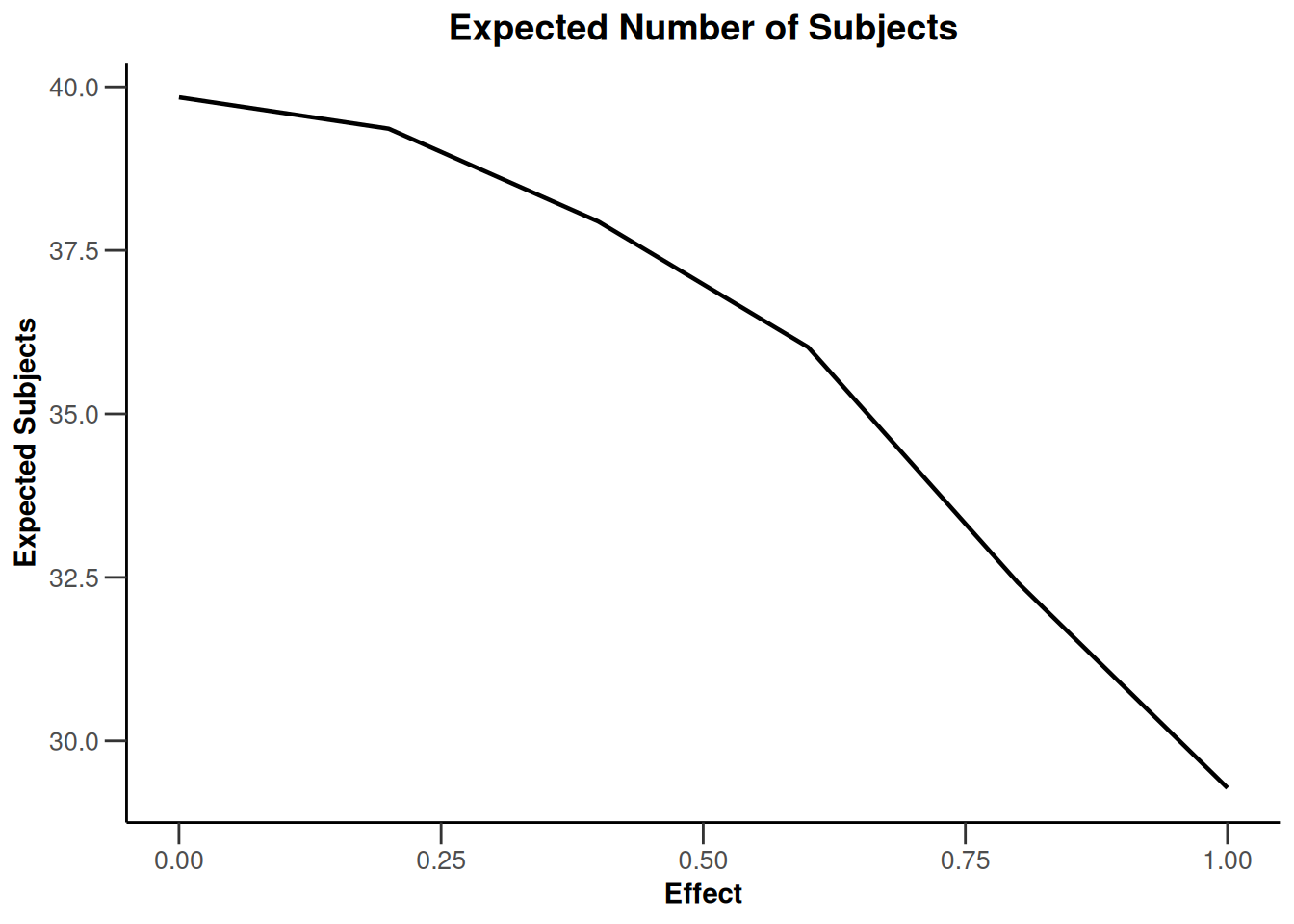``plot(simulationResults1, type = 4)````plot(simulationResults1, type = 5)````plot(simulationResults1, type = 6)``## Simulation rates (binary endpoint)

``````simulationResults1 <- getSimulationRates(
design = getDesignFisher(kMax = 2),
plannedSubjects = c(20, 40), maxNumberOfIterations = 1000,
seed = 12345
)

plot(simulationResults1, type = 4)``````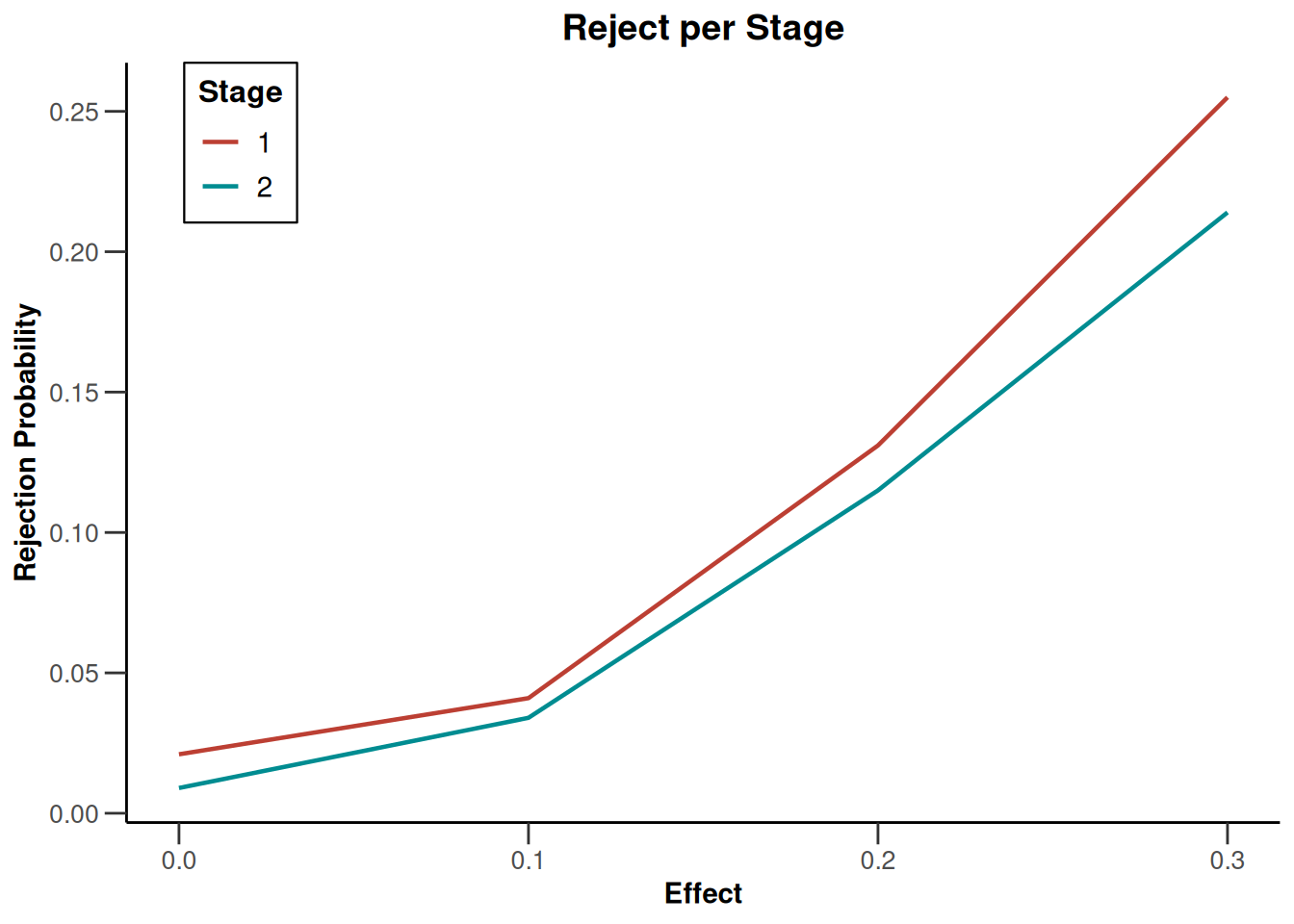``plot(simulationResults1, type = 5)``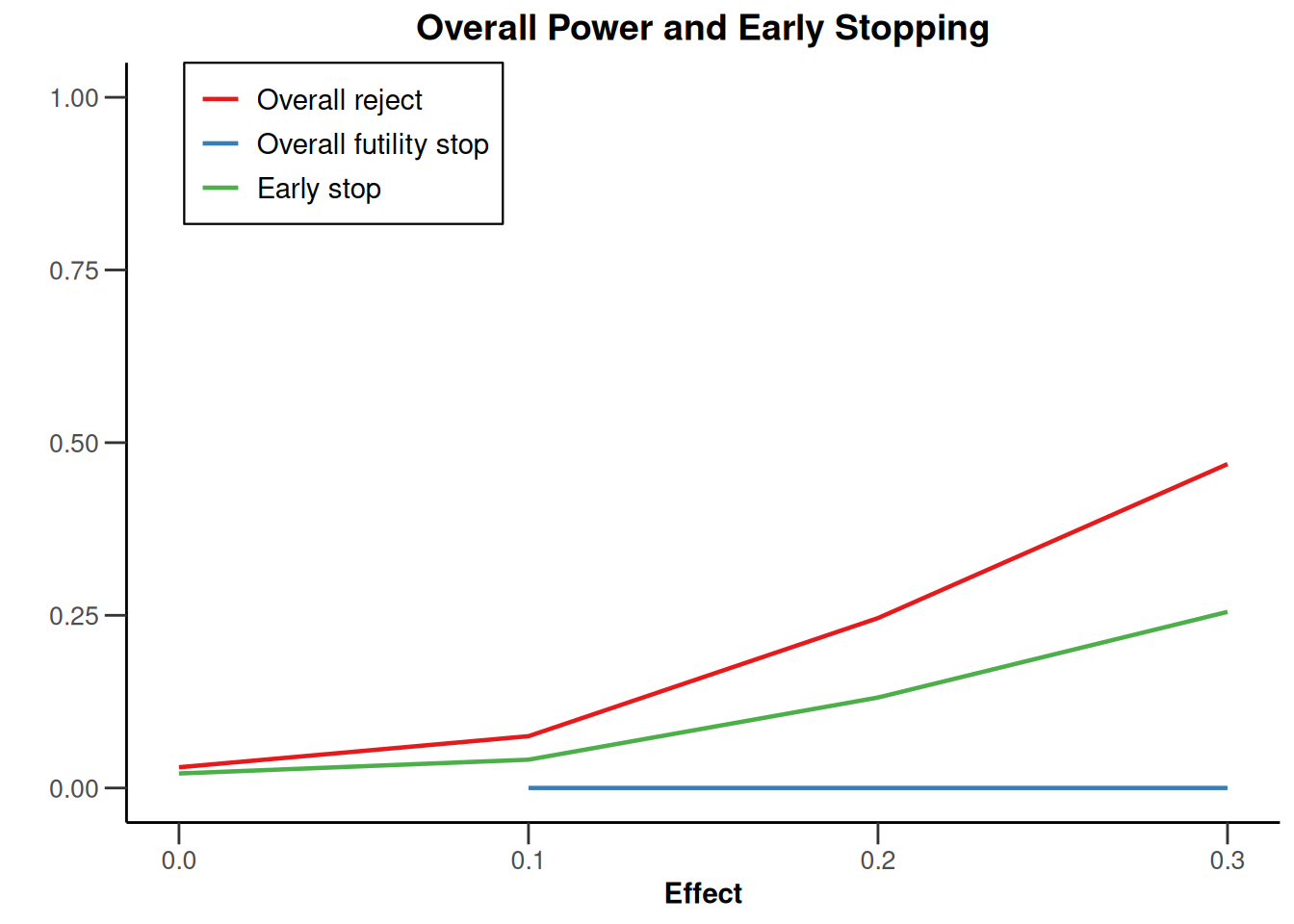``plot(simulationResults1, type = 6)``## Simulation plots survival (survival endpoint)

``````simulationResults1 <- getSimulationSurvival(
accrualTime = 12,
maxNumberOfSubjects = 1405,
plannedEvents = 300, maxNumberOfIterations = 1000,
seed = 12345
)

plot(simulationResults1, type = 5)``````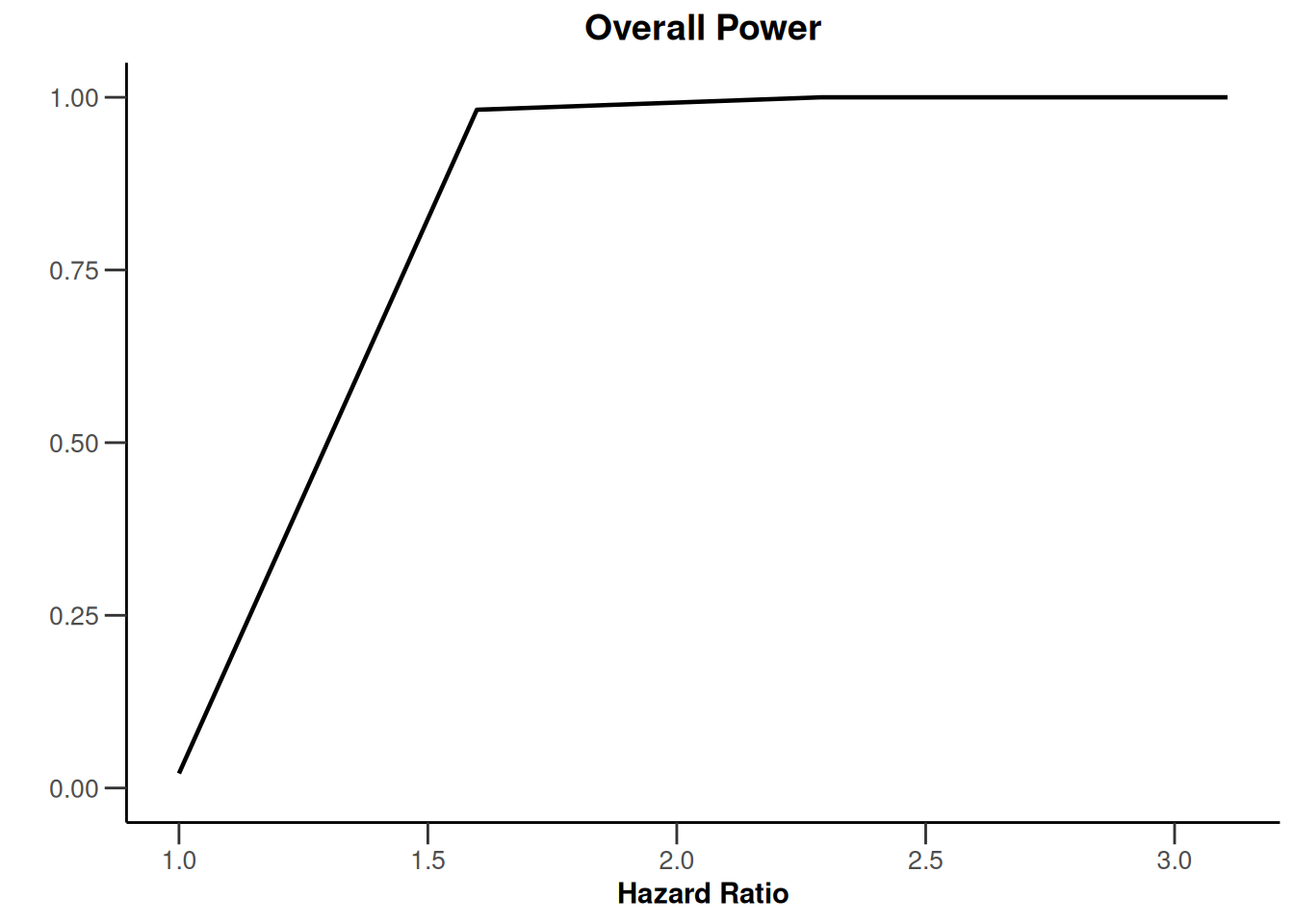``plot(simulationResults1, type = 6)````plot(simulationResults1, type = 7)````plot(simulationResults1, type = 9)``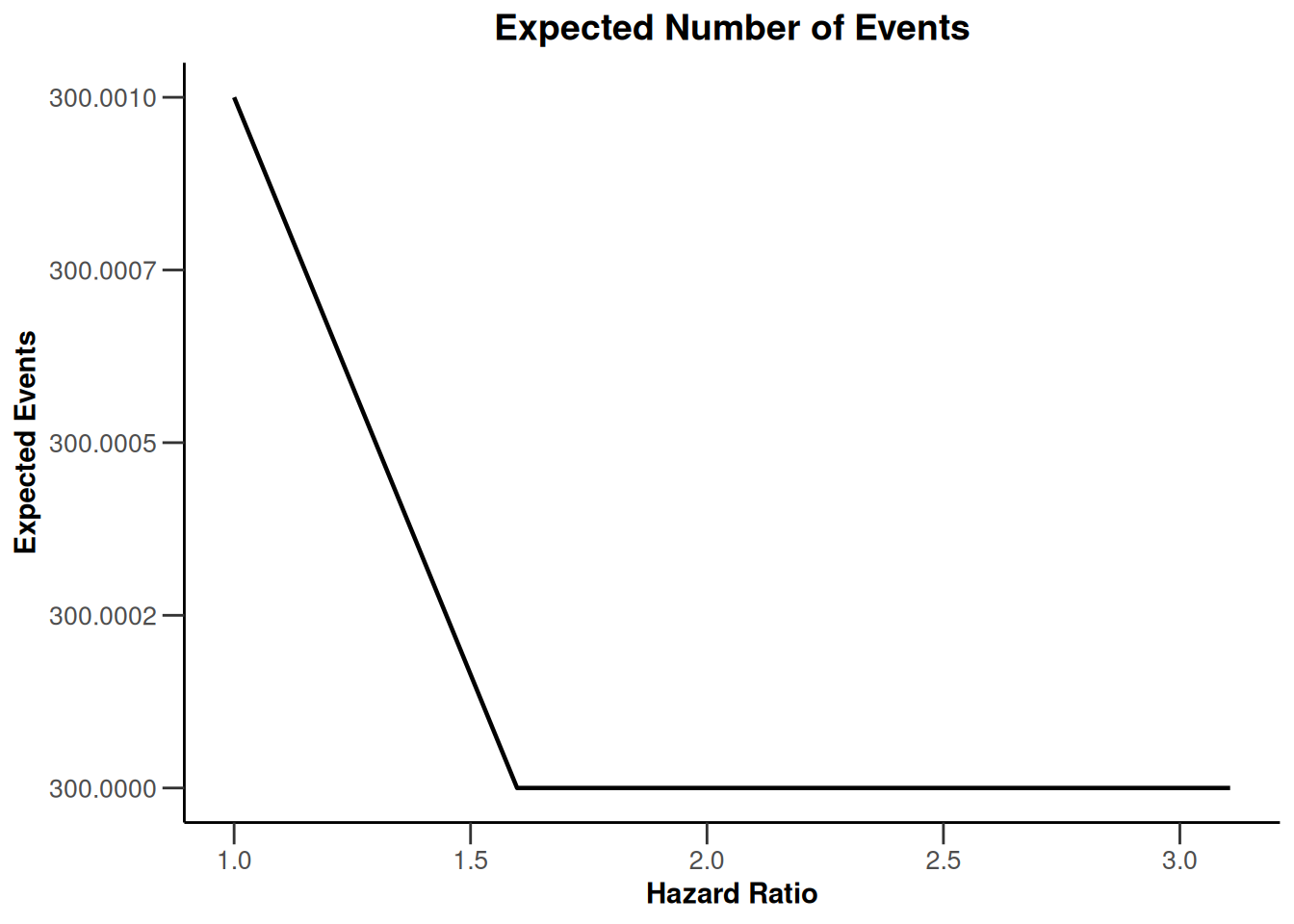``plot(simulationResults1, type = 10)``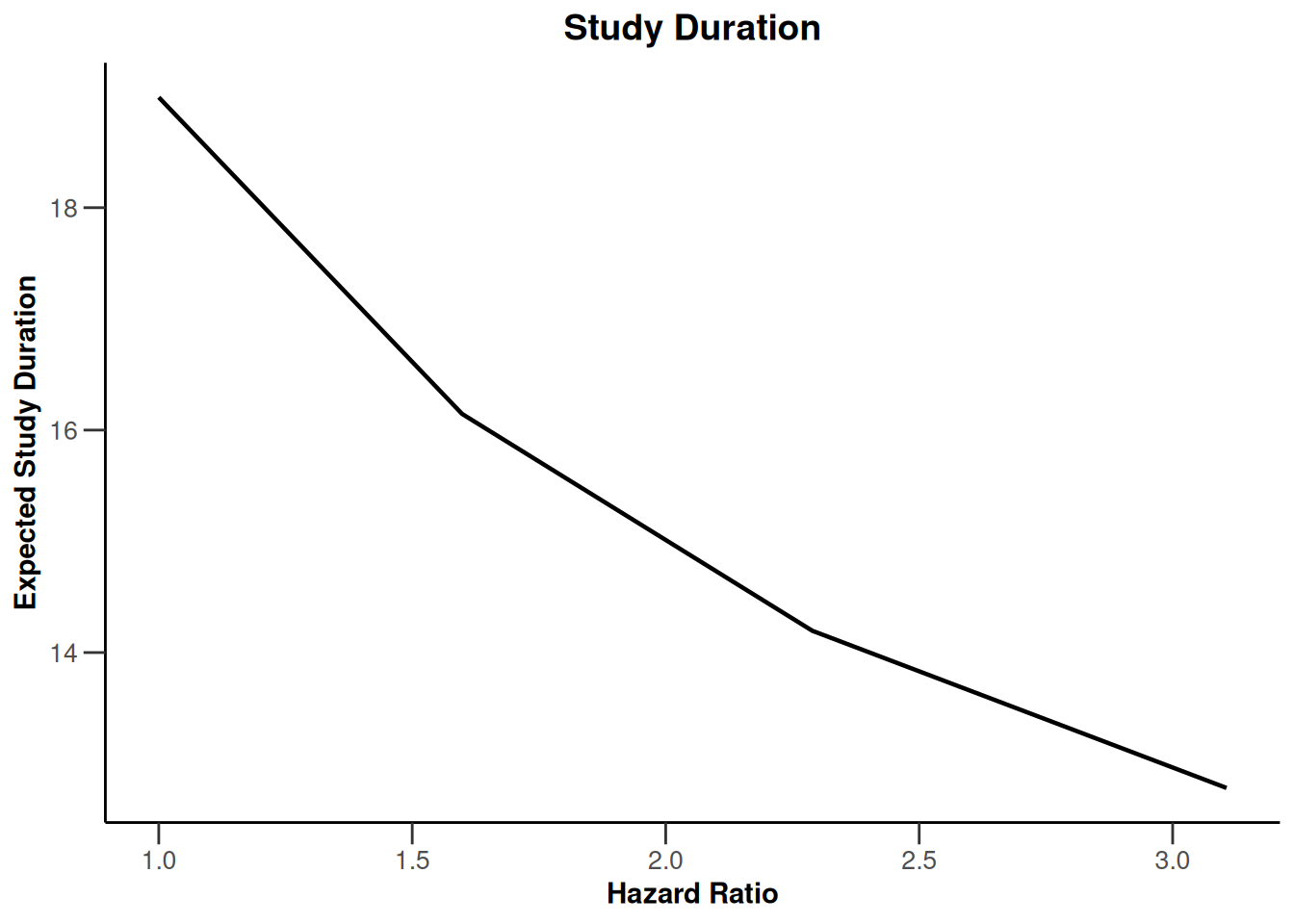``plot(simulationResults1, type = 11)``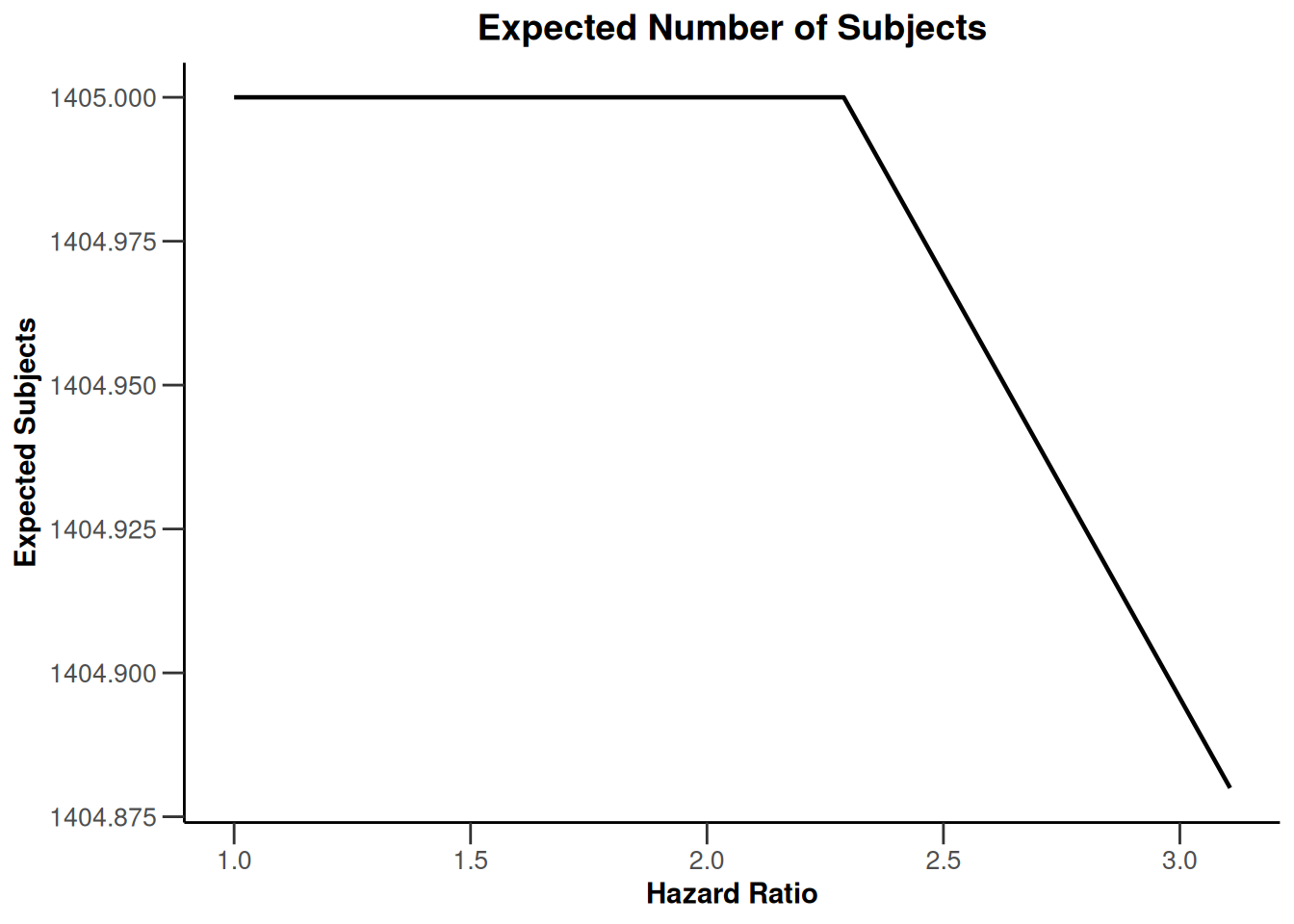``plot(simulationResults1, type = 12)``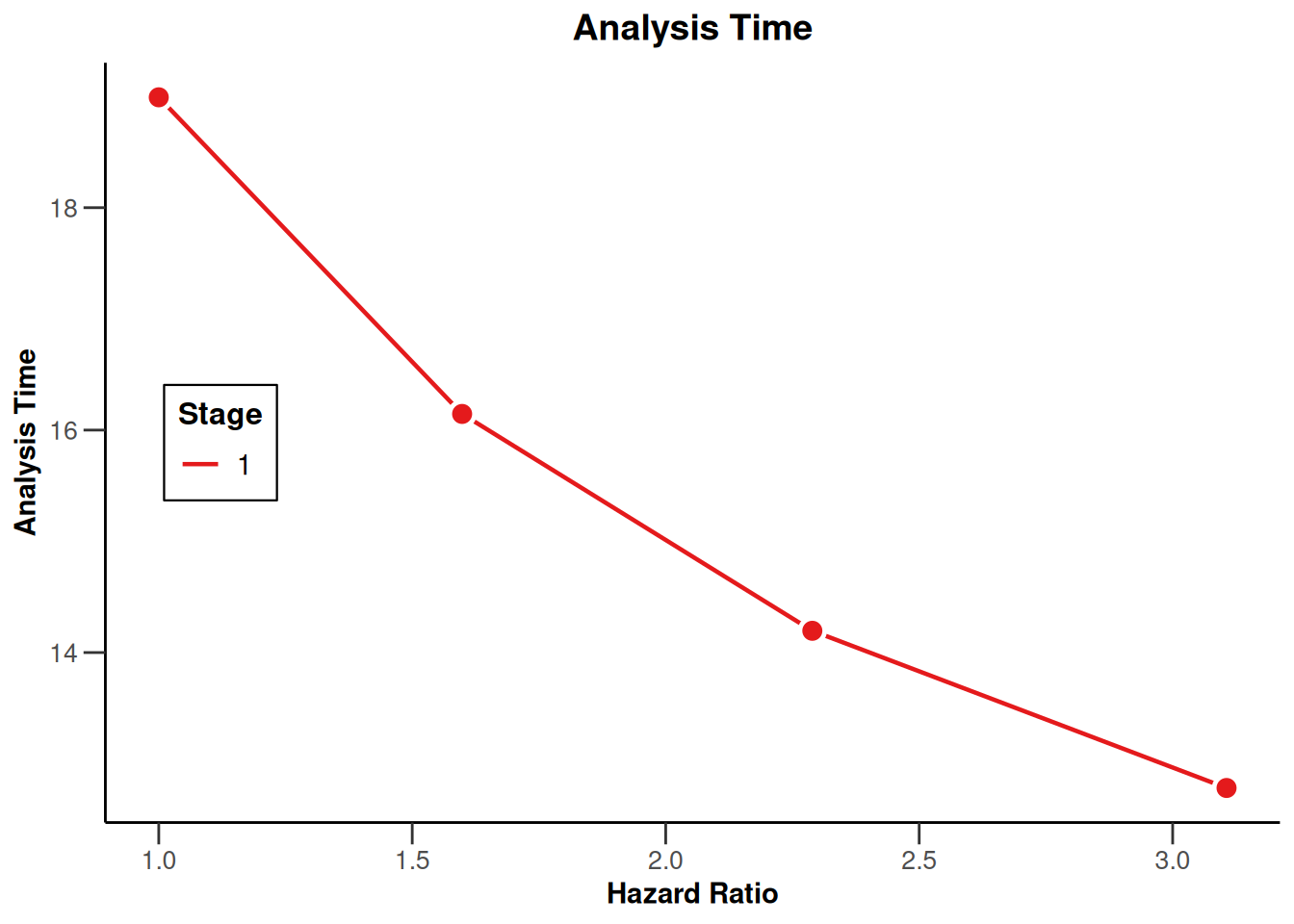``plot(simulationResults1, type = 13)``
``Warning: Only the first 'pi1' (0.2) was used for plotting``
``````Warning in !is.na(designPlan\$pi1) && !is.na(designPlan\$pi2): 'length(x) = 4 >
1' in coercion to 'logical(1)'``````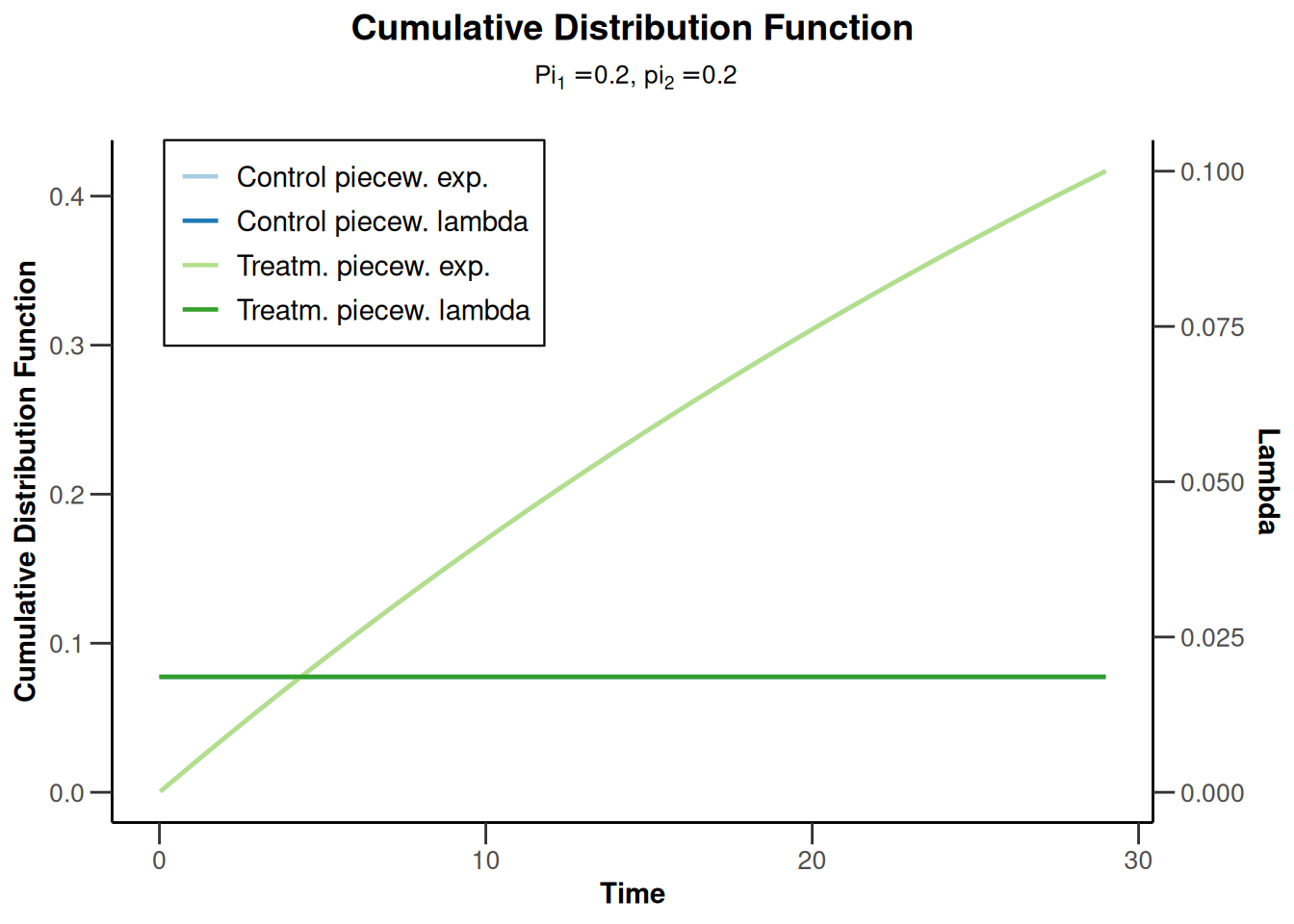``plot(simulationResults1, type = 14)``
``````Warning: Only the first 'pi1' (0.2) was used for plotting

Warning: 'length(x) = 4 > 1' in coercion to 'logical(1)'``````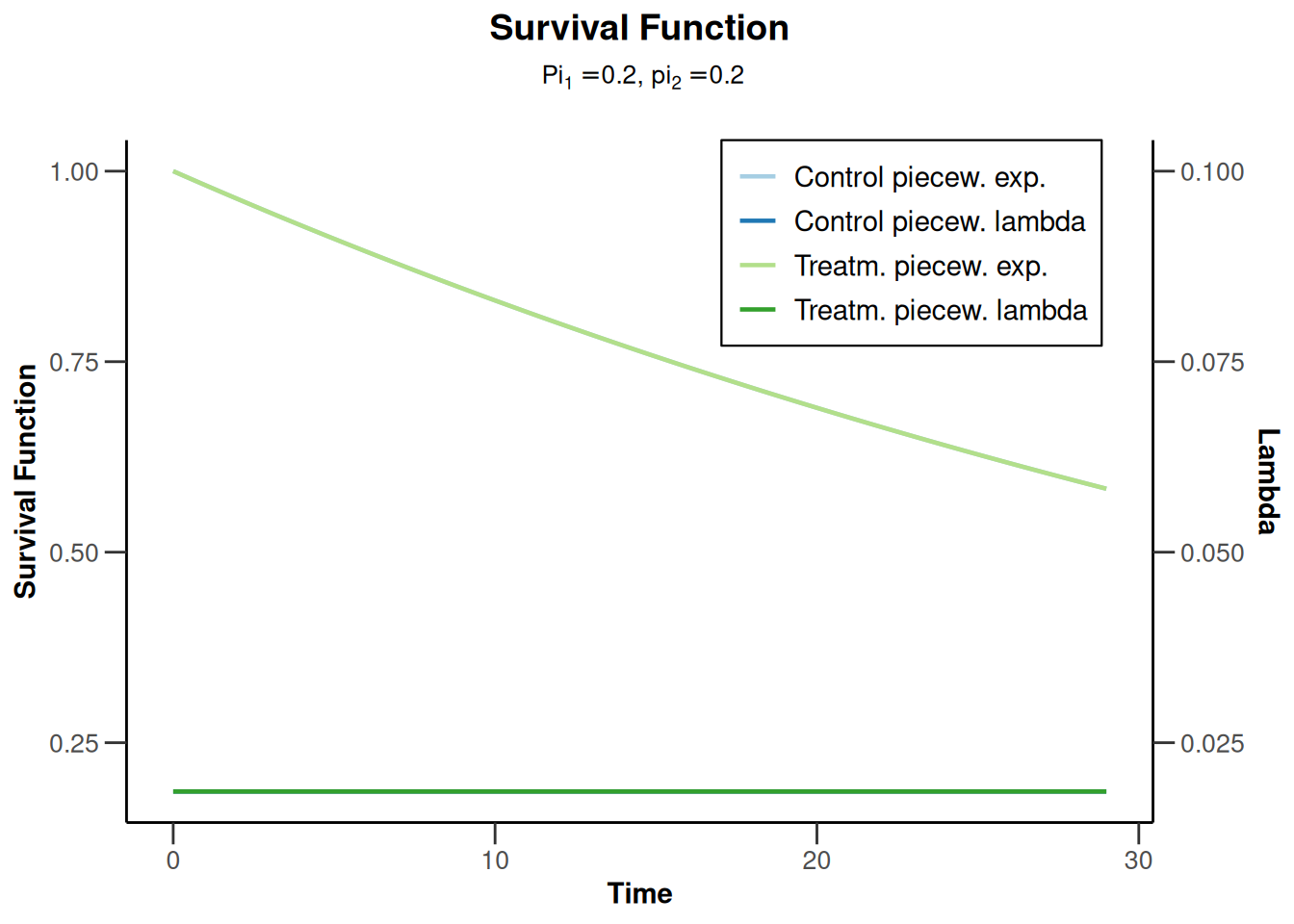``````simulationResults2 <- getSimulationSurvival(
accrualTime = 12,
lambda2 = 0.03,
hazardRatio = 0.8,
maxNumberOfSubjects = 1405,
plannedEvents = 300, maxNumberOfIterations = 1000,
seed = 23456
)

plot(simulationResults2, type = 13)``````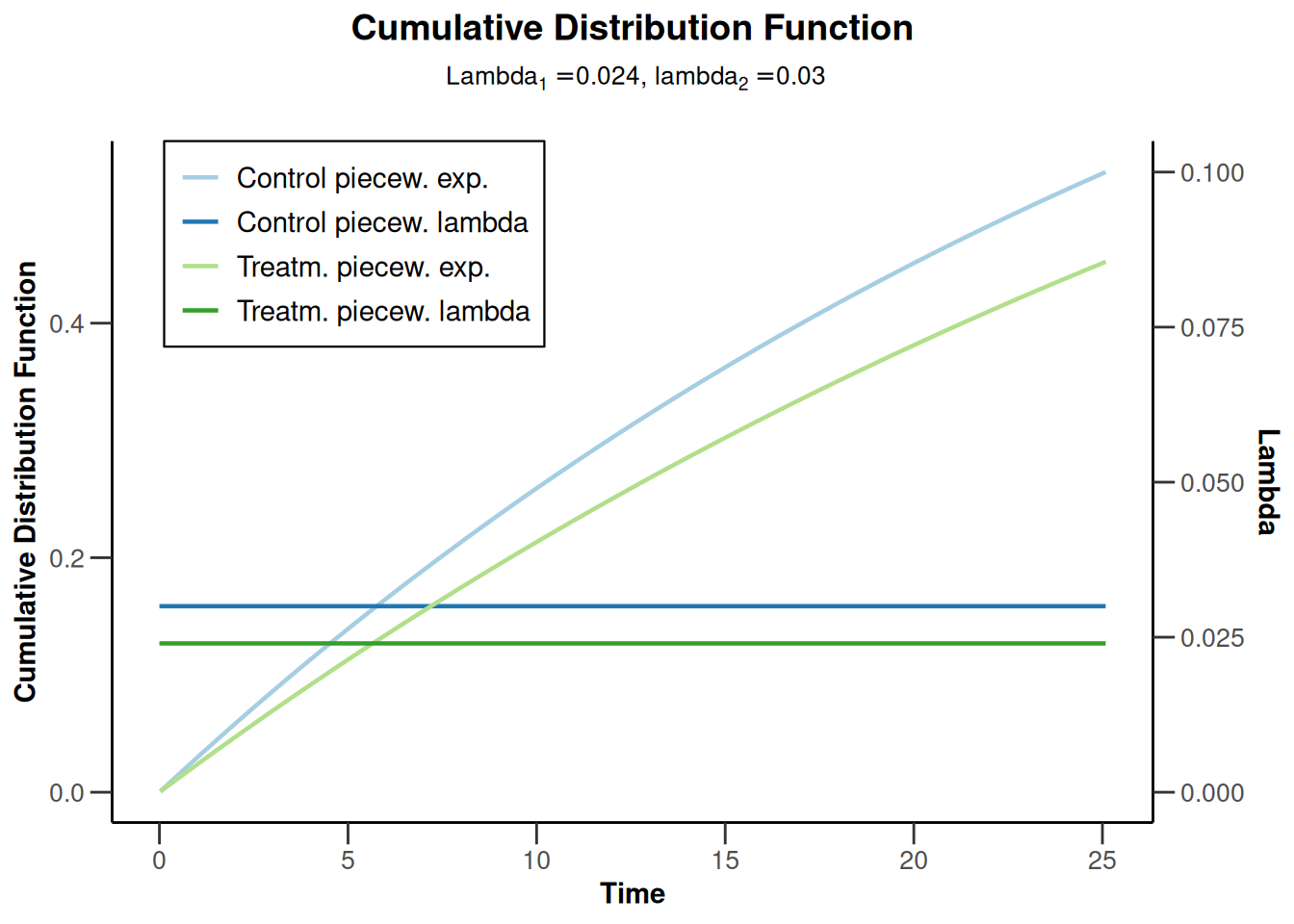``plot(simulationResults2, type = 14)``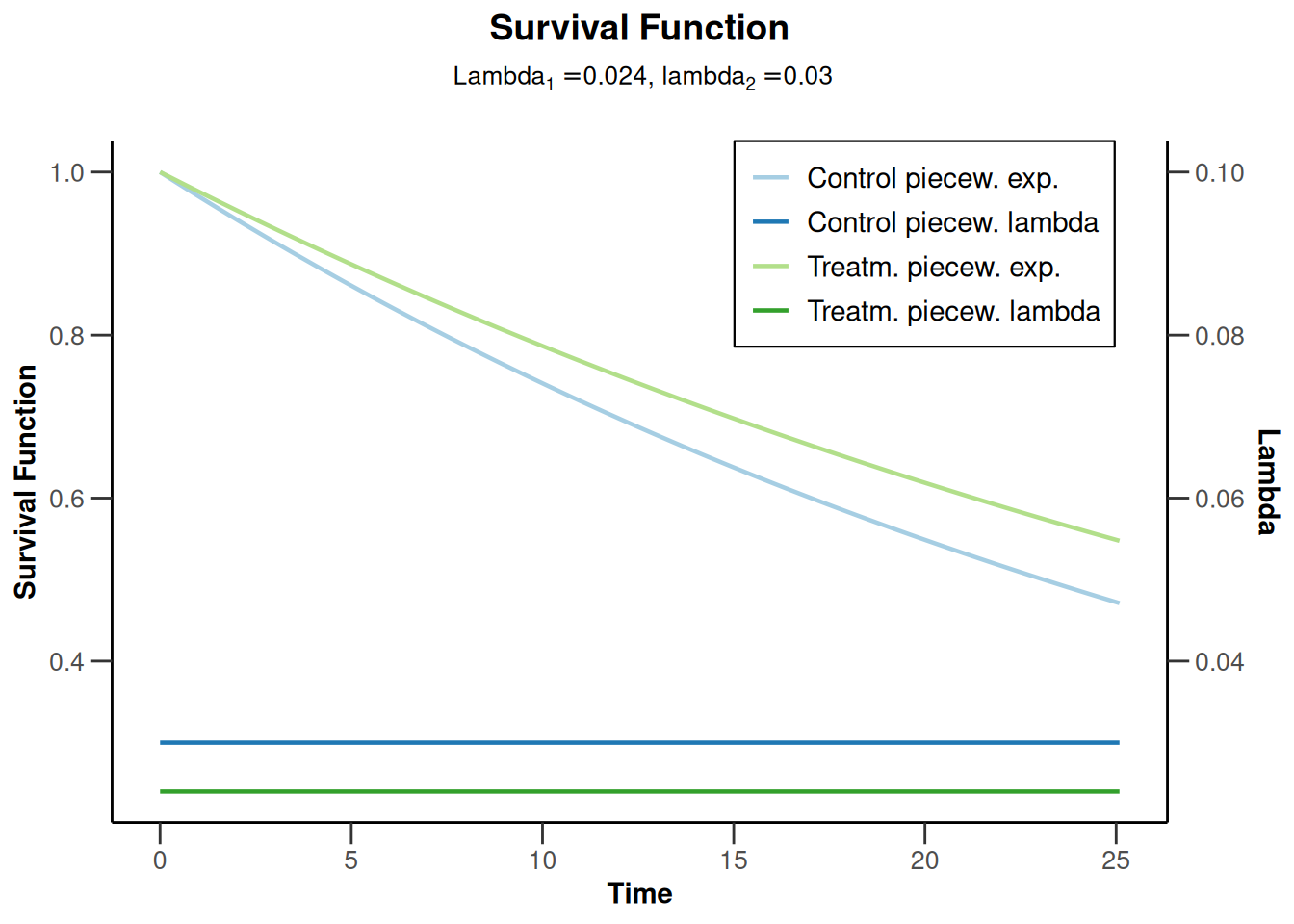System: rpact 3.3.4, R version 4.2.2 (2022-10-31 ucrt), platform: x86_64-w64-mingw32

To cite R in publications use:

R Core Team (2022). R: A language and environment for statistical computing. R Foundation for Statistical Computing, Vienna, Austria. URL https://www.R-project.org/.

To cite package ‘rpact’ in publications use:

Wassmer G, Pahlke F (2023). rpact: Confirmatory Adaptive Clinical Trial Design and Analysis. https://www.rpact.org, https://www.rpact.com, https://github.com/rpact-com/rpact.